Last updated: 2020-10-25

Checks: 7 0

Knit directory: r4ds_book/

This reproducible R Markdown analysis was created with workflowr (version 1.6.2). The Checks tab describes the reproducibility checks that were applied when the results were created. The Past versions tab lists the development history.

Great! Since the R Markdown file has been committed to the Git repository, you know the exact version of the code that produced these results.

Great job! The global environment was empty. Objects defined in the global environment can affect the analysis in your R Markdown file in unknown ways. For reproduciblity it’s best to always run the code in an empty environment.

The command set.seed(20200814) was run prior to running the code in the R Markdown file. Setting a seed ensures that any results that rely on randomness, e.g. subsampling or permutations, are reproducible.

Great job! Recording the operating system, R version, and package versions is critical for reproducibility.

Nice! There were no cached chunks for this analysis, so you can be confident that you successfully produced the results during this run.

Great job! Using relative paths to the files within your workflowr project makes it easier to run your code on other machines.

Great! You are using Git for version control. Tracking code development and connecting the code version to the results is critical for reproducibility.

The results in this page were generated with repository version 57f23a8. See the Past versions tab to see a history of the changes made to the R Markdown and HTML files.

Note that you need to be careful to ensure that all relevant files for the analysis have been committed to Git prior to generating the results (you can use wflow_publish or wflow_git_commit). workflowr only checks the R Markdown file, but you know if there are other scripts or data files that it depends on. Below is the status of the Git repository when the results were generated:


Ignored files:
Ignored:    .Rproj.user/

Untracked files:
Untracked:  VideoDecodeStats/
Untracked:  analysis/images/
Untracked:  code_snipp.txt



Note that any generated files, e.g. HTML, png, CSS, etc., are not included in this status report because it is ok for generated content to have uncommitted changes.

These are the previous versions of the repository in which changes were made to the R Markdown (analysis/ch5_eda.Rmd) and HTML (docs/ch5_eda.html) files. If you’ve configured a remote Git repository (see ?wflow_git_remote), click on the hyperlinks in the table below to view the files as they were in that past version.

File Version Author Date Message
html 570c0bb sciencificity 2020-10-22 Build site.
html cfbefe6 sciencificity 2020-10-21 Build site.
html 4497db4 sciencificity 2020-10-18 Build site.
html 1a3bebe sciencificity 2020-10-18 Build site.
Rmd 03b054a sciencificity 2020-10-18 added Chapter 7
html ce8c214 sciencificity 2020-10-16 Build site.
Rmd 50bf4b7 sciencificity 2020-10-16 added new chapters

options(scipen=10000)
library(tidyverse)
library(flair)
library(nycflights13)
library(palmerpenguins)
library(gt)
library(skimr)
library(emo)
library(tidyquant)
library(lubridate)
library(magrittr)
theme_set(theme_tq())

## Patterns

• Variation: Best way to understand the pattern in a variable is to visualise the distribution of the variables values.

• Categorical: Bar chart

• Continuous: Histogram

• In both:

• tall bars == common values of the variable
• short bars == less common values of the variable
• no bars == absense of that value in your data

### Investigate Categories

ggplot(data = penguins) +  geom_bar(aes(x = species))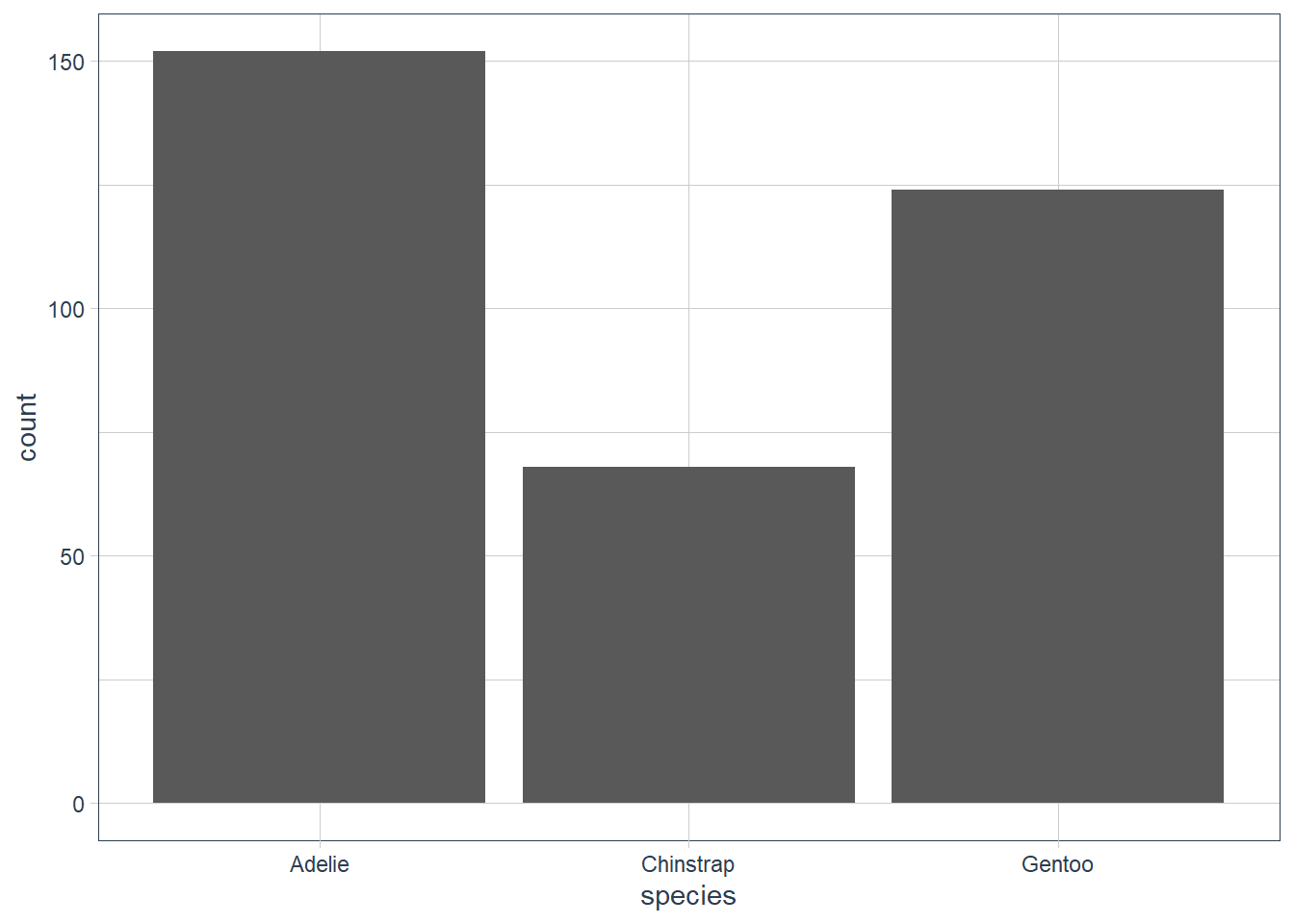ggplot(data = penguins) +  geom_bar(aes(x = sex))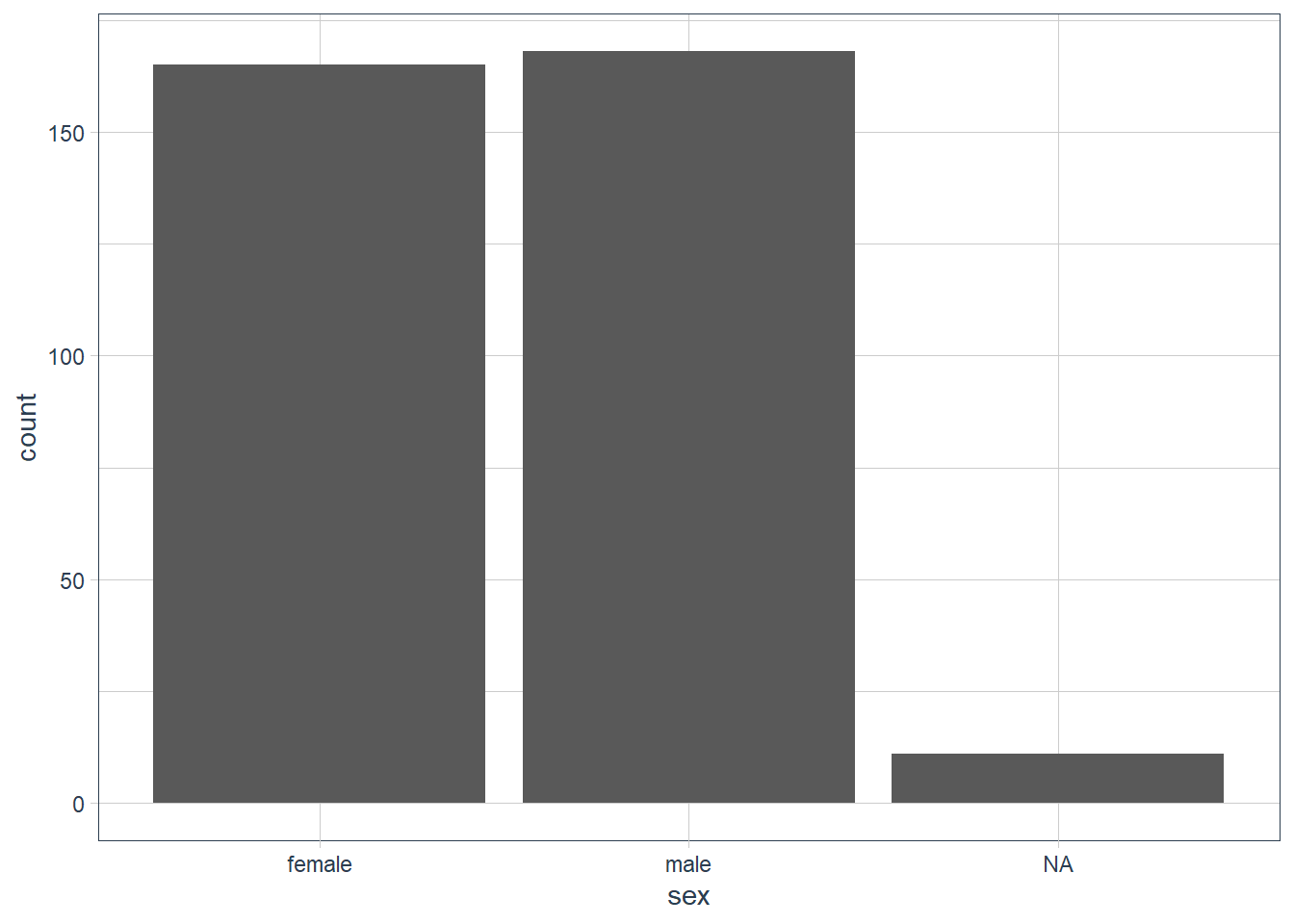ggplot(data = penguins) +  geom_bar(aes(x = island))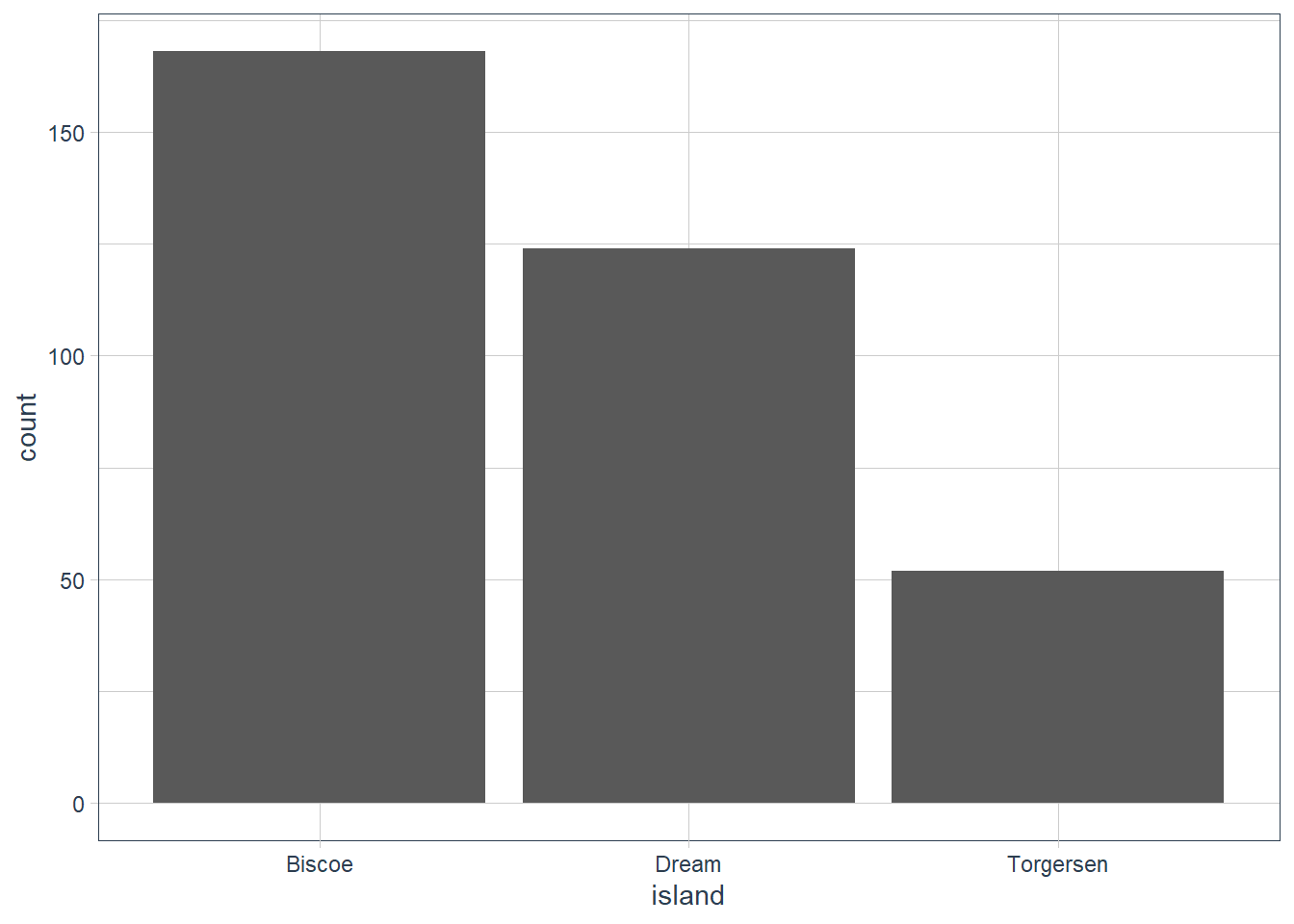### Investigate Continuous Data

ggplot(data = penguins) +  geom_histogram(aes(x = bill_length_mm),                  binwidth = 0.6)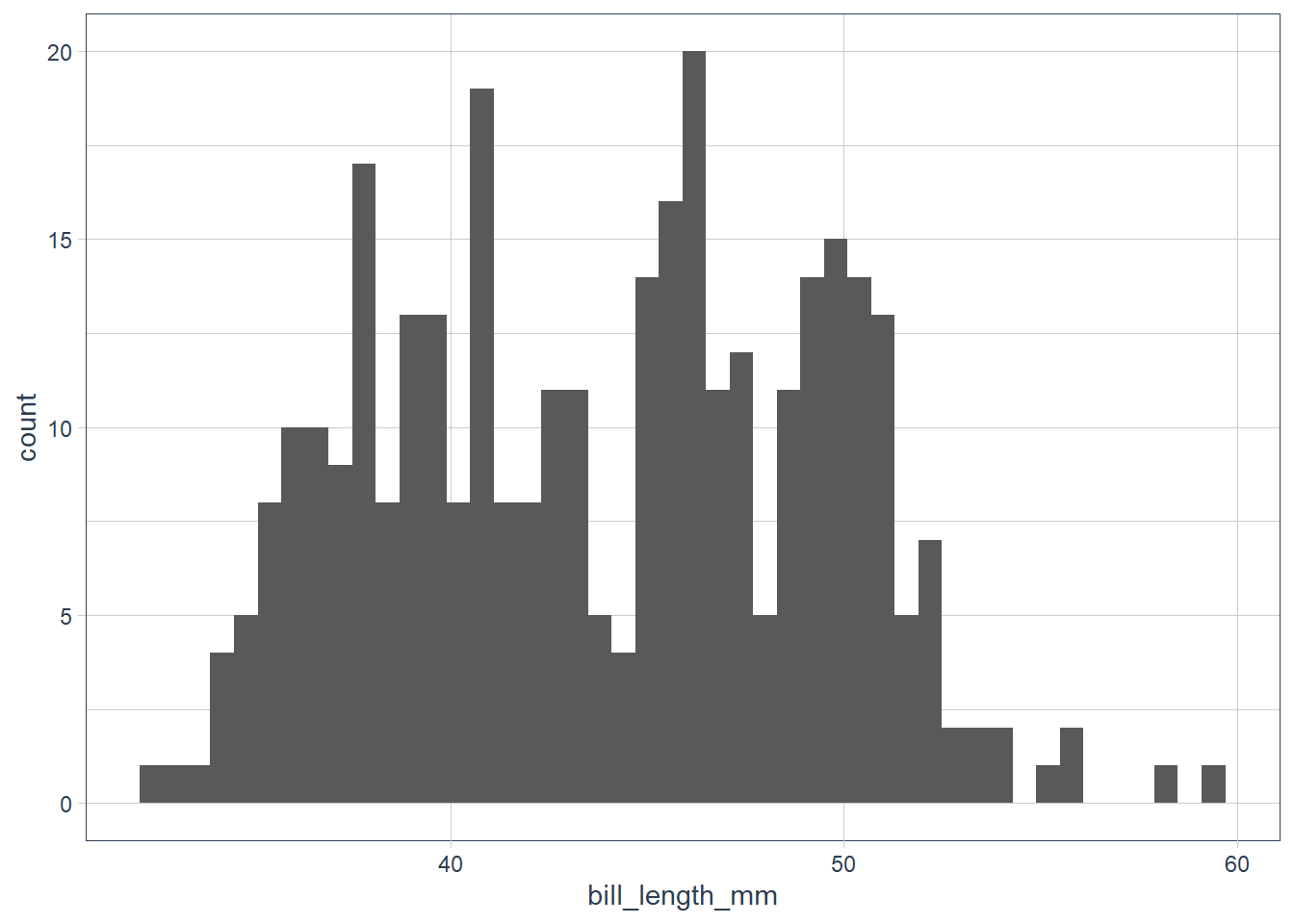penguins %>%   count(cut_width(bill_length_mm, 0.6))

# A tibble: 42 x 2
cut_width(bill_length_mm, 0.6)     n
<fct>                            <int>
1 [32.1,32.7]                          1
2 (32.7,33.3]                          1
3 (33.3,33.9]                          1
4 (33.9,34.5]                          3
5 (34.5,35.1]                          5
6 (35.1,35.7]                          6
7 (35.7,36.3]                         13
8 (36.3,36.9]                          9
9 (36.9,37.5]                          8
10 (37.5,38.1]                         15
# ... with 32 more rows


The data looks bimodal - i.e. there are 2 bumps in the distribution. If we recall we have 3 species, so let’s fill in the bars based on the species.

ggplot(data = penguins) +  geom_histogram(aes(x = bill_length_mm, fill = species),                 alpha = 0.5,                 binwidth = 0.6) +  scale_fill_tq()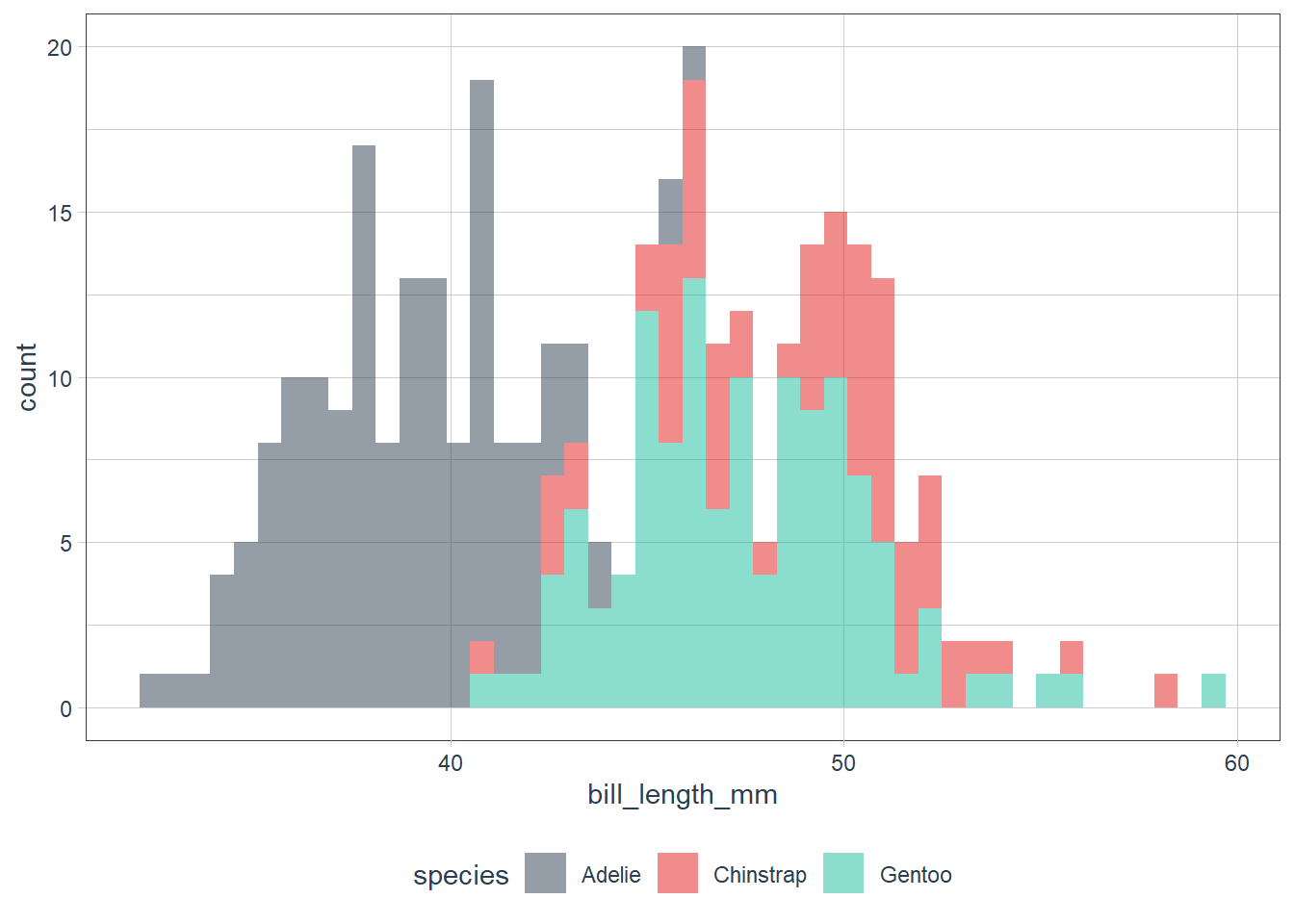penguins %>%   group_by(species) %>%   count(cut_width(bill_length_mm, 0.6))

# A tibble: 71 x 3
# Groups:   species 
species cut_width(bill_length_mm, 0.6)     n
<fct>   <fct>                            <int>
# ... with 61 more rows


When we have overlapping histograms, the suggestion is to use geom_freqpoly() which uses lines instead of bars.

ggplot(data = penguins) +  geom_freqpoly(aes(x = bill_length_mm, colour = species),                binwidth = 0.6) +  scale_fill_tq()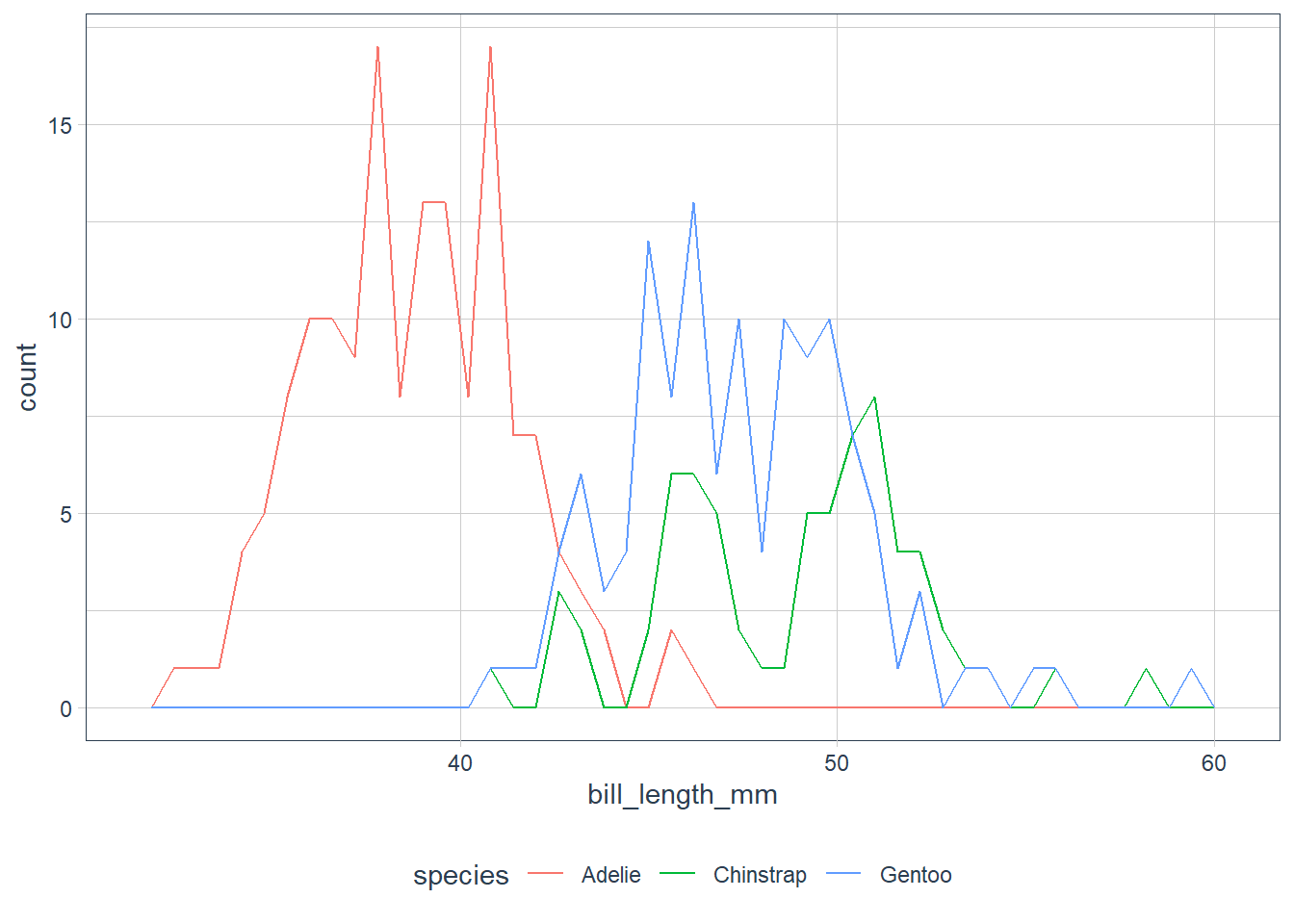• Which values are the most common? Why?

• Which values are rare? Why? Does that match your expectations?

• Can you see any unusual patterns? What might explain them?

Clusters of similar values suggest that subgroups exist in your data. To understand the subgroups, ask:

• How are the observations within each cluster similar to each other?

• How are the observations in separate clusters different from each other?

• How can you explain or describe the clusters?

• Why might the appearance of clusters be misleading?

### Investigate Unusual Values

Outliers are observations that don’t seem to gel nicely with the rest of the data.

Here the long y-axis indicates some outliers.

ggplot(data = diamonds) +
# y is a var in the dataset! it measures one of the
# dimensions of the diamond
geom_histogram(aes(x = y),
binwidth = 0.5)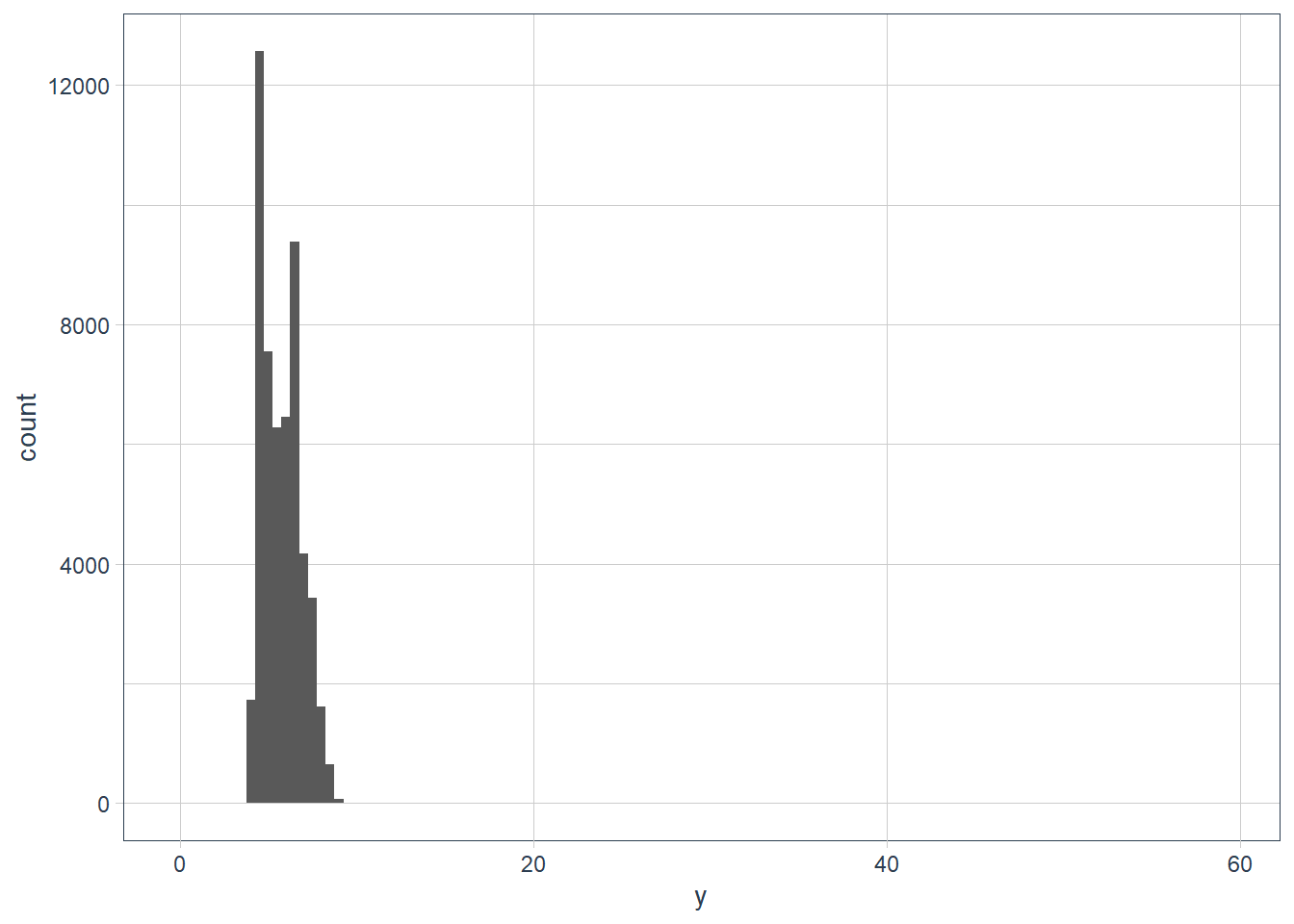To zoom in lets use coord_cartesian() with the ylim variable.

The coord_cartesian() also has an xlim to zoom into the x-axis.

ggplot(data = diamonds) +
geom_histogram(mapping = aes(x = y), binwidth = 0.5) +
# limit the y-axis to btw 0-50! Note to self: DON'T CONFUSE with the x-axis
# which is named y!!
coord_cartesian(ylim = c(0,50)) +
labs(x = "The variable y in the diamonds dataset")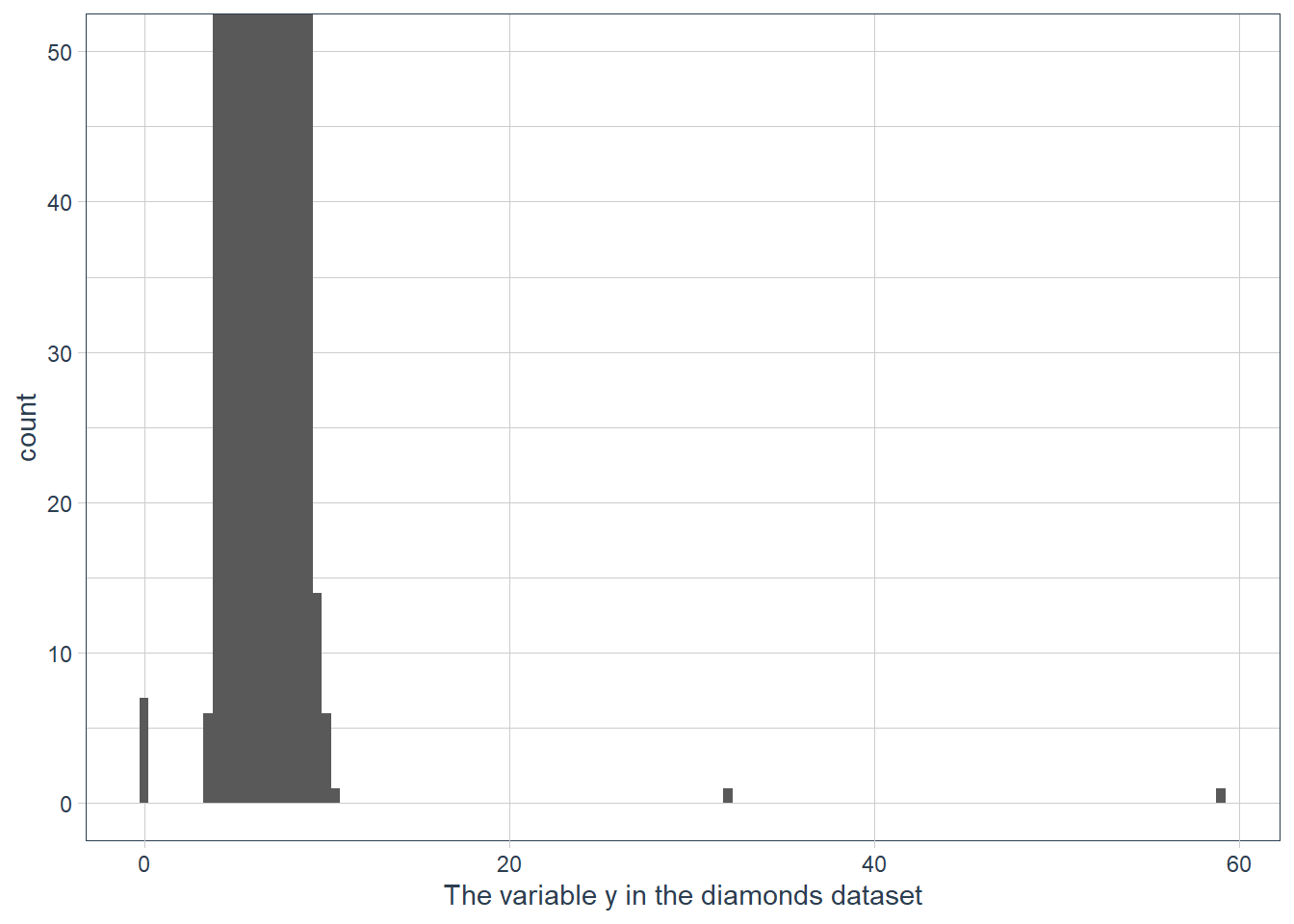# zoom into the unusual values
(unusual <- diamonds %>%
filter(y < 3 | y > 20) %>%
arrange(y)) %>%
print(width = Inf) # print the whole dataset so we can see all cols
# A tibble: 9 x 10
carat cut       color clarity depth table price     x     y     z
<dbl> <ord>     <ord> <ord>   <dbl> <dbl> <int> <dbl> <dbl> <dbl>
1  1    Very Good H     VS2      63.3    53  5139  0      0    0
2  1.14 Fair      G     VS1      57.5    67  6381  0      0    0
3  1.56 Ideal     G     VS2      62.2    54 12800  0      0    0
4  1.2  Premium   D     VVS1     62.1    59 15686  0      0    0
5  2.25 Premium   H     SI2      62.8    59 18034  0      0    0
6  0.71 Good      F     SI2      64.1    60  2130  0      0    0
7  0.71 Good      F     SI2      64.1    60  2130  0      0    0
8  0.51 Ideal     E     VS1      61.8    55  2075  5.15  31.8  5.12
9  2    Premium   H     SI2      58.9    57 12210  8.09  58.9  8.06

The y feature in the diamonds dataset measures a dimension (width, height, depth) in millimeters. A dimension of 0 mm is incorrect, and a dimension of ~32mm or ~59mm is also incorrect. Those diamonds will cost an 💪 and a 🦵!

In practice it is best to do analysis with and without your outliers.

• No difference with or without, probably safe to remove them
• Big difference with vs without, need to investigate why they are there before any action.
• In both cases, disclose the action taken in your analysis.

### Exercises

1. Explore the distribution of each of the x, y, and z variables in diamonds. What do you learn? Think about a diamond and how you might decide which dimension is the length, width, and depth.

ggplot(data = diamonds) +
geom_histogram(aes(x=x),
binwidth = 0.5)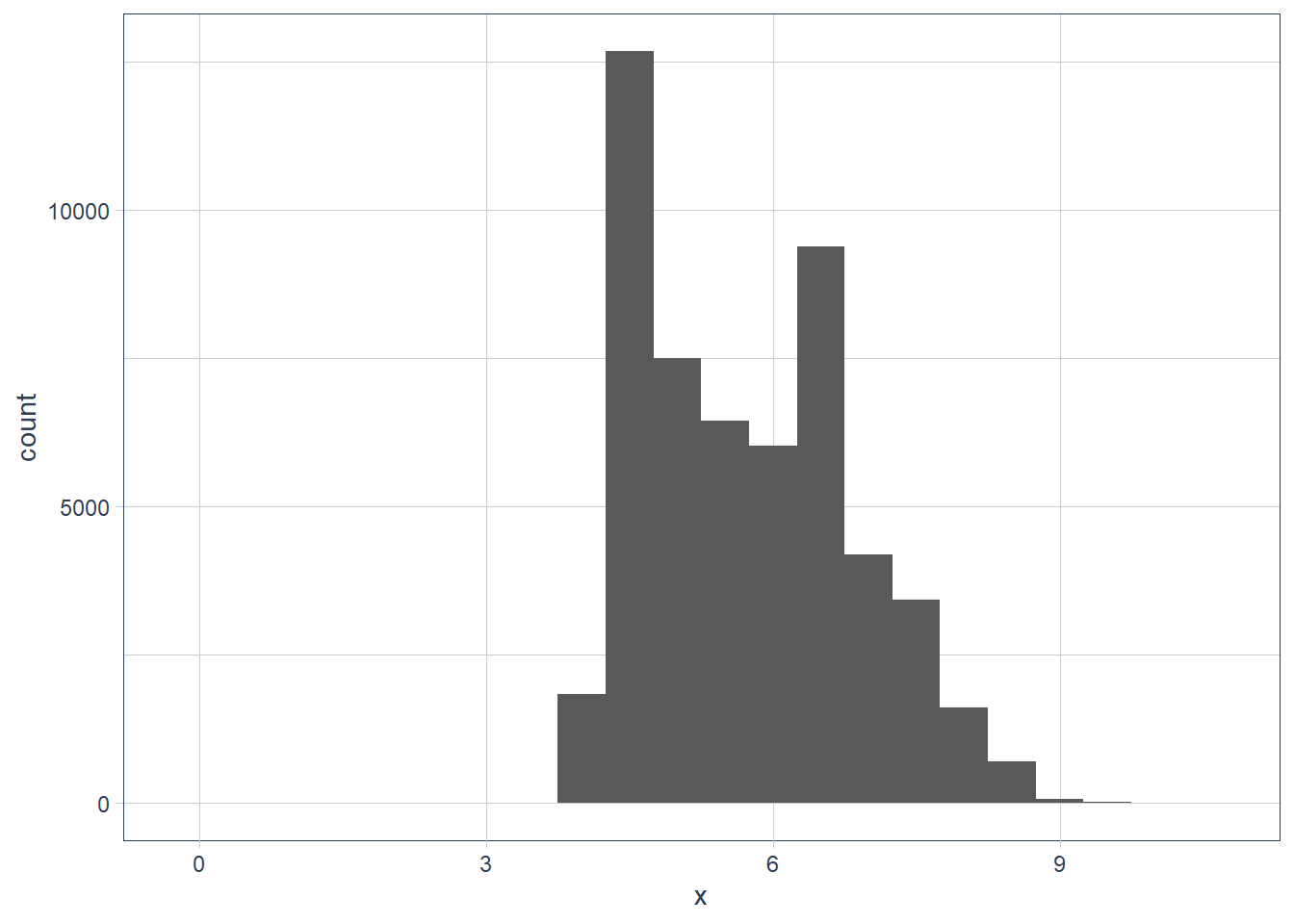filter(diamonds, x == max(x)) %>%
print(width = Inf)
# A tibble: 1 x 10
carat cut   color clarity depth table price     x     y     z
<dbl> <ord> <ord> <ord>   <dbl> <dbl> <int> <dbl> <dbl> <dbl>
1  5.01 Fair  J     I1       65.5    59 18018  10.7  10.5  6.98
filter(diamonds, x == min(x)) %>%
print(width = Inf)
# A tibble: 8 x 10
carat cut       color clarity depth table price     x     y     z
<dbl> <ord>     <ord> <ord>   <dbl> <dbl> <int> <dbl> <dbl> <dbl>
1  1.07 Ideal     F     SI2      61.6    56  4954     0  6.62     0
2  1    Very Good H     VS2      63.3    53  5139     0  0        0
3  1.14 Fair      G     VS1      57.5    67  6381     0  0        0
4  1.56 Ideal     G     VS2      62.2    54 12800     0  0        0
5  1.2  Premium   D     VVS1     62.1    59 15686     0  0        0
6  2.25 Premium   H     SI2      62.8    59 18034     0  0        0
7  0.71 Good      F     SI2      64.1    60  2130     0  0        0
8  0.71 Good      F     SI2      64.1    60  2130     0  0        0
diamonds %>%
filter(x != 0) %>%
filter(x == min(x)) %>%
print(width = Inf)
# A tibble: 2 x 10
carat cut     color clarity depth table price     x     y     z
<dbl> <ord>   <ord> <ord>   <dbl> <dbl> <int> <dbl> <dbl> <dbl>
1   0.2 Premium F     VS2      62.6    59   367  3.73  3.71  2.33
2   0.2 Premium D     VS2      62.3    60   367  3.73  3.68  2.31
ggplot(data = diamonds) +
geom_histogram(aes(x = x), binwidth = 0.5) +
coord_cartesian(xlim = c(0, 1),
ylim = c(0, 10))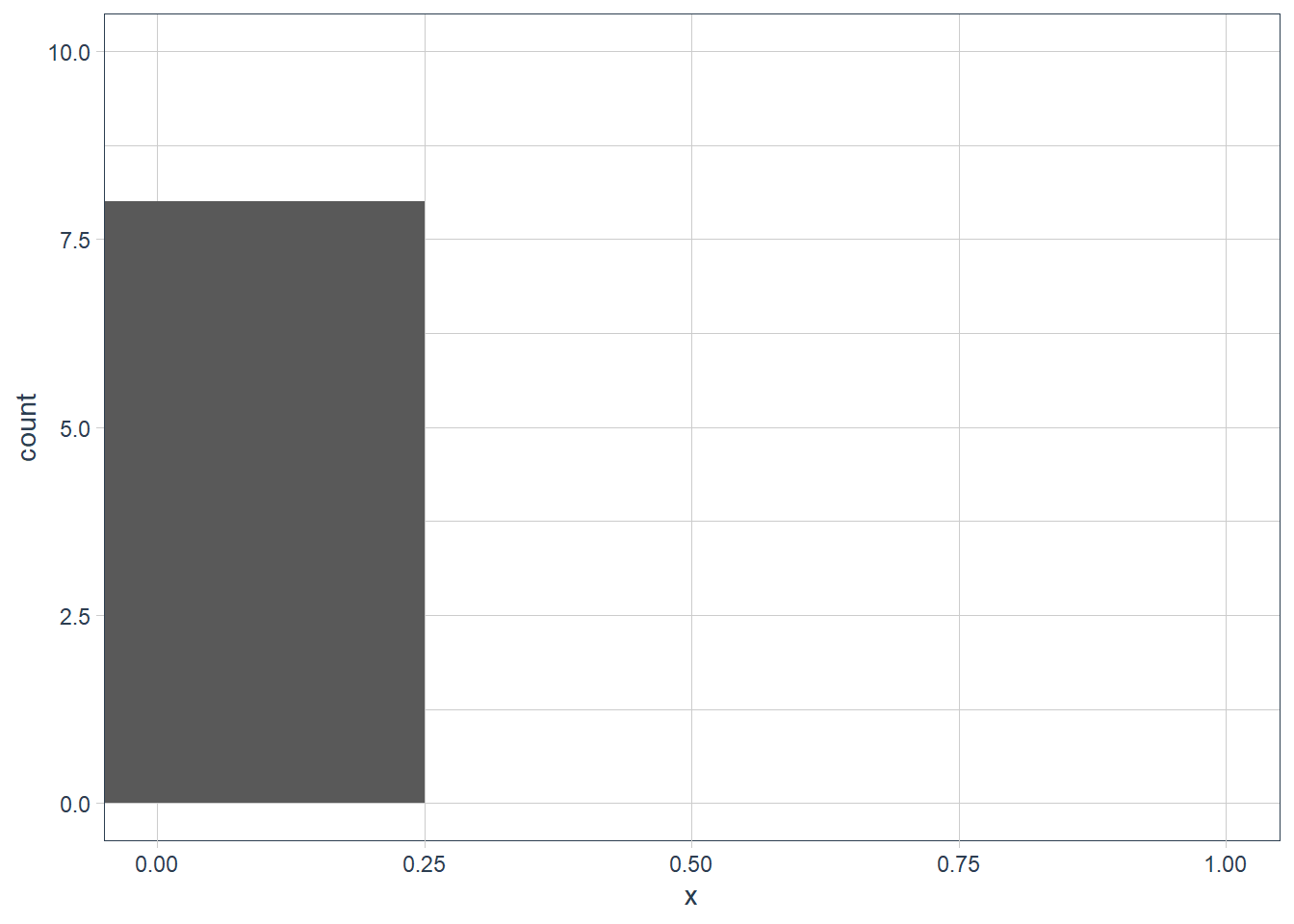ggplot(data = diamonds) +
geom_histogram(aes(x = y),
binwidth = 0.5)filter(diamonds, y == max(y)) %>%
print(width = Inf)
# A tibble: 1 x 10
carat cut     color clarity depth table price     x     y     z
<dbl> <ord>   <ord> <ord>   <dbl> <dbl> <int> <dbl> <dbl> <dbl>
1     2 Premium H     SI2      58.9    57 12210  8.09  58.9  8.06
filter(diamonds, y == min(y)) %>%
print(width = Inf)
# A tibble: 7 x 10
carat cut       color clarity depth table price     x     y     z
<dbl> <ord>     <ord> <ord>   <dbl> <dbl> <int> <dbl> <dbl> <dbl>
1  1    Very Good H     VS2      63.3    53  5139     0     0     0
2  1.14 Fair      G     VS1      57.5    67  6381     0     0     0
3  1.56 Ideal     G     VS2      62.2    54 12800     0     0     0
4  1.2  Premium   D     VVS1     62.1    59 15686     0     0     0
5  2.25 Premium   H     SI2      62.8    59 18034     0     0     0
6  0.71 Good      F     SI2      64.1    60  2130     0     0     0
7  0.71 Good      F     SI2      64.1    60  2130     0     0     0
diamonds %>%
filter(y != 0) %>%
filter(y == min(y)) %>%
print(width = Inf)    
# A tibble: 1 x 10
carat cut     color clarity depth table price     x     y     z
<dbl> <ord>   <ord> <ord>   <dbl> <dbl> <int> <dbl> <dbl> <dbl>
1   0.2 Premium D     VS2      62.3    60   367  3.73  3.68  2.31
diamonds %>%
filter(y < 10) %>%
filter(y == max(y)) %>%
print(width = Inf)    
# A tibble: 2 x 10
carat cut       color clarity depth table price     x     y     z
<dbl> <ord>     <ord> <ord>   <dbl> <dbl> <int> <dbl> <dbl> <dbl>
1  4.01 Premium   J     I1       62.5    62 15223  10.0  9.94  6.24
2  4    Very Good I     I1       63.3    58 15984  10.0  9.94  6.31
ggplot(data = diamonds) +
geom_histogram(aes(x = z),
binwidth = 0.5)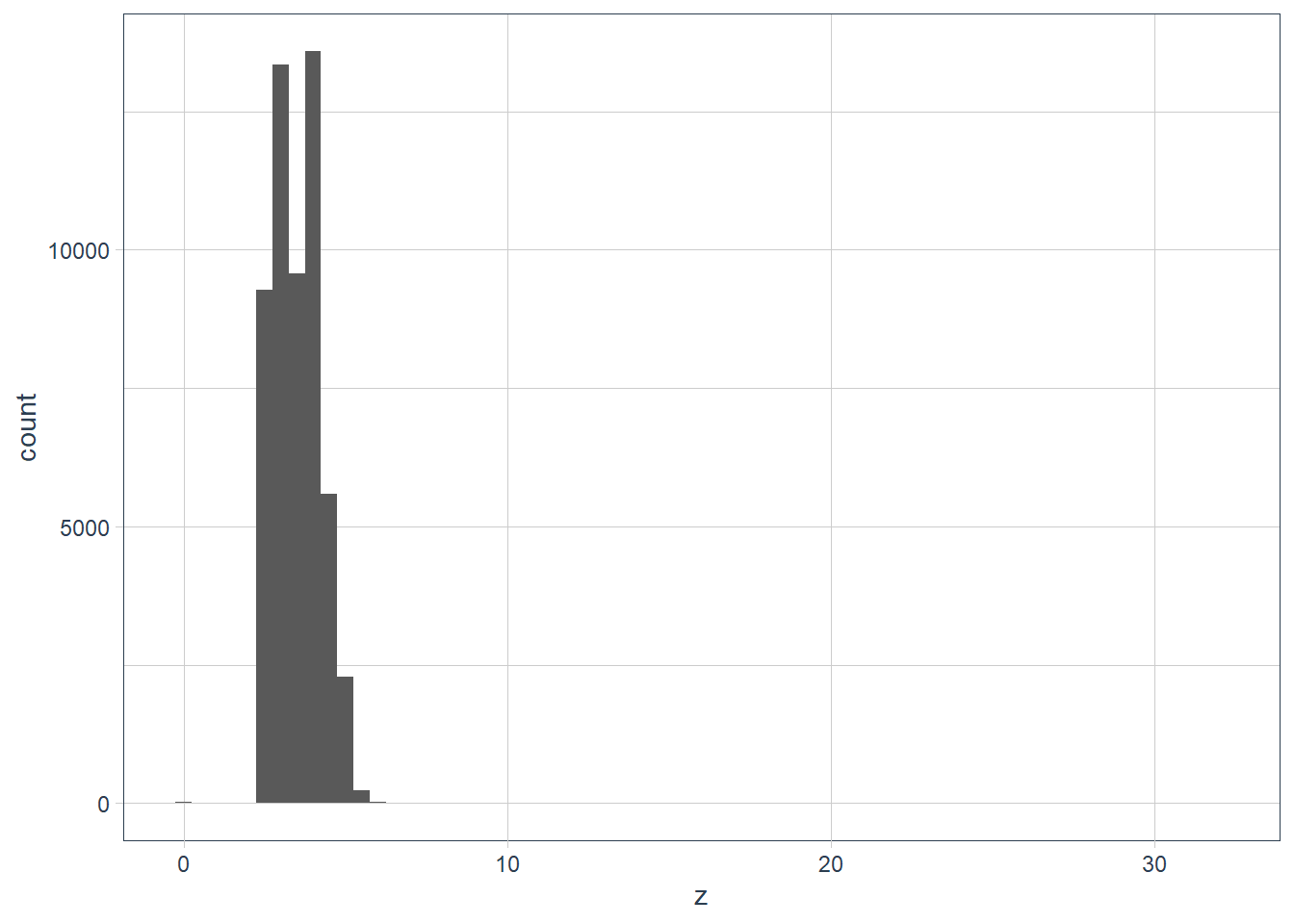filter(diamonds, z == max(z)) %>%
print(width = Inf)
# A tibble: 1 x 10
carat cut       color clarity depth table price     x     y     z
<dbl> <ord>     <ord> <ord>   <dbl> <dbl> <int> <dbl> <dbl> <dbl>
1  0.51 Very Good E     VS1      61.8  54.7  1970  5.12  5.15  31.8
filter(diamonds, z == min(z)) %>%
print(width = Inf)
# A tibble: 20 x 10
carat cut       color clarity depth table price     x     y     z
<dbl> <ord>     <ord> <ord>   <dbl> <dbl> <int> <dbl> <dbl> <dbl>
1  1    Premium   G     SI2      59.1    59  3142  6.55  6.48     0
2  1.01 Premium   H     I1       58.1    59  3167  6.66  6.6      0
3  1.1  Premium   G     SI2      63      59  3696  6.5   6.47     0
4  1.01 Premium   F     SI2      59.2    58  3837  6.5   6.47     0
5  1.5  Good      G     I1       64      61  4731  7.15  7.04     0
6  1.07 Ideal     F     SI2      61.6    56  4954  0     6.62     0
7  1    Very Good H     VS2      63.3    53  5139  0     0        0
8  1.15 Ideal     G     VS2      59.2    56  5564  6.88  6.83     0
9  1.14 Fair      G     VS1      57.5    67  6381  0     0        0
10  2.18 Premium   H     SI2      59.4    61 12631  8.49  8.45     0
11  1.56 Ideal     G     VS2      62.2    54 12800  0     0        0
12  2.25 Premium   I     SI1      61.3    58 15397  8.52  8.42     0
13  1.2  Premium   D     VVS1     62.1    59 15686  0     0        0
14  2.2  Premium   H     SI1      61.2    59 17265  8.42  8.37     0
15  2.25 Premium   H     SI2      62.8    59 18034  0     0        0
16  2.02 Premium   H     VS2      62.7    53 18207  8.02  7.95     0
17  2.8  Good      G     SI2      63.8    58 18788  8.9   8.85     0
18  0.71 Good      F     SI2      64.1    60  2130  0     0        0
19  0.71 Good      F     SI2      64.1    60  2130  0     0        0
20  1.12 Premium   G     I1       60.4    59  2383  6.71  6.67     0
unusual_z <- diamonds %>%
filter(z < 2 | z > 10)

ggplot(data = unusual_z) +
geom_histogram(aes(x = z), binwidth = 0.5) +
coord_cartesian(xlim = c(0, 35),
ylim = c(0, 25))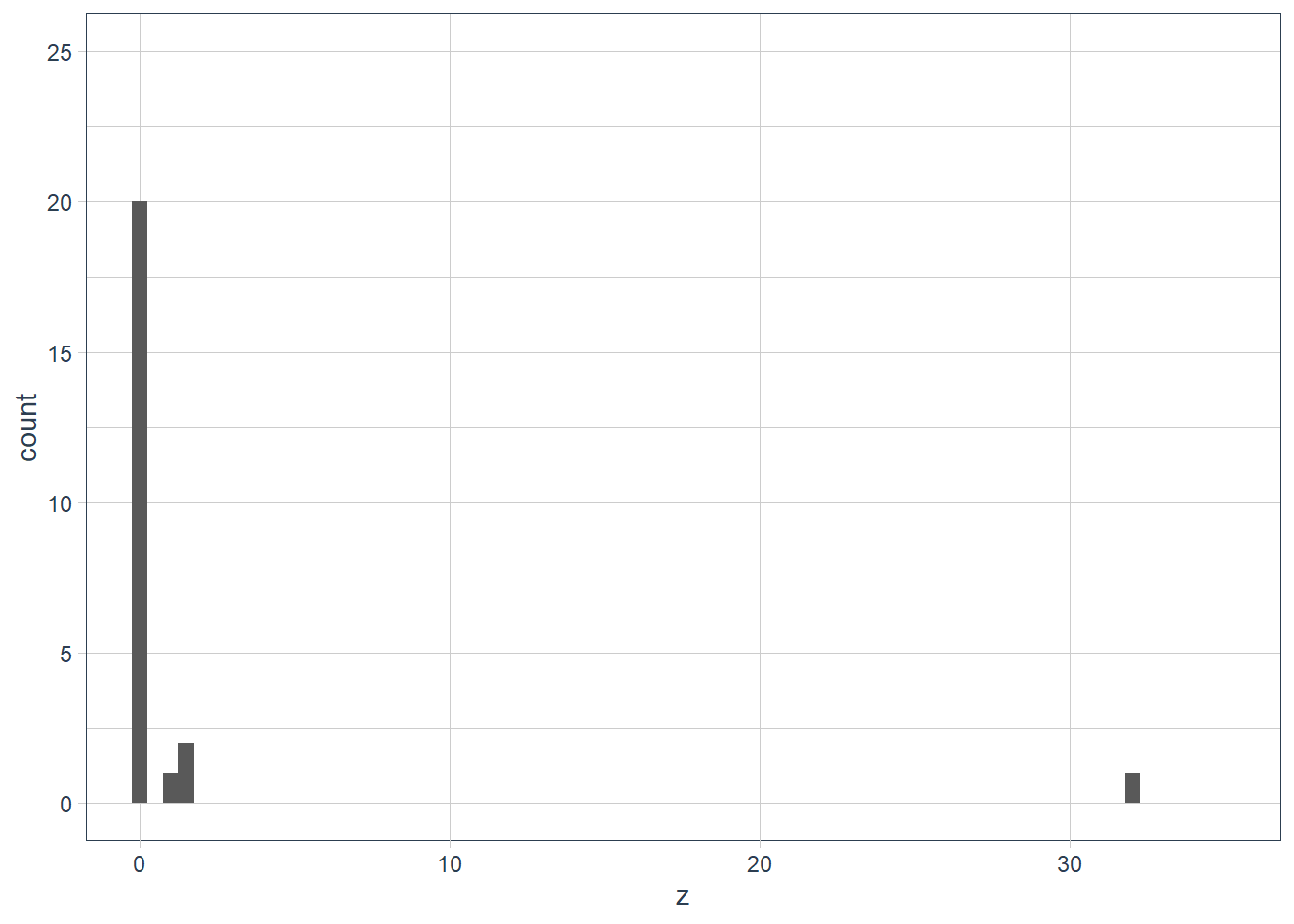diamonds %>%
select(x, y, z) %>%
filter(x != 0,
y != 0,
z != 0) %>%
skim()    
 Name Piped data Number of rows 53920 Number of columns 3 _______________________ Column type frequency: numeric 3 ________________________ Group variables None

Variable type: numeric

skim_variable n_missing complete_rate mean sd p0 p25 p50 p75 p100 hist
x 0 1 5.73 1.12 3.73 4.71 5.70 6.54 10.74 ▇▇▅▁▁
y 0 1 5.73 1.14 3.68 4.72 5.71 6.54 58.90 ▇▁▁▁▁
z 0 1 3.54 0.70 1.07 2.91 3.53 4.04 31.80 ▇▁▁▁▁

So it turns out a diamond has a table, width and depth.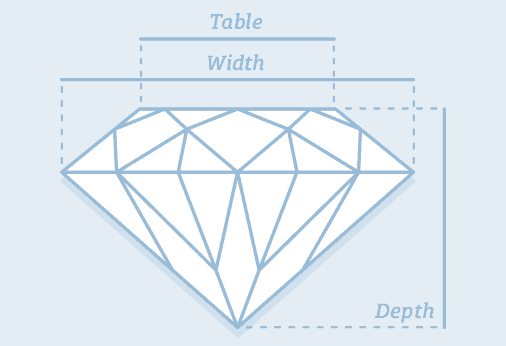Credit: https://www.diamonds.pro/

x and y could be depth or width - there’s not much difference between the 2 values. The z is likely to be the table since it is smaller.

But looking at the ?diamonds help page x = length, y = width and z = depth, and there is actually a table variable that is a relative measure 🤷, so there goes my “theory” 🤦.

2. Explore the distribution of price. Do you discover anything unusual or surprising? (Hint: Carefully think about the binwidth and make sure you try a wide range of values.)

ggplot(data = diamonds) +
geom_histogram(aes(x = price), bins = 20)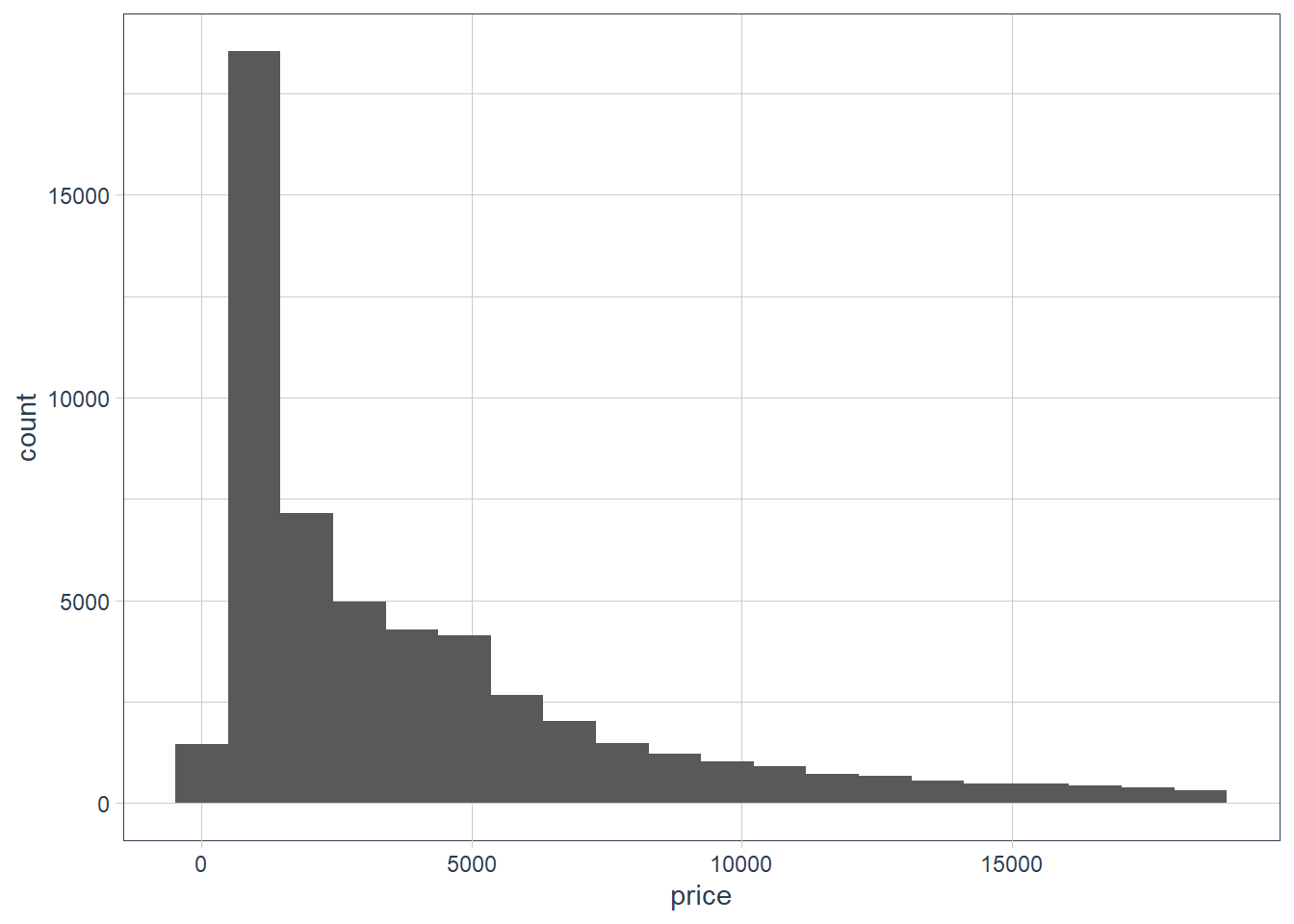ggplot(data = diamonds) +
geom_histogram(aes(x = price), binwidth = 1000)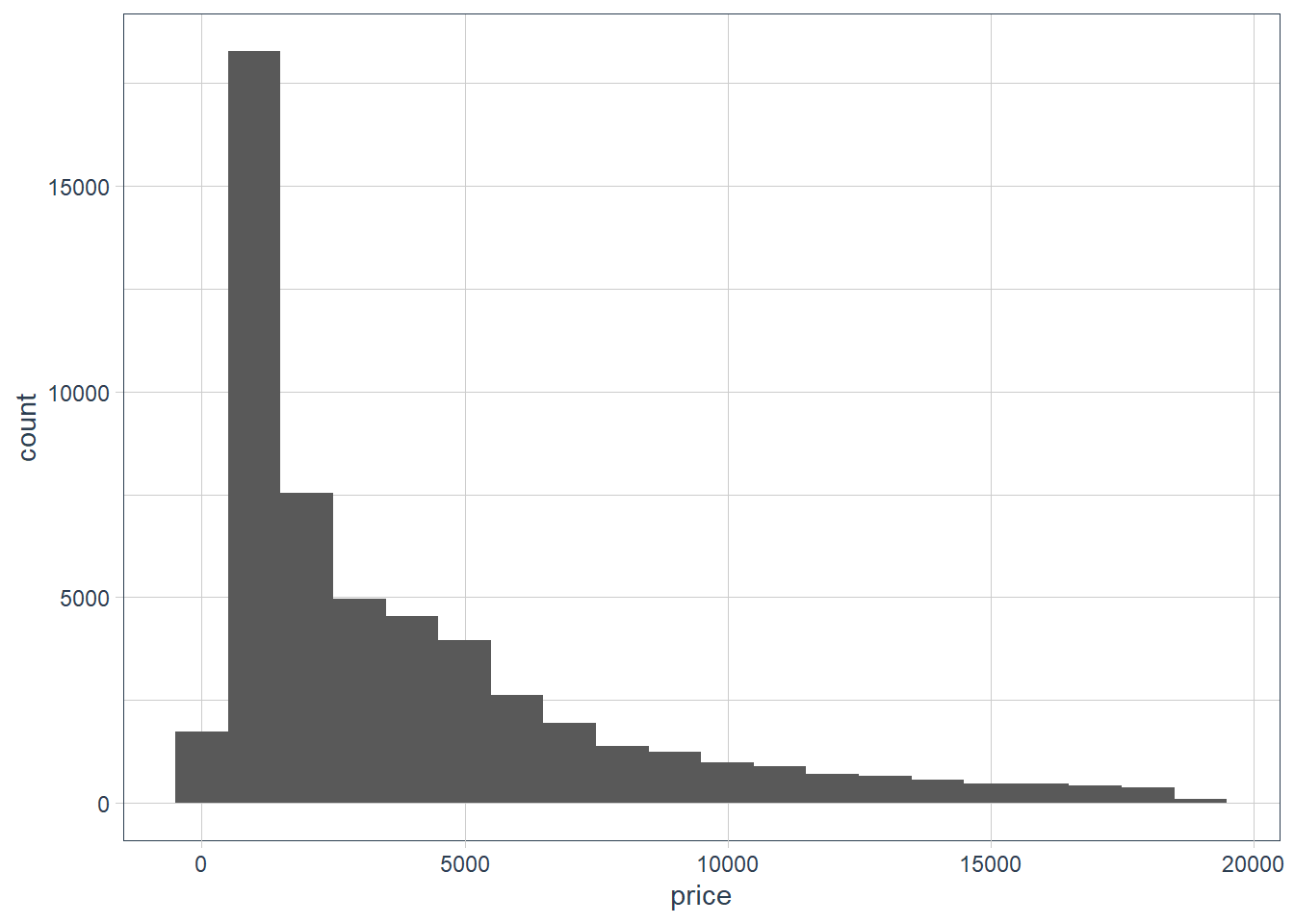ggplot(data = diamonds) +
geom_histogram(aes(x = price), binwidth = 200)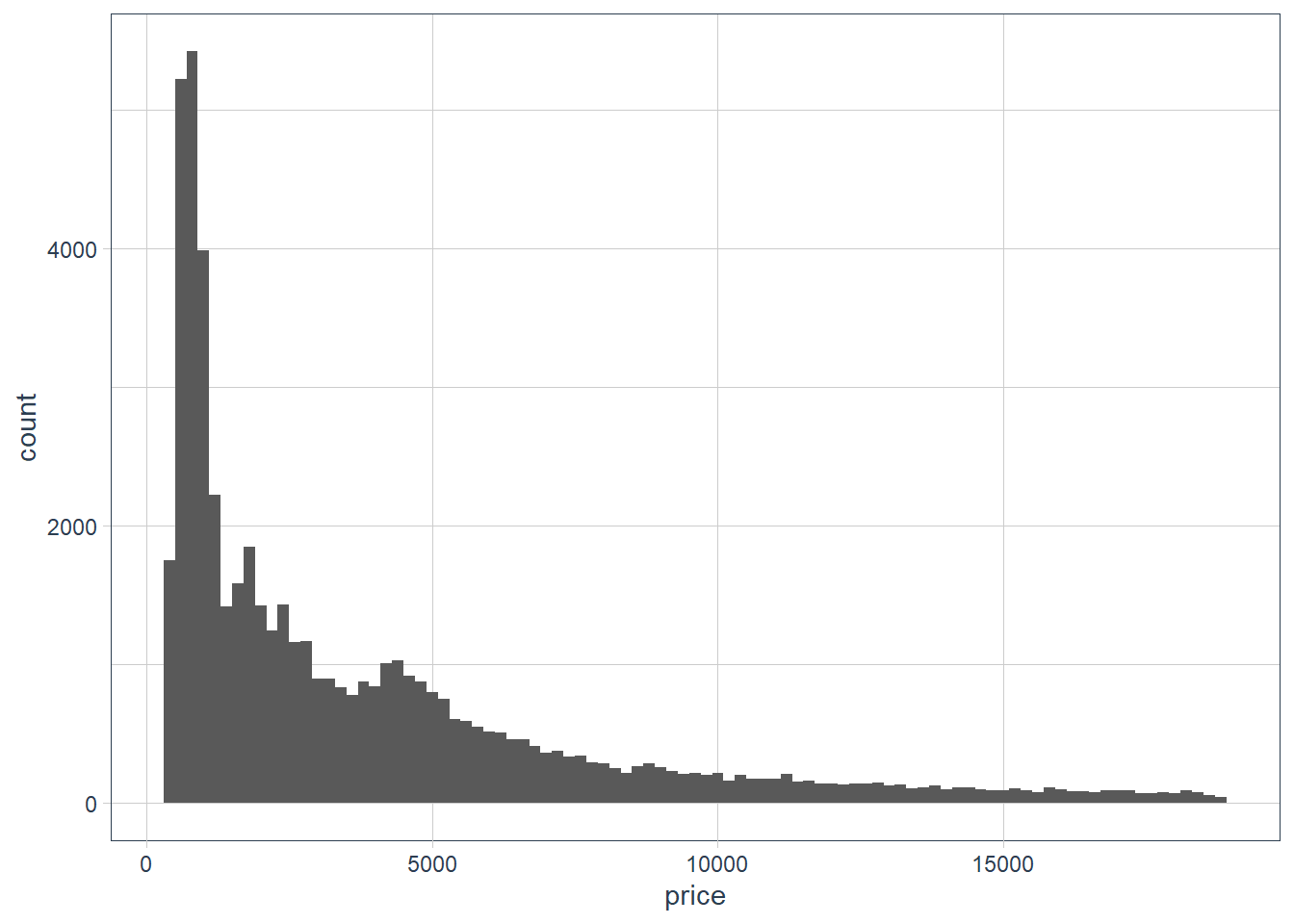ggplot(data = diamonds) +
geom_histogram(aes(x = price), binwidth = 500)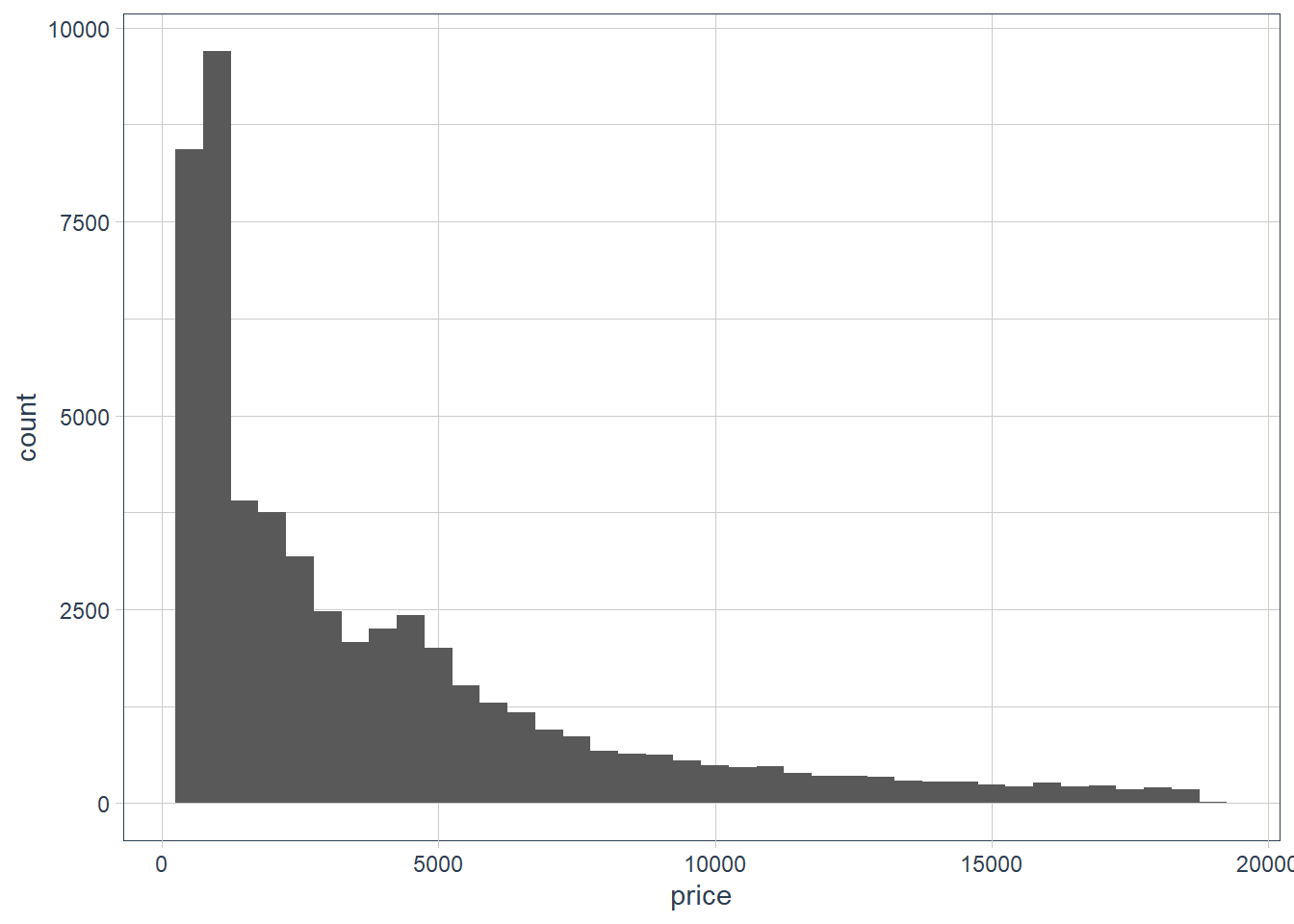diamonds %>%
select(price) %>%
skim()
 Name Piped data Number of rows 53940 Number of columns 1 _______________________ Column type frequency: numeric 1 ________________________ Group variables None

Variable type: numeric

skim_variable n_missing complete_rate mean sd p0 p25 p50 p75 p100 hist
price 0 1 3932.8 3989.44 326 950 2401 5324.25 18823 ▇▂▁▁▁
diamonds %>%
filter(price == 326 |
price == 18823) %>%
gt()
carat cut color clarity depth table price x y z
0.23 Ideal E SI2 61.5 55 326 3.95 3.98 2.43
0.21 Premium E SI1 59.8 61 326 3.89 3.84 2.31
2.29 Premium I VS2 60.8 60 18823 8.50 8.47 5.16
diamonds %>%
arrange(-carat) %>%
gt()
carat cut color clarity depth table price x y z
5.01 Fair J I1 65.5 59 18018 10.74 10.54 6.98
4.50 Fair J I1 65.8 58 18531 10.23 10.16 6.72
4.13 Fair H I1 64.8 61 17329 10.00 9.85 6.43
4.01 Premium I I1 61.0 61 15223 10.14 10.10 6.17
4.01 Premium J I1 62.5 62 15223 10.02 9.94 6.24
4.00 Very Good I I1 63.3 58 15984 10.01 9.94 6.31
3.67 Premium I I1 62.4 56 16193 9.86 9.81 6.13
3.65 Fair H I1 67.1 53 11668 9.53 9.48 6.38
3.51 Premium J VS2 62.5 59 18701 9.66 9.63 6.03
3.50 Ideal H I1 62.8 57 12587 9.65 9.59 6.03

The prices range from $326 to$18823. The carat of the high priced $18823 diamond is 2.29. There are other diamonds where the carat is bigger, and the cut is also Premium yet the price is lower. So it seems diamond pricing considers many factors, and could also be determined on who is doing the selling. 3. How many diamonds are 0.99 carat? How many are 1 carat? What do you think is the cause of the difference? diamonds %>% filter( carat == 1 | carat == 0.99 ) %>% count(carat, sort = TRUE) # A tibble: 2 x 2 carat n <dbl> <int> 1 1 1558 2 0.99 23 sample_test <- diamonds %>% filter(carat == 1 | carat == 0.99) %>% group_by(carat) %>% arrange(carat, price) sample_test %>% sample_n(20) %>% gt() cut color clarity depth table price x y z 0.99 Good F SI2 63.3 54 4052 6.36 6.43 4.05 Premium F VS2 62.6 55 5893 6.50 6.35 4.02 Very Good F SI2 61.2 56 4852 6.40 6.45 3.93 Fair I SI1 60.7 66 3337 6.42 6.34 3.87 Very Good D SI1 62.6 57 5671 6.34 6.37 3.98 Ideal F SI1 62.8 57 5728 6.30 6.38 3.98 Ideal H SI1 61.1 56 5287 6.44 6.47 3.94 Ideal F VS1 61.0 55 5947 6.46 6.52 3.96 Very Good F SI1 62.5 58 5112 6.36 6.38 3.98 Very Good G SI1 62.8 56 4863 6.34 6.36 3.99 Ideal F SI1 62.1 58 6038 6.33 6.40 3.95 Fair I SI2 68.1 56 2811 6.21 6.06 4.18 Fair H VS2 71.6 57 3593 5.94 5.80 4.20 Very Good J SI1 60.3 57 4002 6.44 6.49 3.90 Very Good F VS2 61.8 57 6094 6.37 6.39 3.94 Ideal I SI1 61.8 57 4763 6.40 6.42 3.96 Very Good E SI2 61.8 59 4780 6.30 6.33 3.90 Premium F SI2 60.6 61 4075 6.45 6.38 3.89 Very Good D SI2 62.5 57 4993 6.30 6.34 3.95 Fair J I1 73.6 60 1789 6.01 5.80 4.35 1 Very Good H SI1 62.1 58 5247 6.44 6.45 4.00 Very Good D SI1 60.1 60 4697 6.47 6.55 3.91 Premium H SI1 62.7 60 4469 6.30 6.24 3.93 Ideal E SI1 61.9 56 5458 6.37 6.45 3.97 Premium E VS2 63.0 58 7168 6.25 6.22 3.93 Good H VS1 61.8 61 5219 6.40 6.31 3.93 Very Good D IF 63.3 59 16073 6.37 6.33 4.02 Good D SI2 64.0 54 3304 6.29 6.24 4.01 Premium H VS2 61.5 61 5000 6.45 6.40 3.95 Fair F SI1 64.8 59 5292 6.29 6.24 4.06 Ideal E SI2 62.0 57 4623 6.37 6.41 3.96 Premium D SI1 61.5 60 5488 6.38 6.34 3.91 Premium G VS1 60.6 59 6115 6.48 6.42 3.91 Very Good I SI1 58.5 61 4649 6.51 6.55 3.82 Premium D SI2 61.6 58 4626 6.45 6.37 3.95 Premium H VS2 62.6 59 5320 6.37 6.31 3.97 Premium H SI2 62.8 60 3080 6.46 6.34 4.02 Ideal D SI1 63.0 56 4592 6.33 6.28 3.97 Premium F SI1 59.7 60 4939 6.51 6.48 3.88 Ideal G VS2 58.9 60 6235 6.53 6.58 3.86 sample_test %>% select(cut, price) %>% skim()  Name Piped data Number of rows 1581 Number of columns 3 _______________________ Column type frequency: factor 1 numeric 1 ________________________ Group variables carat Variable type: factor skim_variable carat n_missing complete_rate ordered n_unique top_counts cut 0.99 0 1 TRUE 5 Ver: 8, Fai: 7, Ide: 5, Pre: 2 cut 1.00 0 1 TRUE 5 Pre: 462, Ver: 389, Goo: 333, Ide: 208 Variable type: numeric skim_variable carat n_missing complete_rate mean sd p0 p25 p50 p75 p100 hist price 0.99 0 1 4406.17 1318.99 1789 3465 4780 5479 6094 ▂▅▅▇▇ price 1.00 0 1 5241.59 1603.94 1681 4155 4864 6073 16469 ▇▇▁▁▁ The one carat diamonds are gigher in price and hugely so in the upper range. 4. Compare and contrast coord_cartesian() vs xlim() or ylim() when zooming in on a histogram. What happens if you leave binwidth unset? What happens if you try and zoom so only half a bar shows? ggplot(data = diamonds) + geom_histogram(mapping = aes(x = y), binwidth = 0.5) + # limit the y-axis to btw 0-50! # Note to self: DON'T CONFUSE with the x-axis # which is named y!! coord_cartesian(ylim = c(0,50)) + labs(x = "The variable y in the diamonds dataset")ggplot(data = diamonds) + geom_histogram(mapping = aes(x = y), binwidth = 0.5) + ylim(c(0,50)) + labs(x = "The variable y in the diamonds dataset")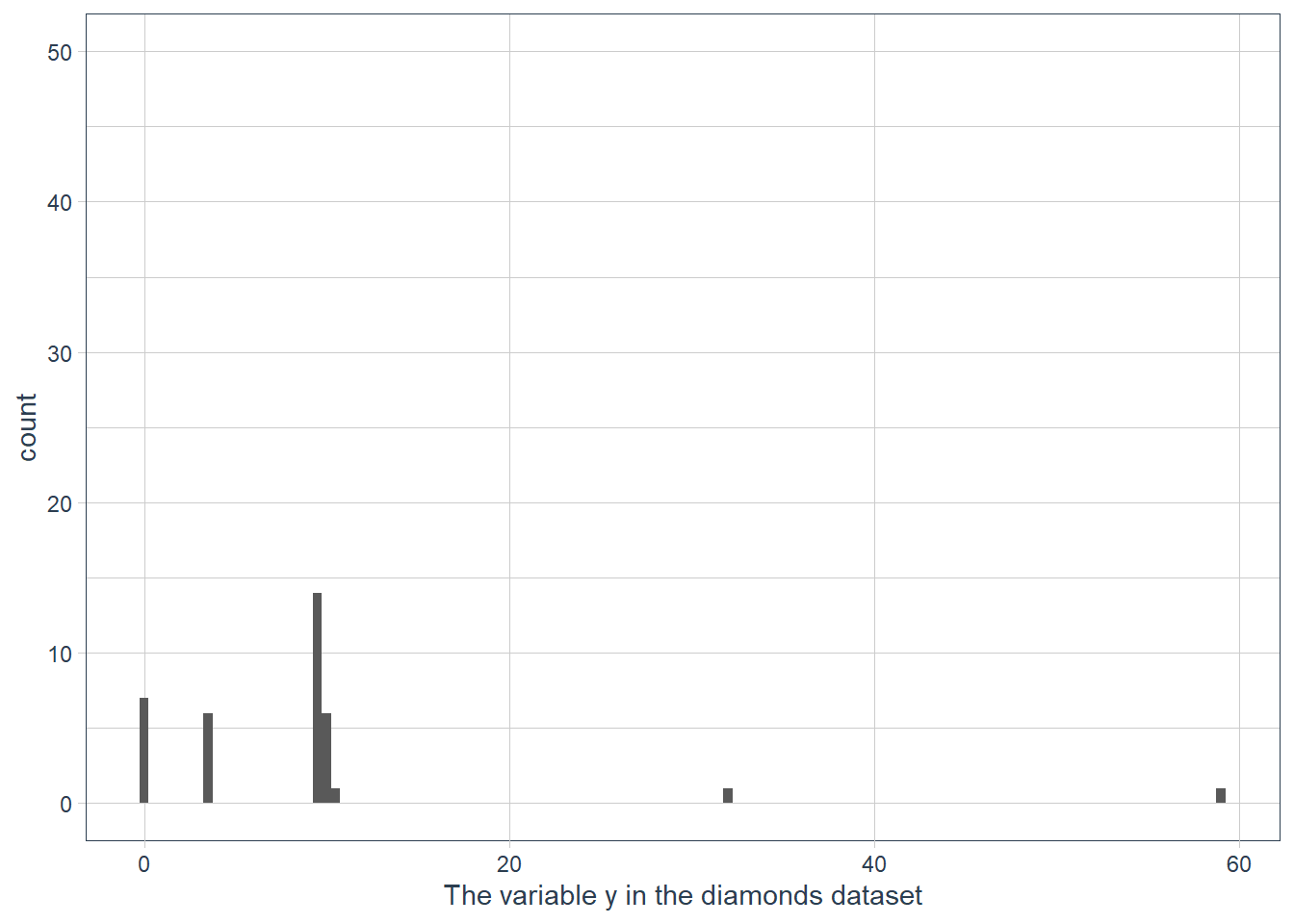ggplot(data = diamonds) + geom_histogram(mapping = aes(x = y)) + ylim(c(0,50)) + labs(x = "The variable y in the diamonds dataset")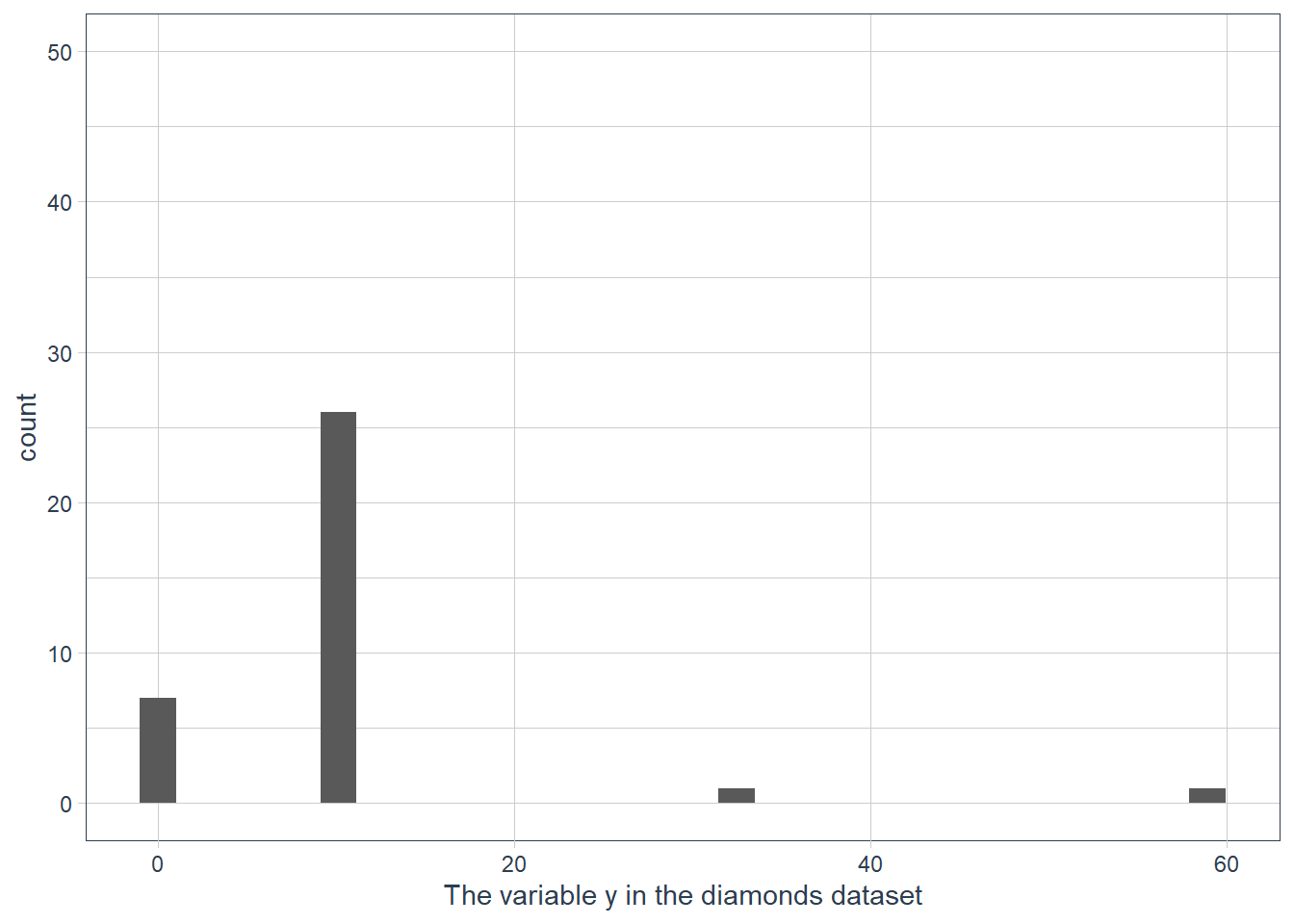Documentation reads: “This is a shortcut for supplying the limits argument to the individual scales. Note that, by default, any values outside the limits will be replaced with NA.” So ylim() and xlim() replace values outside the limit with NULL values resulting in the histogram being quite different to what we have when we use coord_cartesian(). If we don’t supply a binwidth ggplot defaults the number of bins to display to 30, and hence implicitly picks a binwidth for us. ## Leveraging Missing Values When you have weird values for variables, e.g. the ~59 mm for y, you may be tempted to drop these values and carry on. Often though a dataset is not nice and neat with all it’s weird values in a few observations! Usually the weird values are spread over multiple observations! diamonds %>% mutate(row_num = row_number()) %>% filter(x==0 | y==0 | z==0) %>% gt() carat cut color clarity depth table price x y z row_num 1.00 Premium G SI2 59.1 59 3142 6.55 6.48 0 2208 1.01 Premium H I1 58.1 59 3167 6.66 6.60 0 2315 1.10 Premium G SI2 63.0 59 3696 6.50 6.47 0 4792 1.01 Premium F SI2 59.2 58 3837 6.50 6.47 0 5472 1.50 Good G I1 64.0 61 4731 7.15 7.04 0 10168 1.07 Ideal F SI2 61.6 56 4954 0.00 6.62 0 11183 1.00 Very Good H VS2 63.3 53 5139 0.00 0.00 0 11964 1.15 Ideal G VS2 59.2 56 5564 6.88 6.83 0 13602 1.14 Fair G VS1 57.5 67 6381 0.00 0.00 0 15952 2.18 Premium H SI2 59.4 61 12631 8.49 8.45 0 24395 1.56 Ideal G VS2 62.2 54 12800 0.00 0.00 0 24521 2.25 Premium I SI1 61.3 58 15397 8.52 8.42 0 26124 1.20 Premium D VVS1 62.1 59 15686 0.00 0.00 0 26244 2.20 Premium H SI1 61.2 59 17265 8.42 8.37 0 27113 2.25 Premium H SI2 62.8 59 18034 0.00 0.00 0 27430 2.02 Premium H VS2 62.7 53 18207 8.02 7.95 0 27504 2.80 Good G SI2 63.8 58 18788 8.90 8.85 0 27740 0.71 Good F SI2 64.1 60 2130 0.00 0.00 0 49557 0.71 Good F SI2 64.1 60 2130 0.00 0.00 0 49558 1.12 Premium G I1 60.4 59 2383 6.71 6.67 0 51507 Throwing away all observations where a variable has weird values can result in a serious shrinking of your dataset. The authors suggest that instead of throwing away the observations with weird values, rather replace them with missing values. This may sound strange since many of us have probably heard that missing values shouldn’t be used in data science! It’s usually a problem to be fixed! But in EDA it may provide insight to either force weird values to be NA, or to investigate how missing values differ from non-missing values. ### Exercises 1. What happens to missing values in a histogram? What happens to missing values in a bar chart? Why is there a difference? Missing values in bar charts are plotted as a category. Missing values in a histogram are dropped. I guess that it is easy to create one category for missing and lump all the missing variable observations into that count. For a continuous variable where data is binned however how do you show that on a graph? The limits are continuous values e.g. from 0 - 100, -100 - 100 etc. Where should the count of NAs go on the x-axis, and y-axis given that the range that NA could represent is any number in the range of the continuous values we have for a variable. 2. What does na.rm = TRUE do in mean() and sum()? The NAs are removed and then the mean() or sum() is computed on the remaining non-NA values. c(1,2,NA,3,4,NA,5) %>% mean(na.rm=TRUE)  3 # (1+2+3+4+5) / 5 = 15 / 5 = 3 c(1,2,NA,3,4,NA,5) %>% sum(na.rm=TRUE)  15 # (1+2+3+4+5) ## Covariation Covariance is how two or more variables change together in some way. Visualisation is good for noticing these relationships. ### Categorical and Continuous #### Density Freq Polygons We previously used a geom_freqpoly() for this, which doesn’t provide much use if groups are unbalanced. If one group is much smaller than the others it’s hard to see differences. We can display density on the y-axis instead of a count. ..density.. is a count that is standardised so that the area under each curve is 1. ggplot(penguins, aes(x = body_mass_g)) + geom_freqpoly(aes(colour = species), binwidth = 200)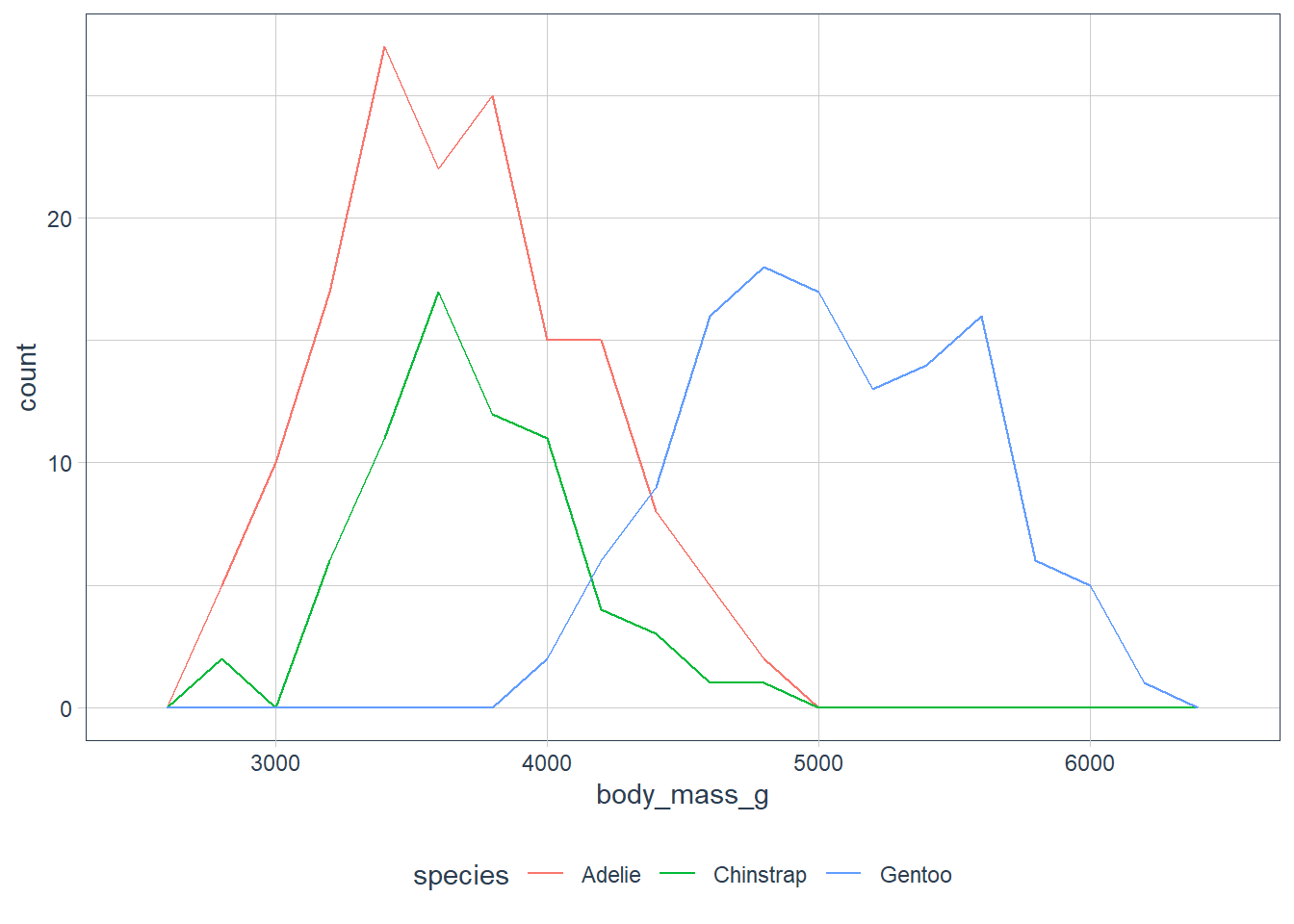ggplot(penguins, aes(x = body_mass_g, y = ..density..)) + geom_freqpoly(aes(colour = species), binwidth = 200)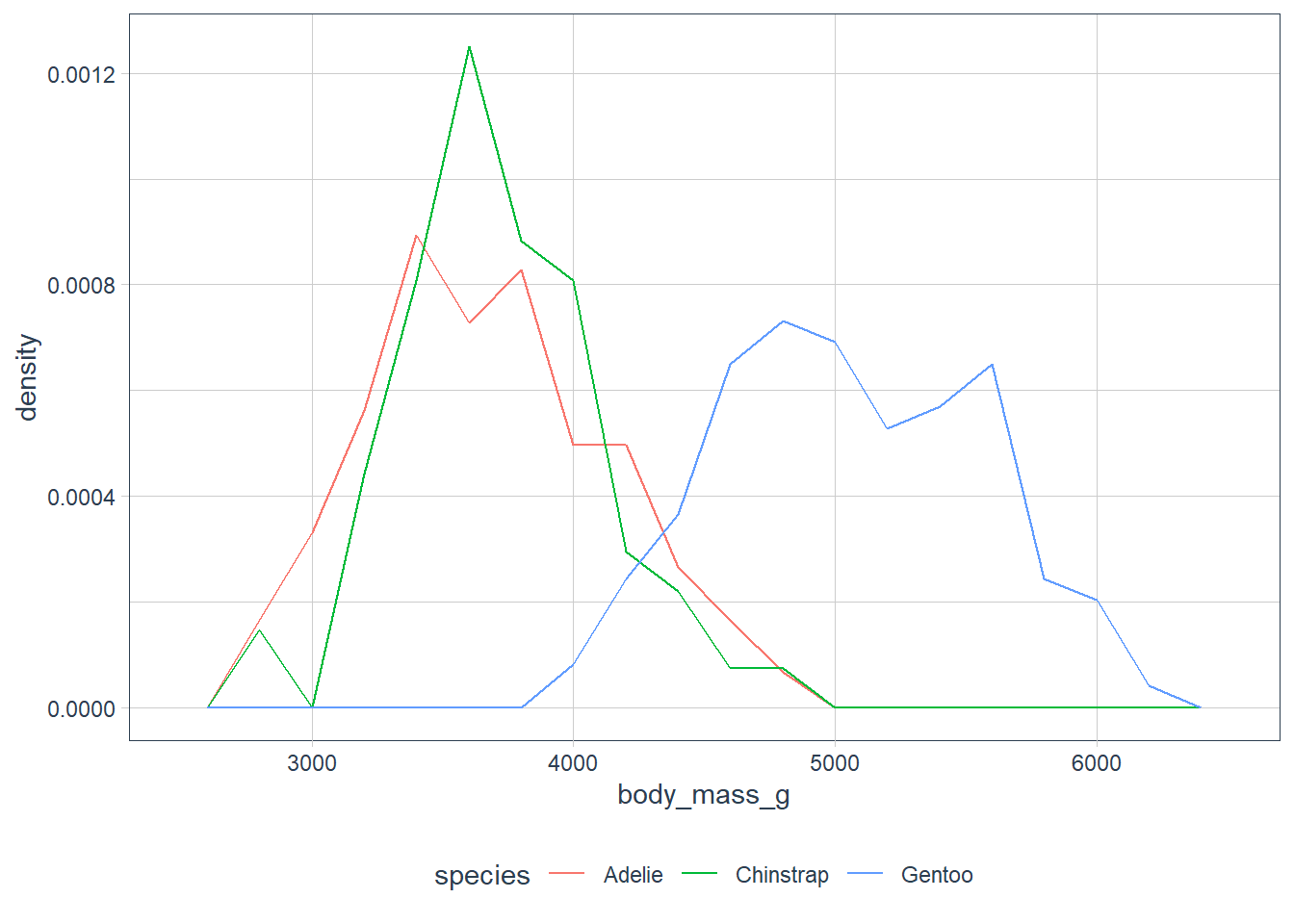ggplot(penguins, aes(x = bill_length_mm)) + geom_freqpoly(aes(colour = species), binwidth = 5)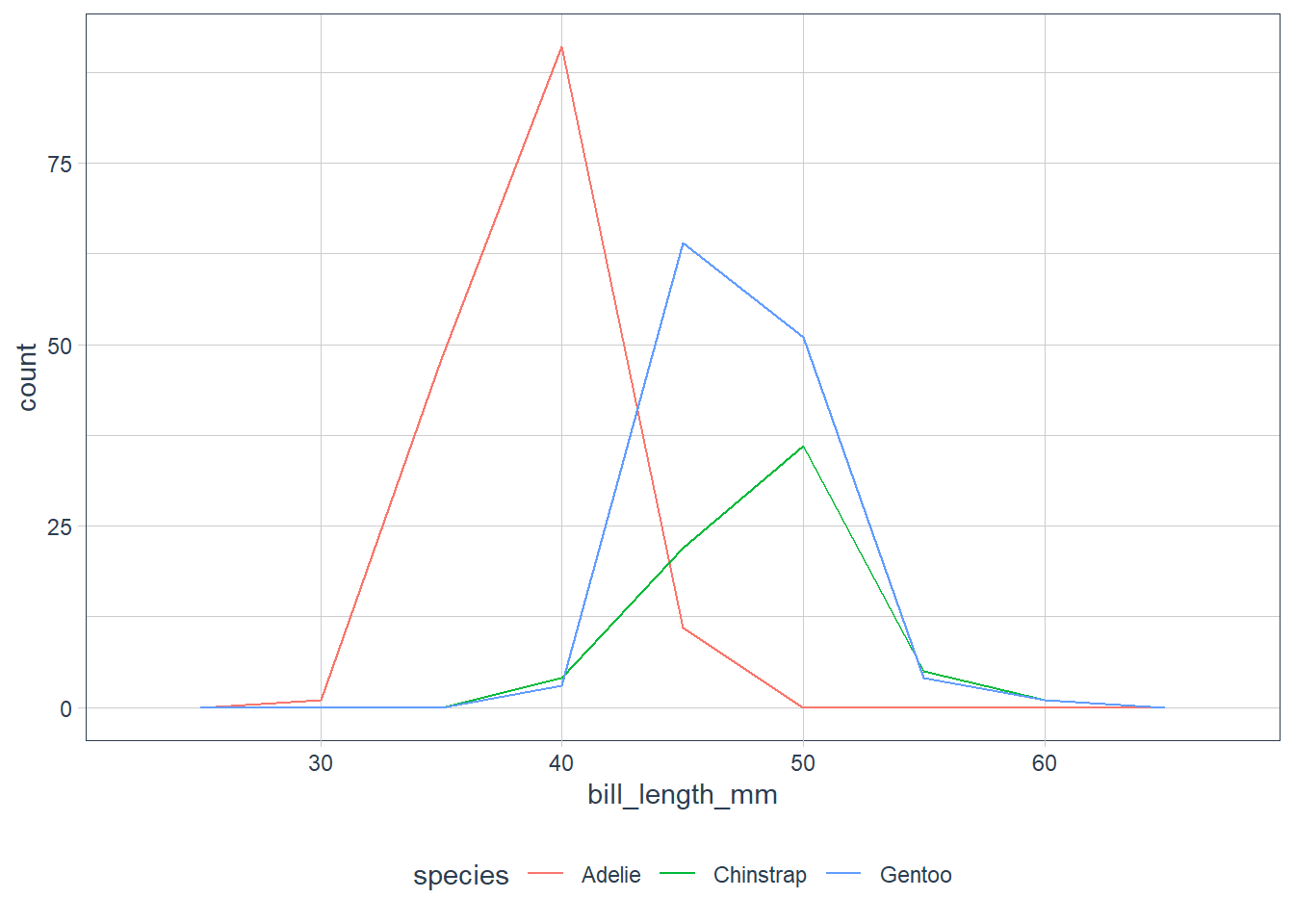ggplot(penguins, aes(x = bill_length_mm, y = ..density..)) + geom_freqpoly(aes(colour = species), binwidth = 5)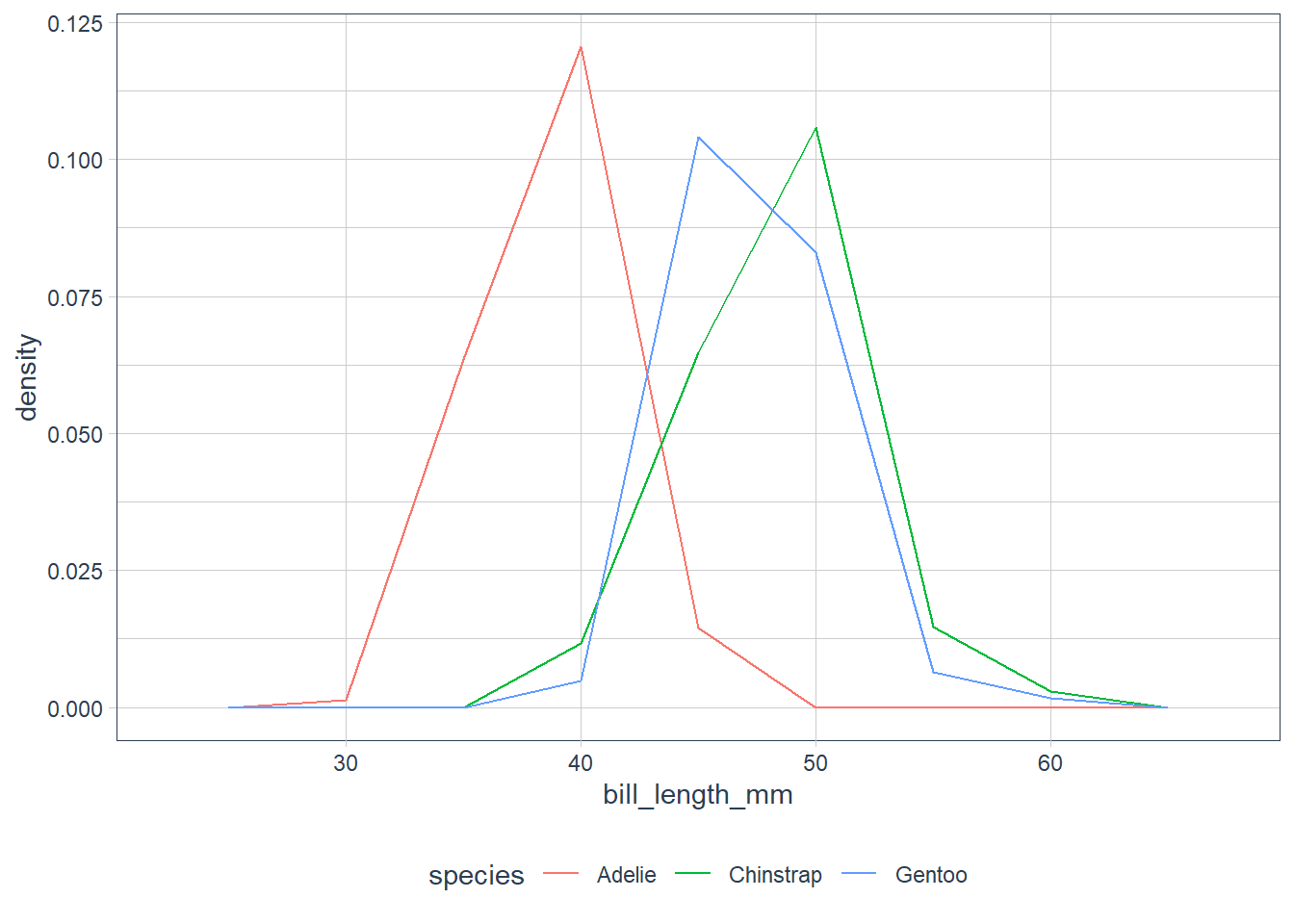ggplot(penguins, aes(x = bill_depth_mm)) + geom_freqpoly(aes(colour = species), binwidth = 5)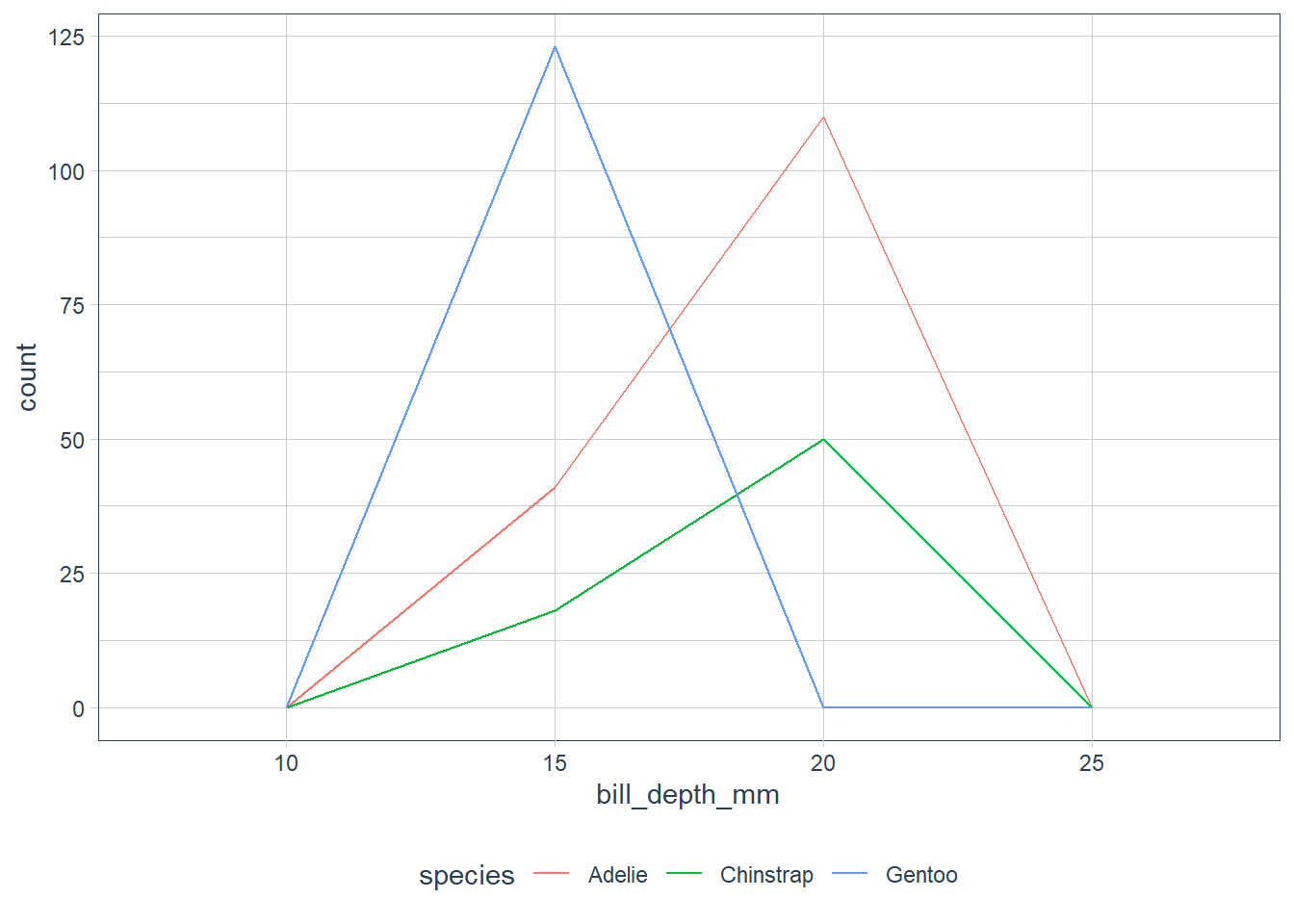ggplot(penguins, aes(x = bill_depth_mm, y = ..density..)) + geom_freqpoly(aes(colour = species), binwidth = 5)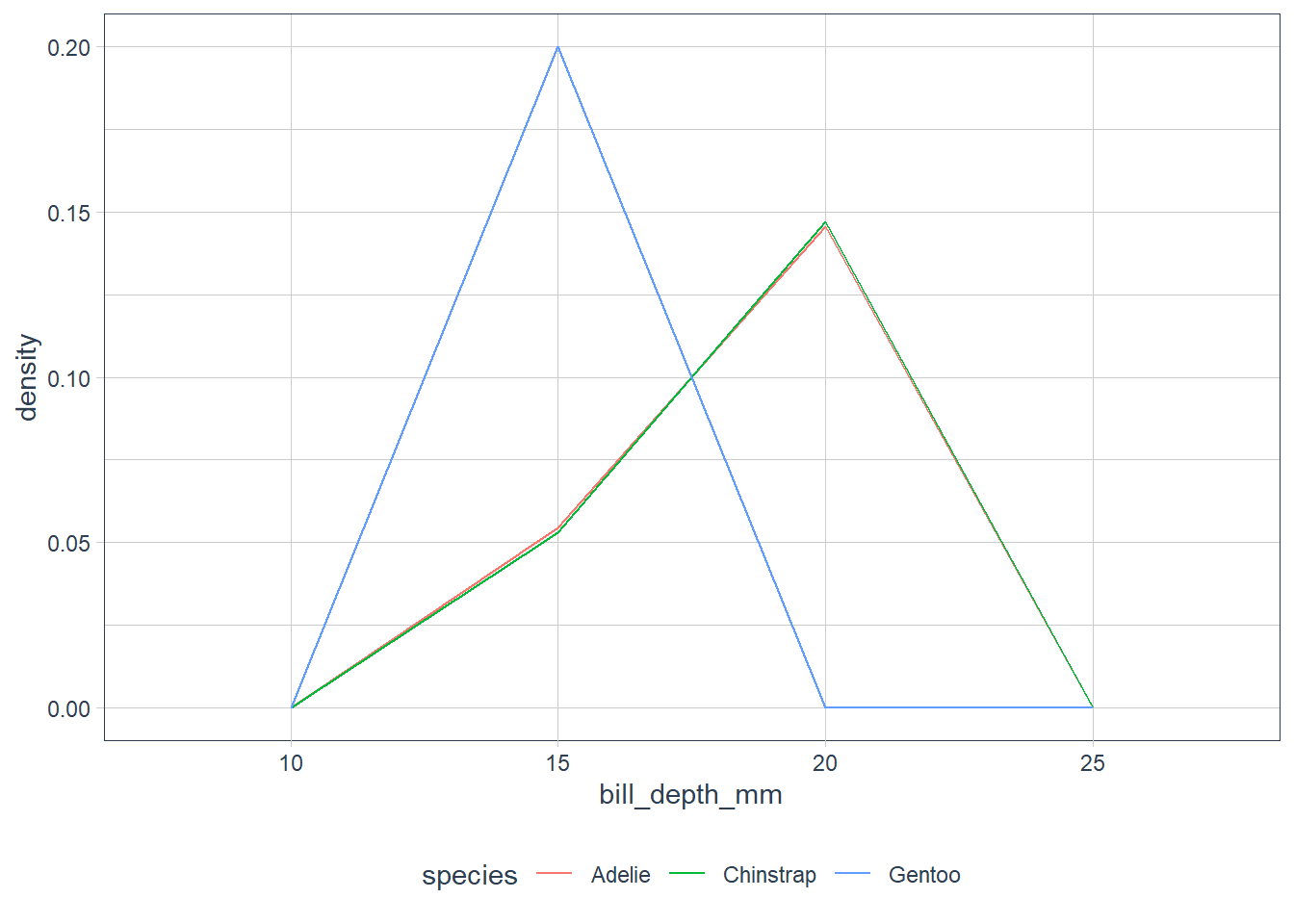ggplot(penguins, aes(x = flipper_length_mm)) + geom_freqpoly(aes(colour = species), binwidth = 5)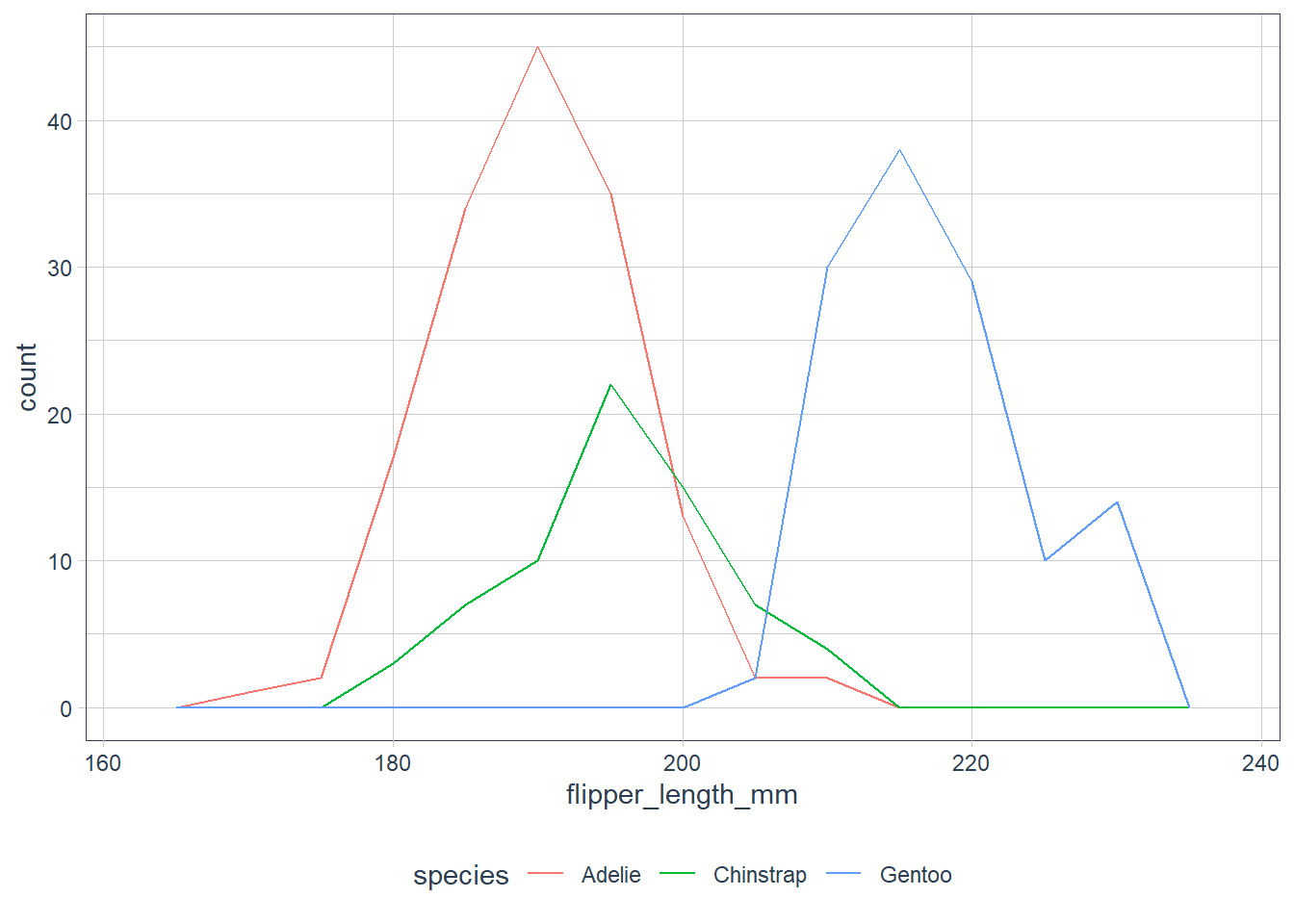ggplot(penguins, aes(x = flipper_length_mm, y = ..density..)) + geom_freqpoly(aes(colour = species), binwidth = 5)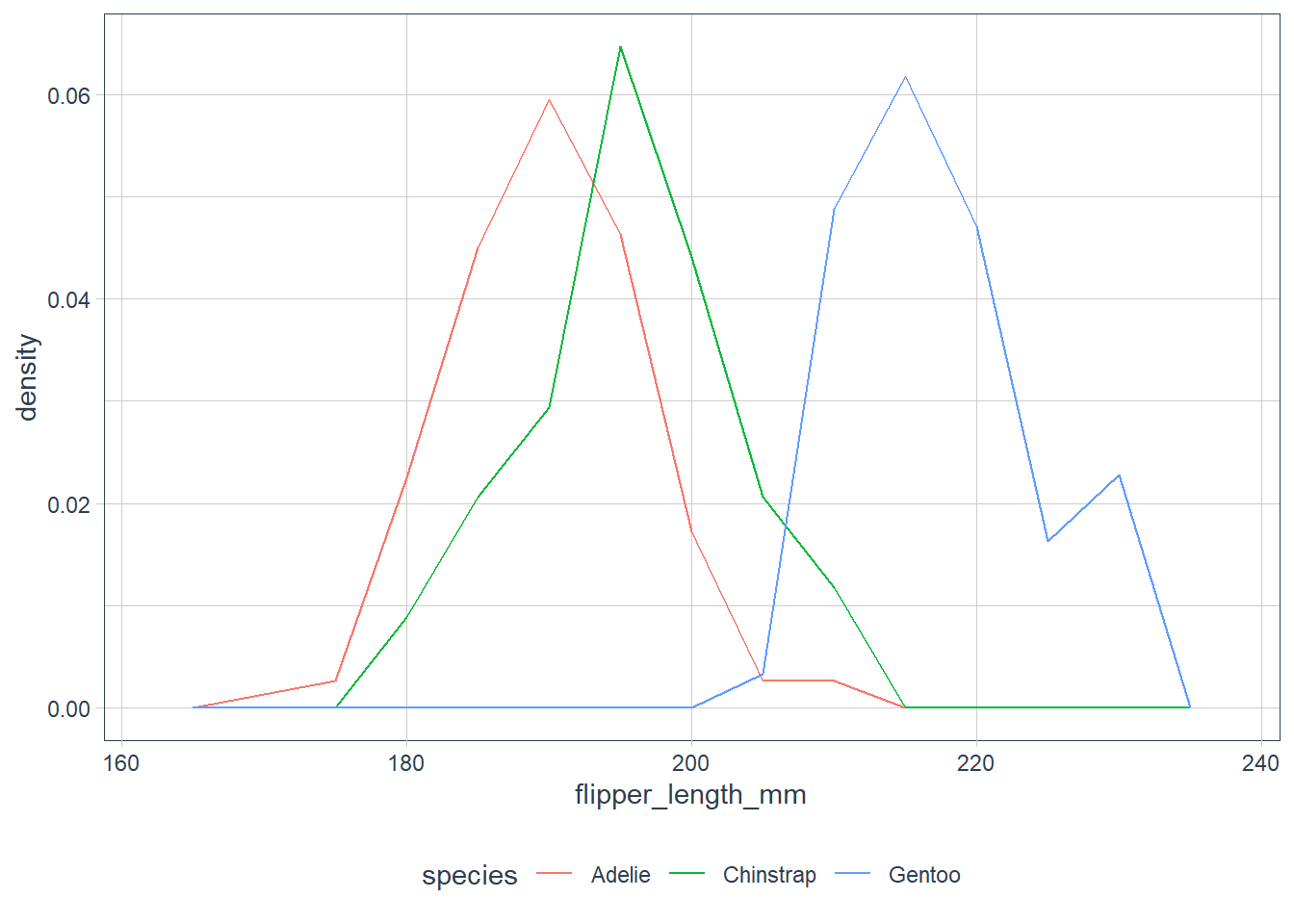#### Boxplots Verbatim from the book: • A box that stretches from the 25th percentile of the distribution to the 75th percentile, a distance known as the interquartile range (IQR). In the middle of the box is a line that displays the median, i.e. 50th percentile, of the distribution. These three lines give you a sense of the spread of the distribution and whether or not the distribution is symmetric about the median or skewed to one side. • Visual points that display observations that fall more than 1.5 times the IQR from either edge of the box. These outlying points are unusual so are plotted individually. • A line (or whisker) that extends from each end of the box and goes to the farthest non-outlier point in the distribution.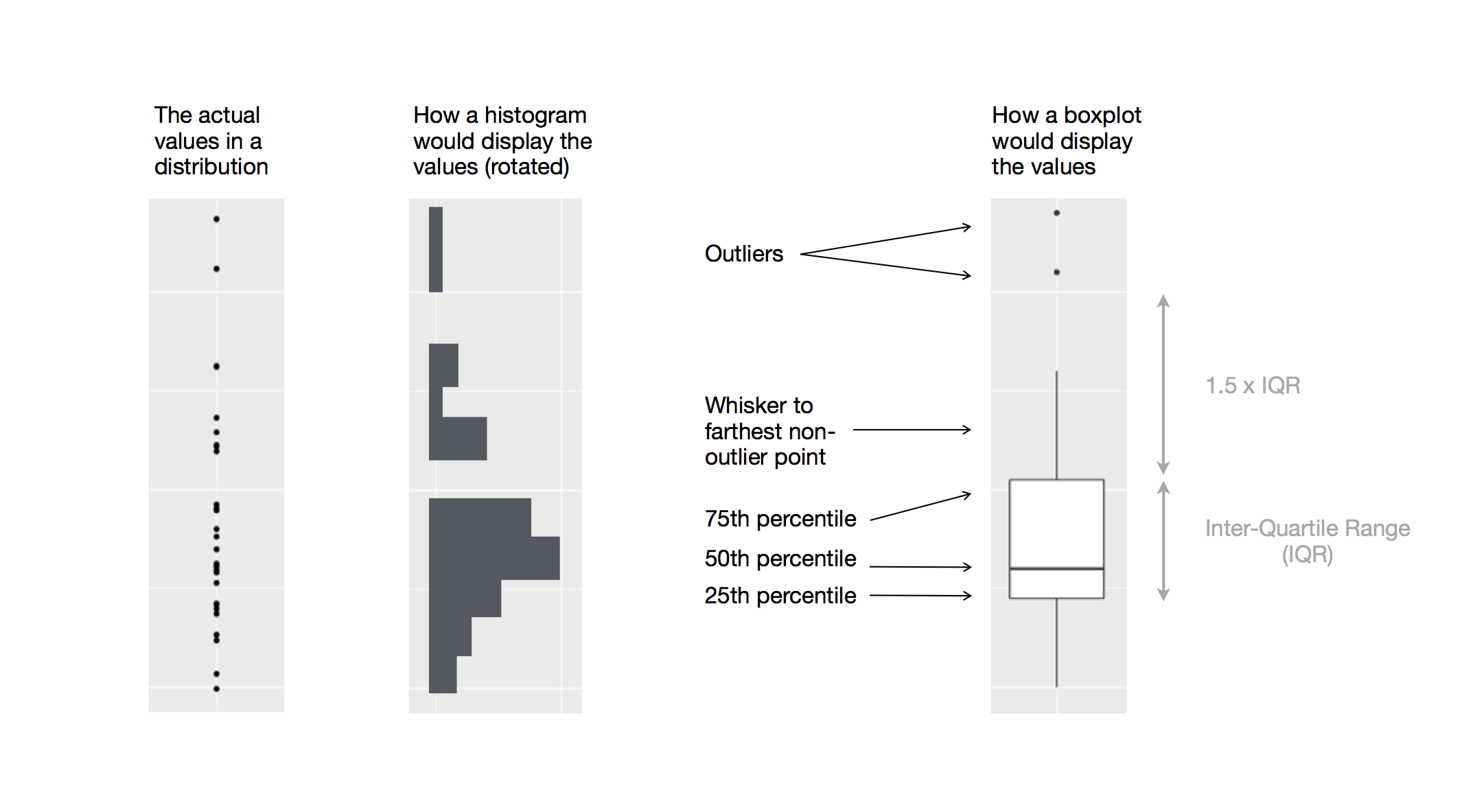Credit: R for Data Science ggplot(penguins, aes(x = species, y = body_mass_g)) + geom_boxplot()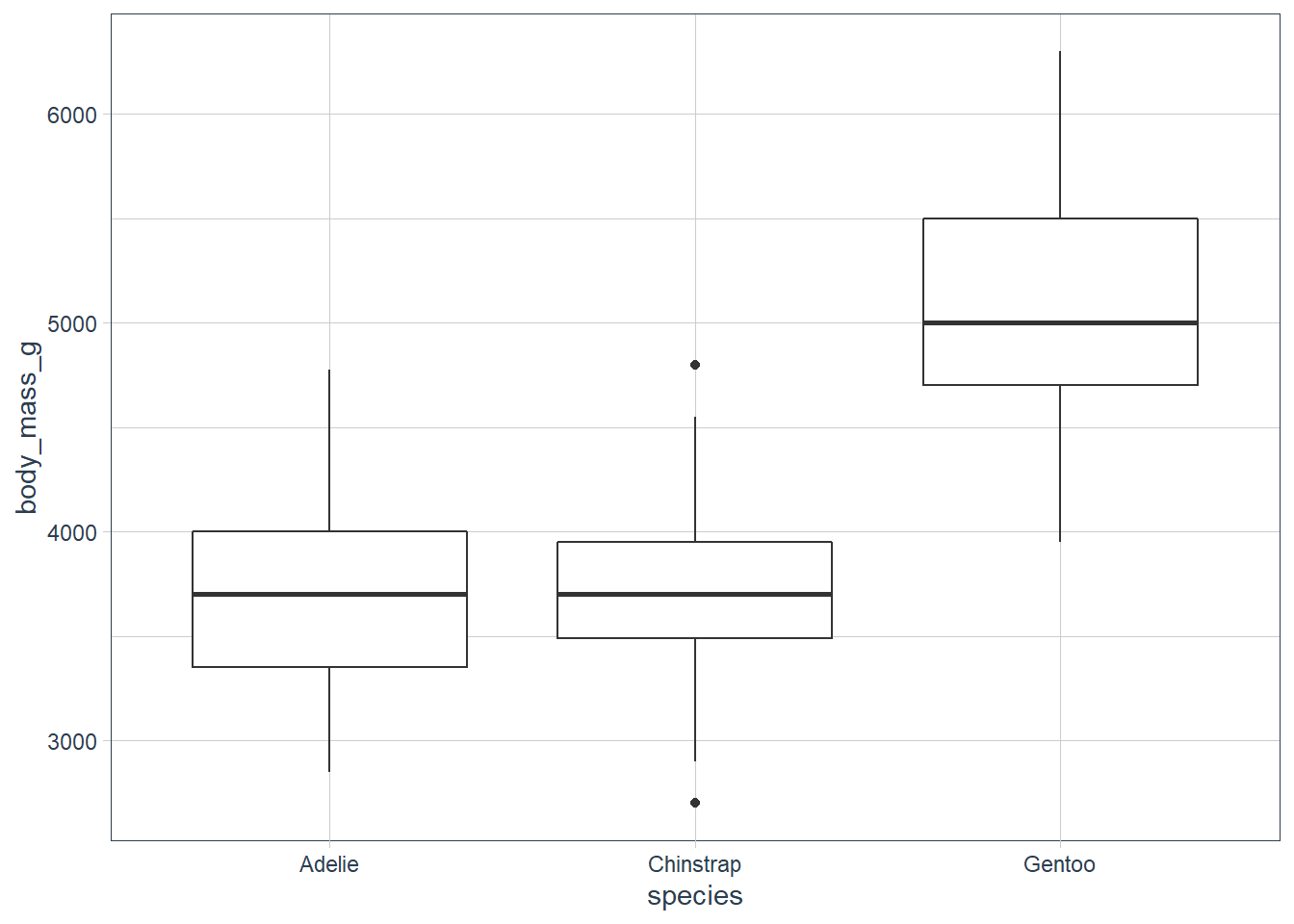ggplot(penguins, aes(x = body_mass_g, y = species)) + geom_boxplot()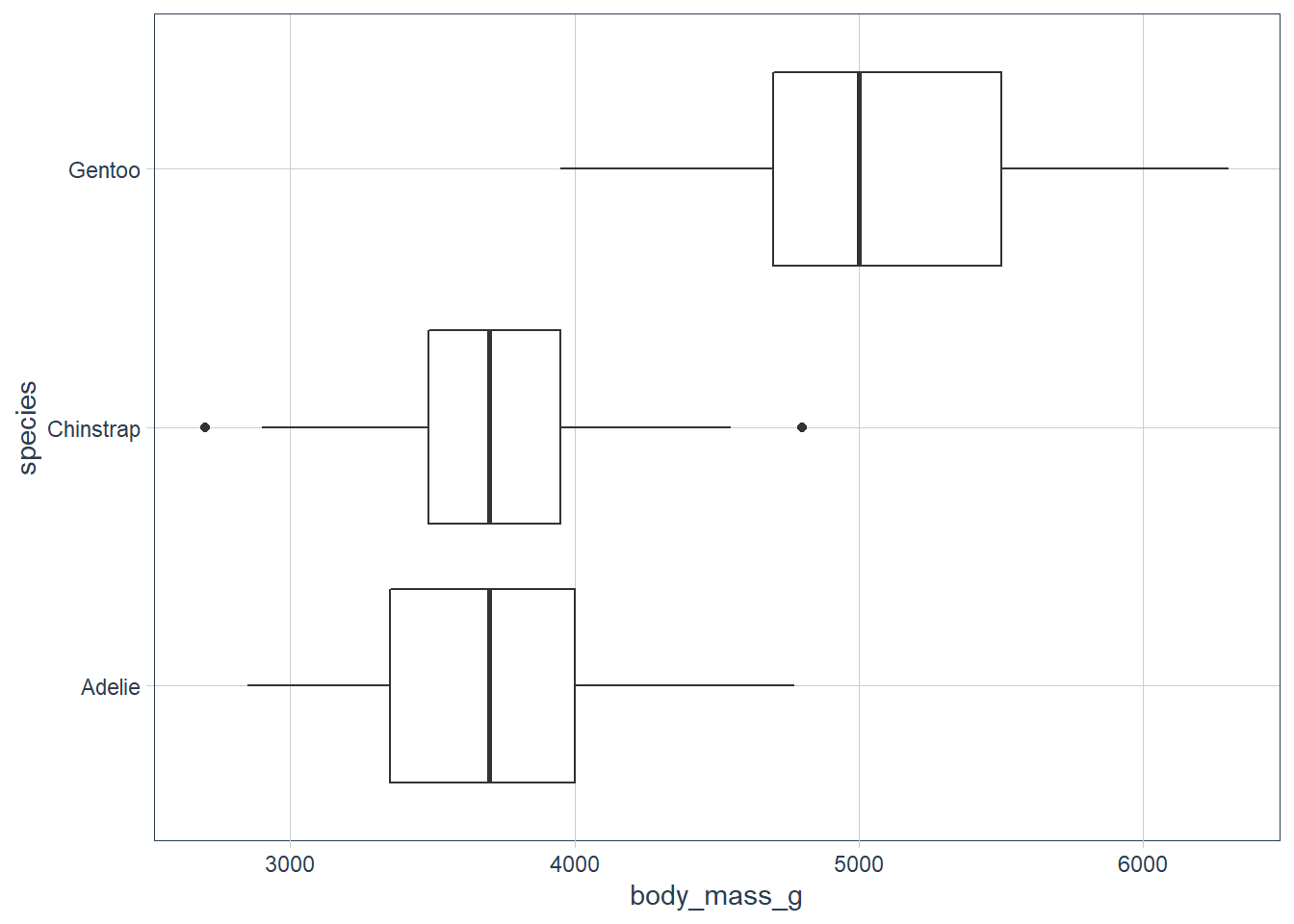ggplot(penguins, aes(x = bill_length_mm, y = species)) + geom_boxplot()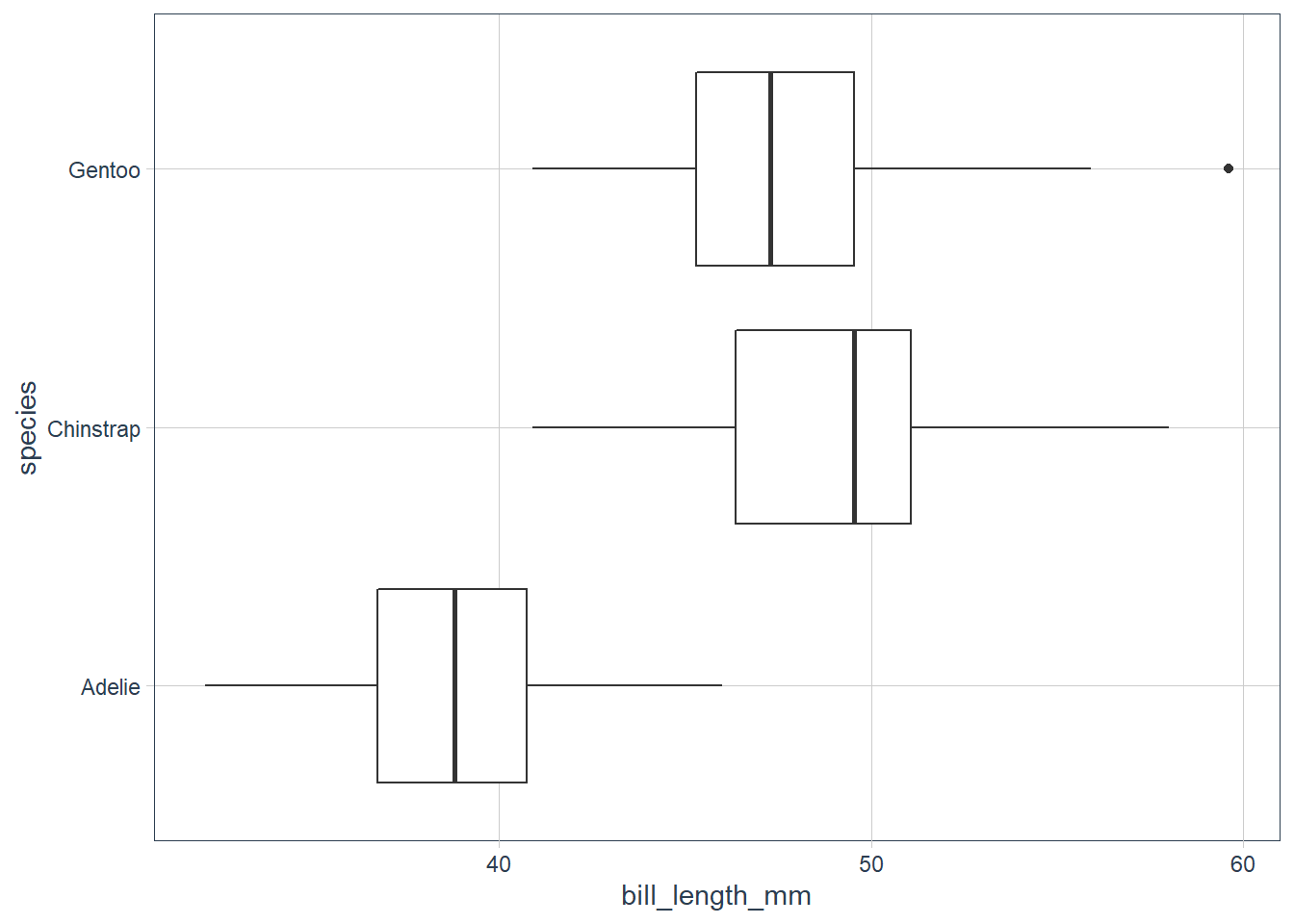ggplot(penguins, aes(x = flipper_length_mm, y = species)) + geom_boxplot()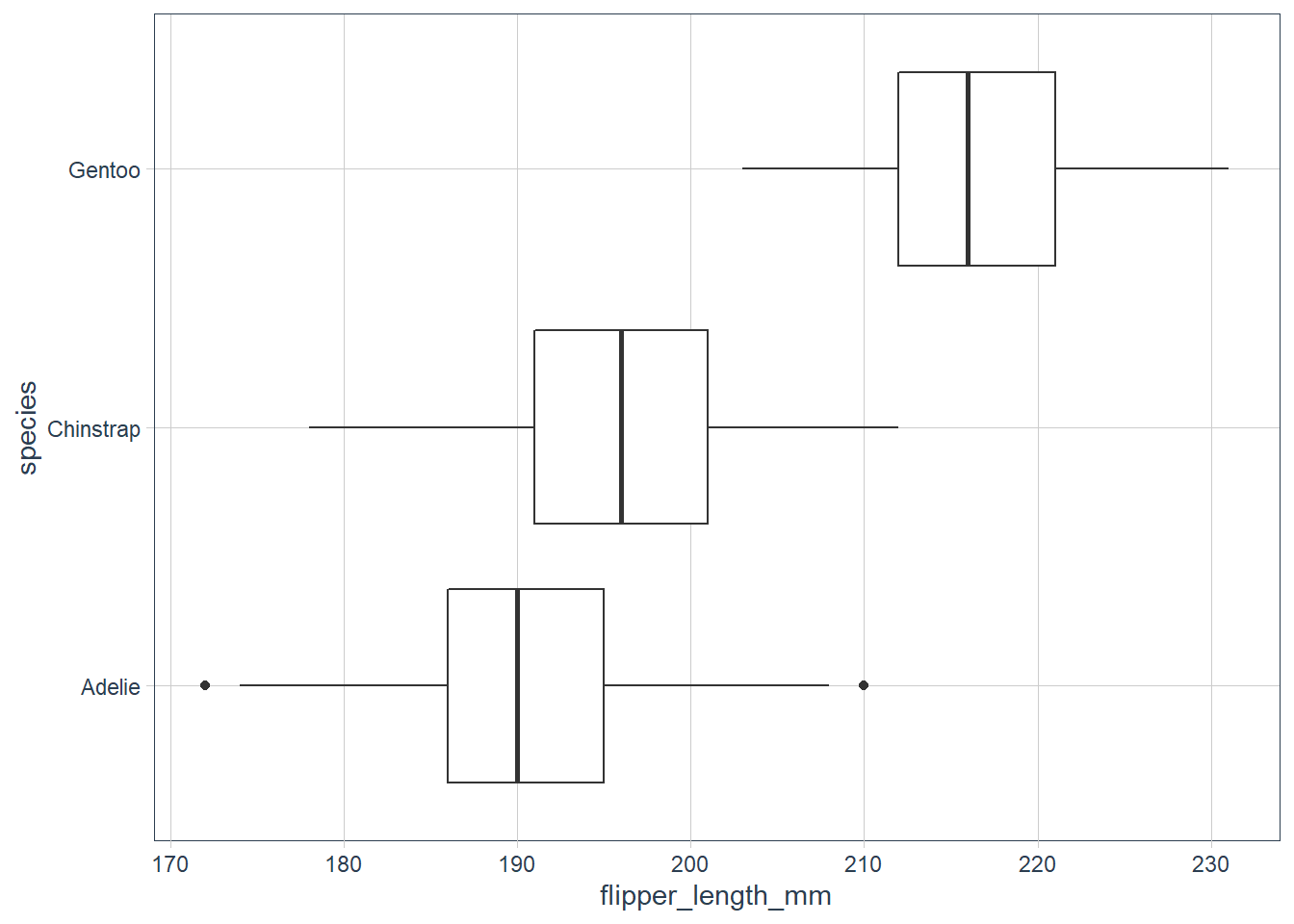# the reorder does not seem to be working for meggplot(penguins, aes(y = bill_depth_mm, x = reorder(species, bill_depth_mm, FUN = median))) + geom_boxplot()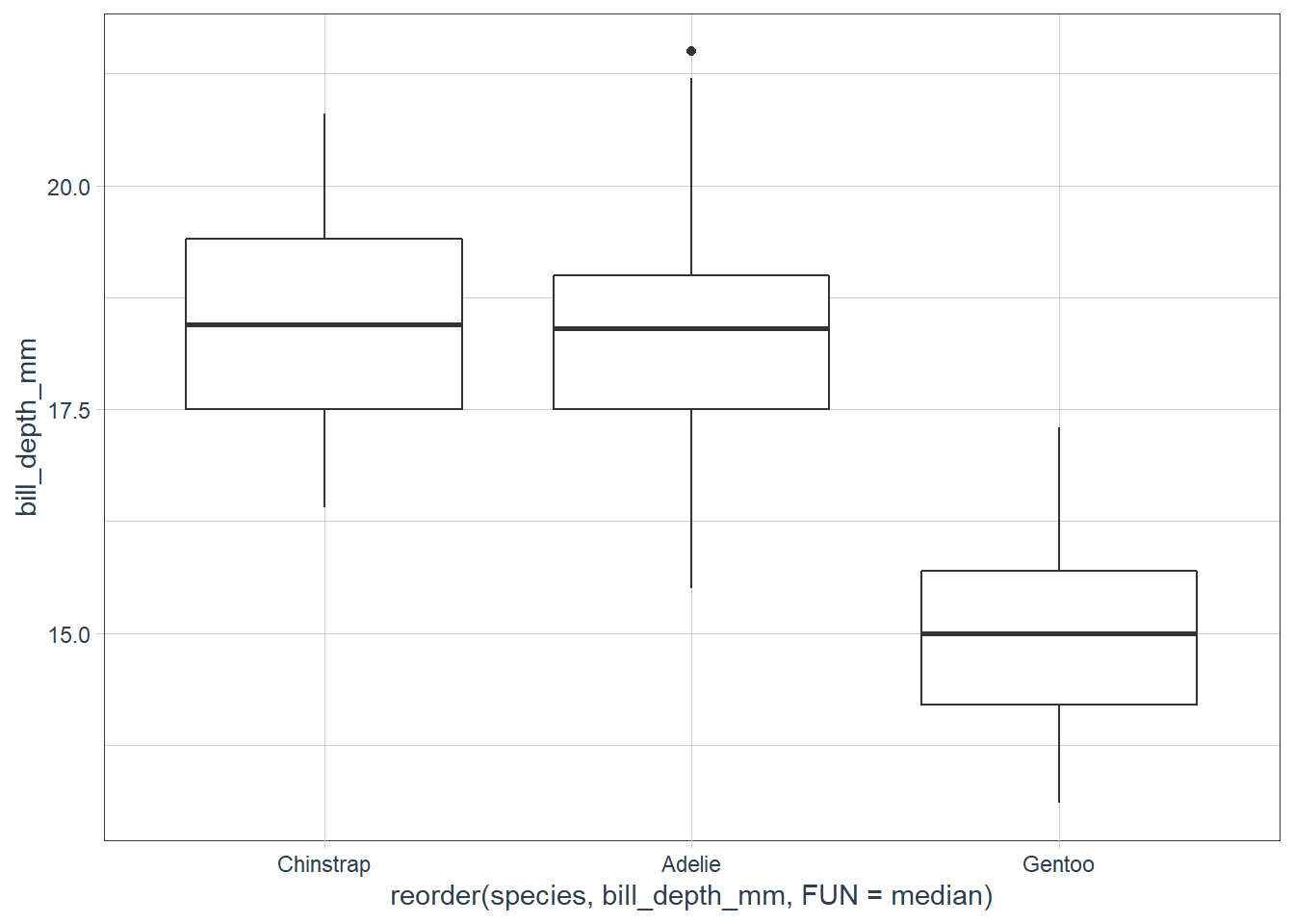### Exercises 1. Use what you’ve learned to improve the visualisation of the departure times of cancelled vs. non-cancelled flights. nycflights13::flights %>% mutate(cancelled = is.na(dep_time), sched_hour = sched_dep_time %/% 100, sched_min = sched_dep_time %/% 100, sched_dep_time = sched_hour + sched_min / 60) %>% ggplot(aes(x = sched_dep_time, y = cancelled)) + geom_boxplot()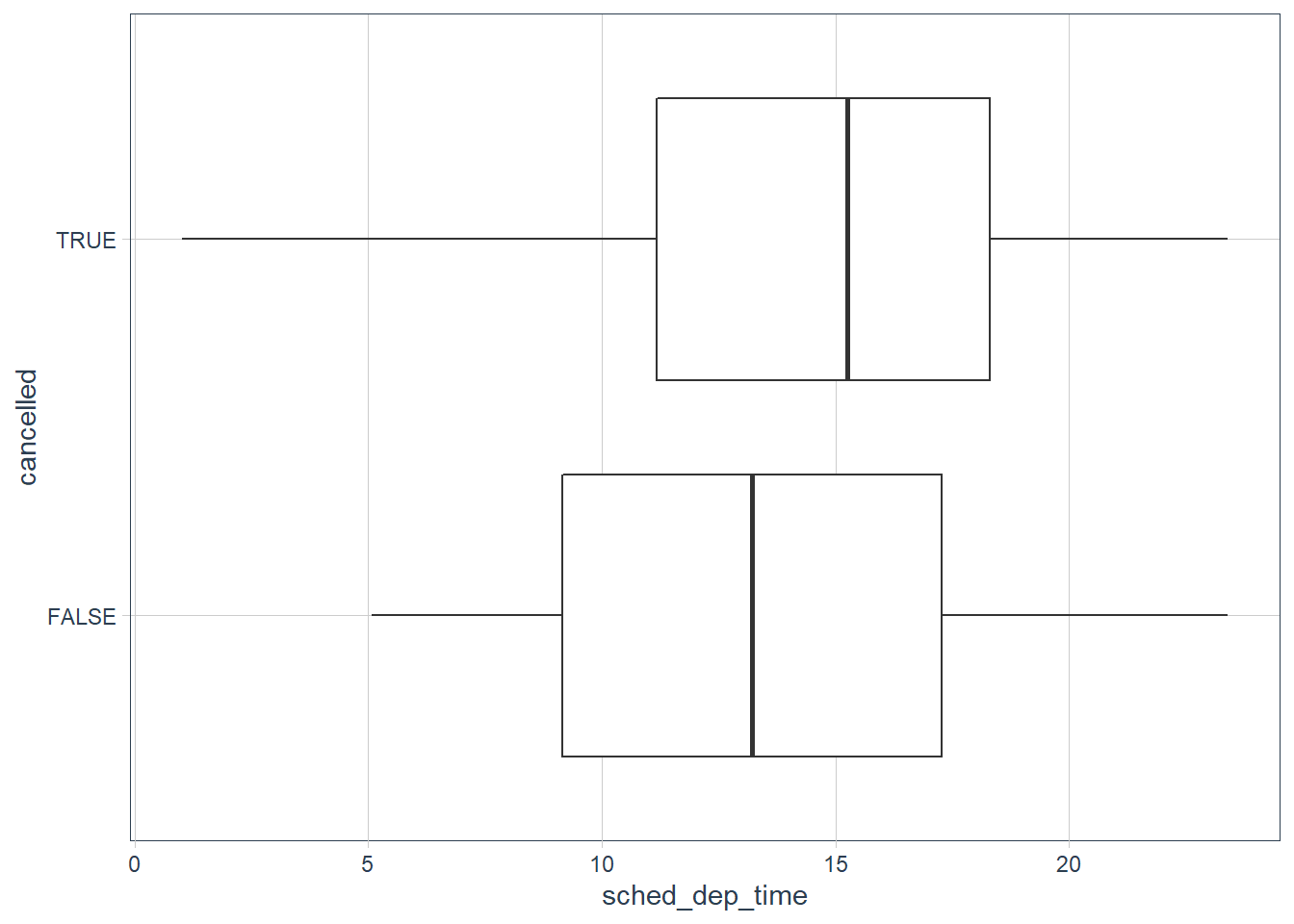2. What variable in the diamonds dataset is most important for predicting the price of a diamond? How is that variable correlated with cut? Why does the combination of those two relationships lead to lower quality diamonds being more expensive? ggplot(diamonds, aes(x = carat, y = price)) + geom_point()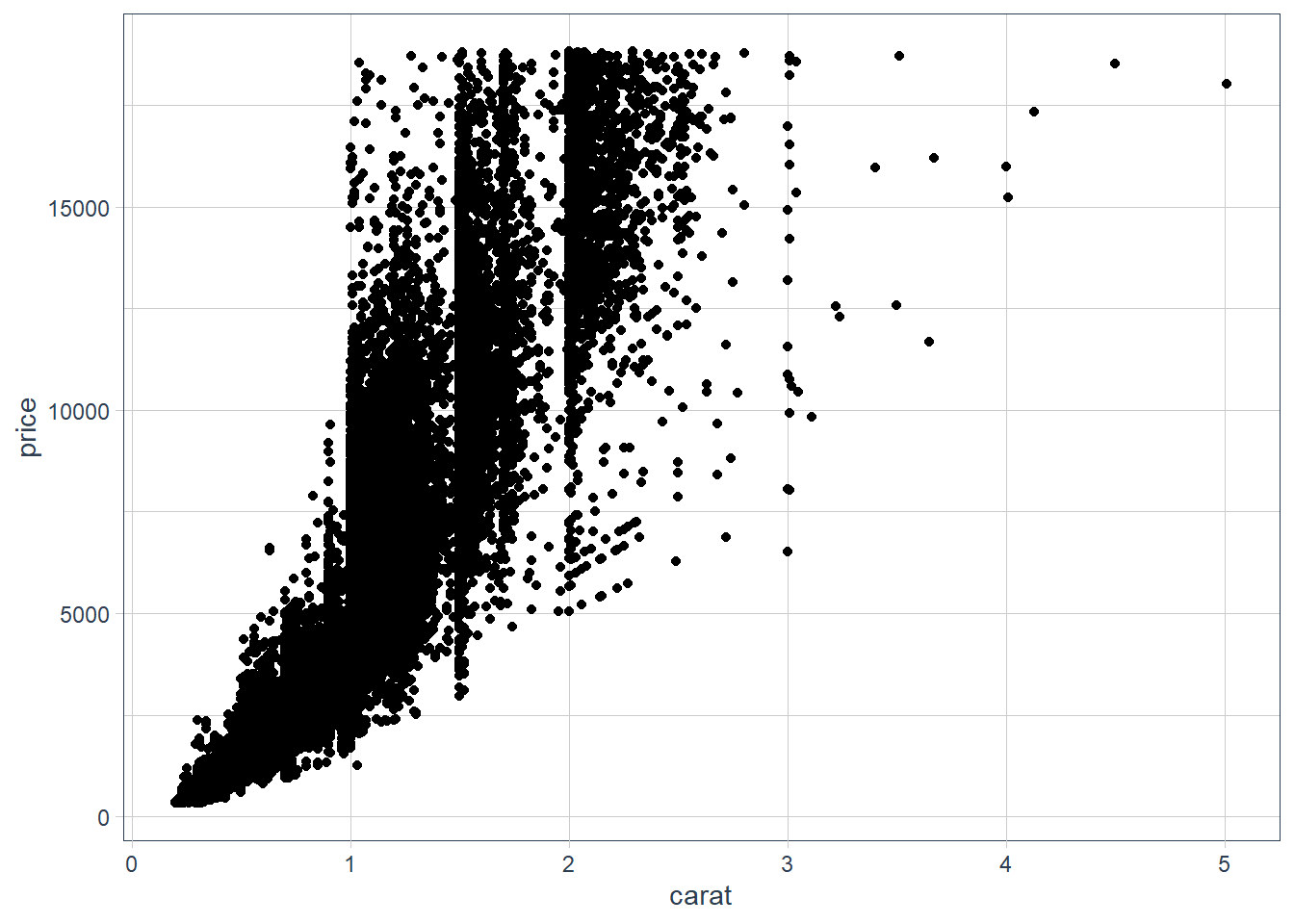ggplot(diamonds, aes(x = reorder(cut, price, median), y = price)) + geom_boxplot()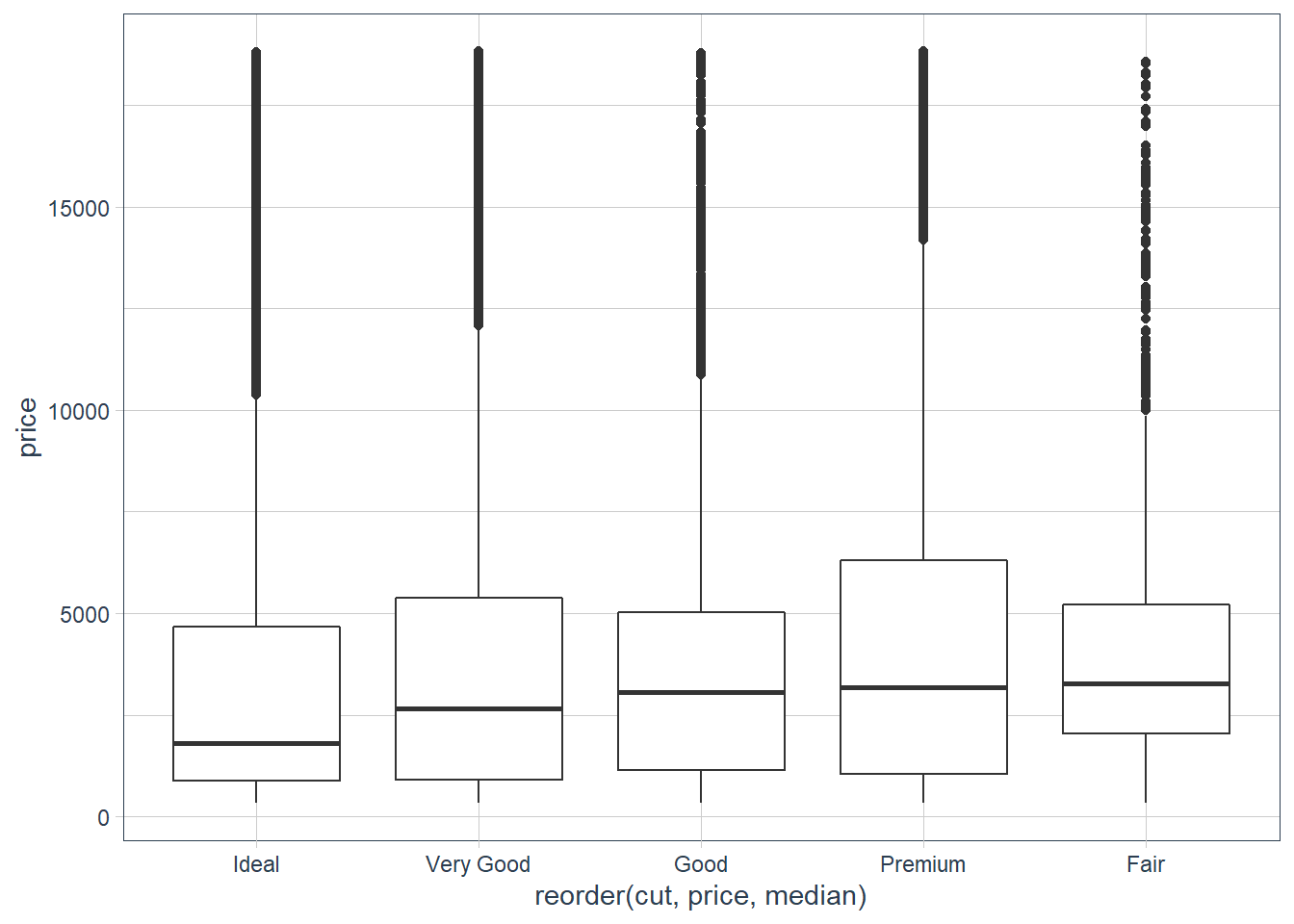ggplot(diamonds, aes(x = reorder(color, price, median), y = price)) + geom_boxplot()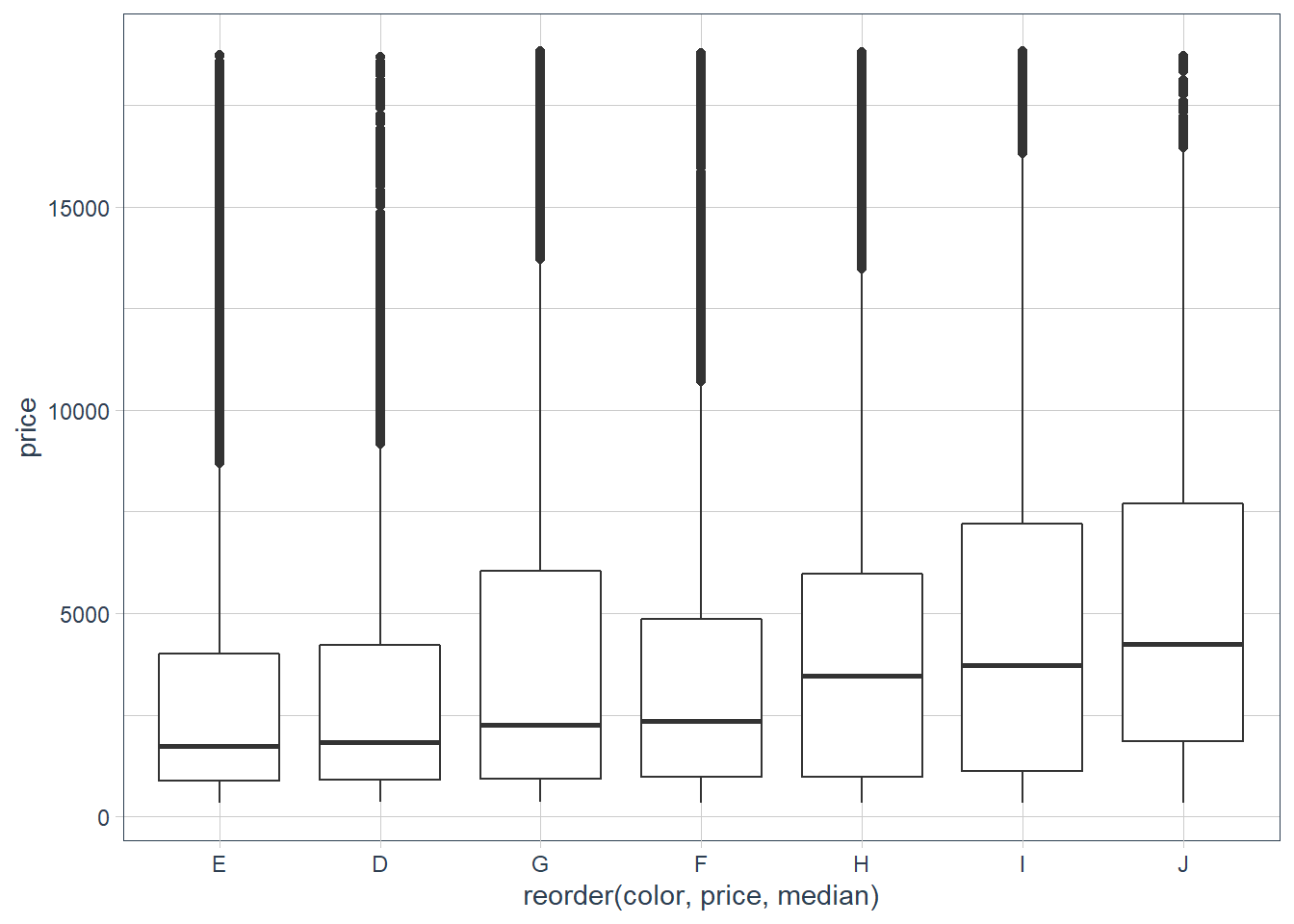ggplot(diamonds, aes(x = reorder(clarity, price, median), y = price)) + geom_boxplot()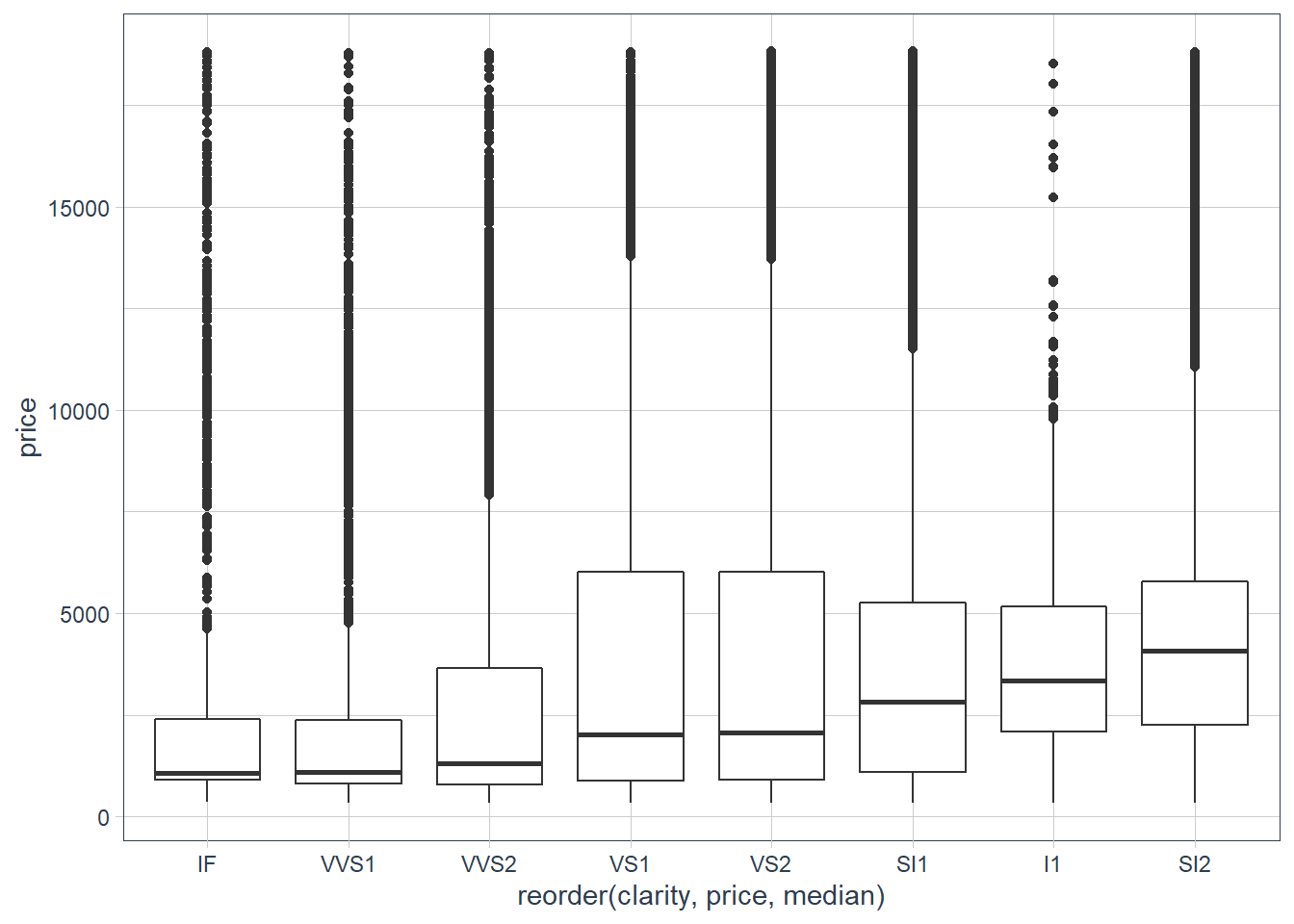ggplot(diamonds, aes(x = reorder(cut, carat, median), y = carat)) + geom_boxplot()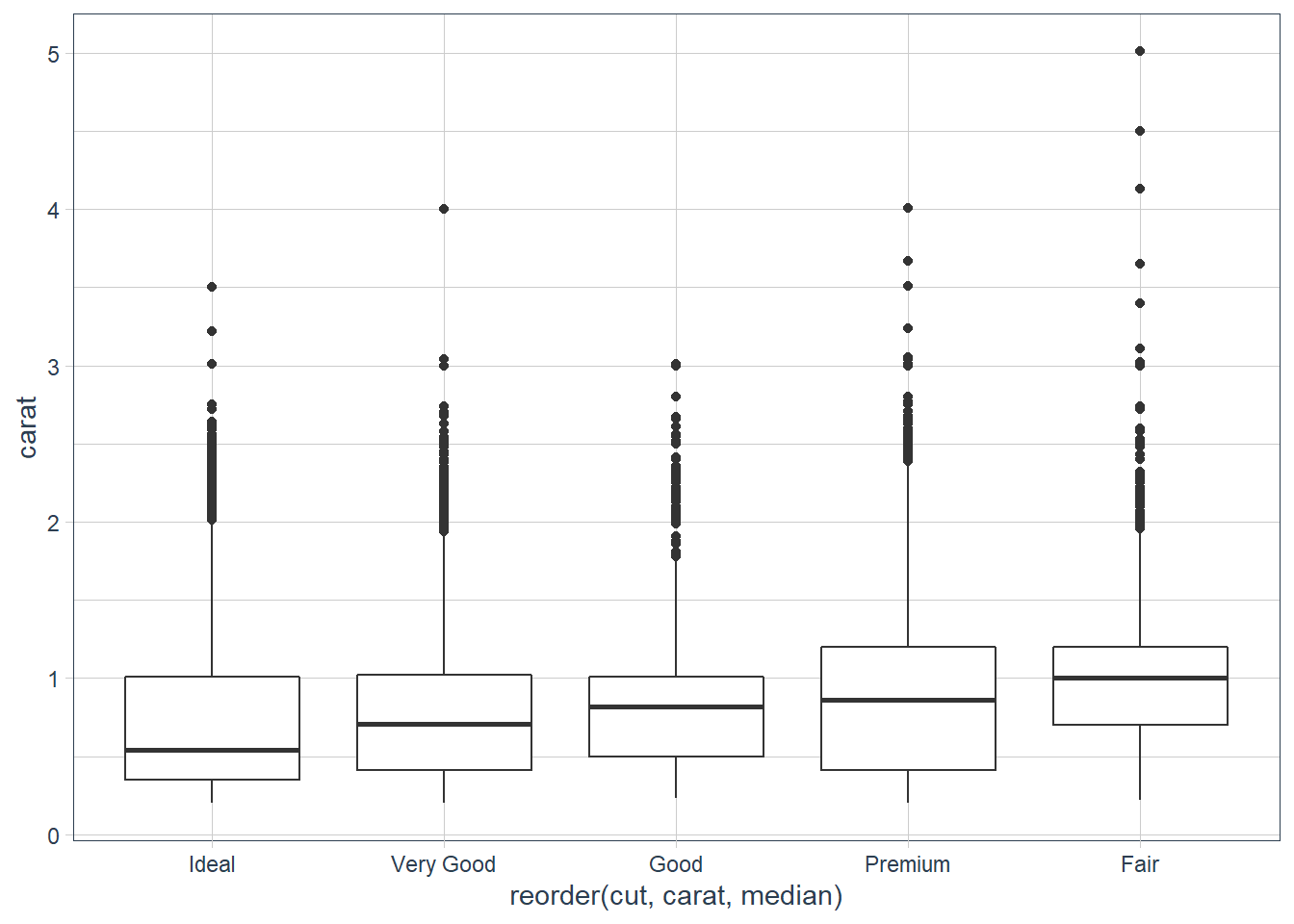ggplot(diamonds, aes(x = reorder(color, carat, median), y = carat)) + geom_boxplot()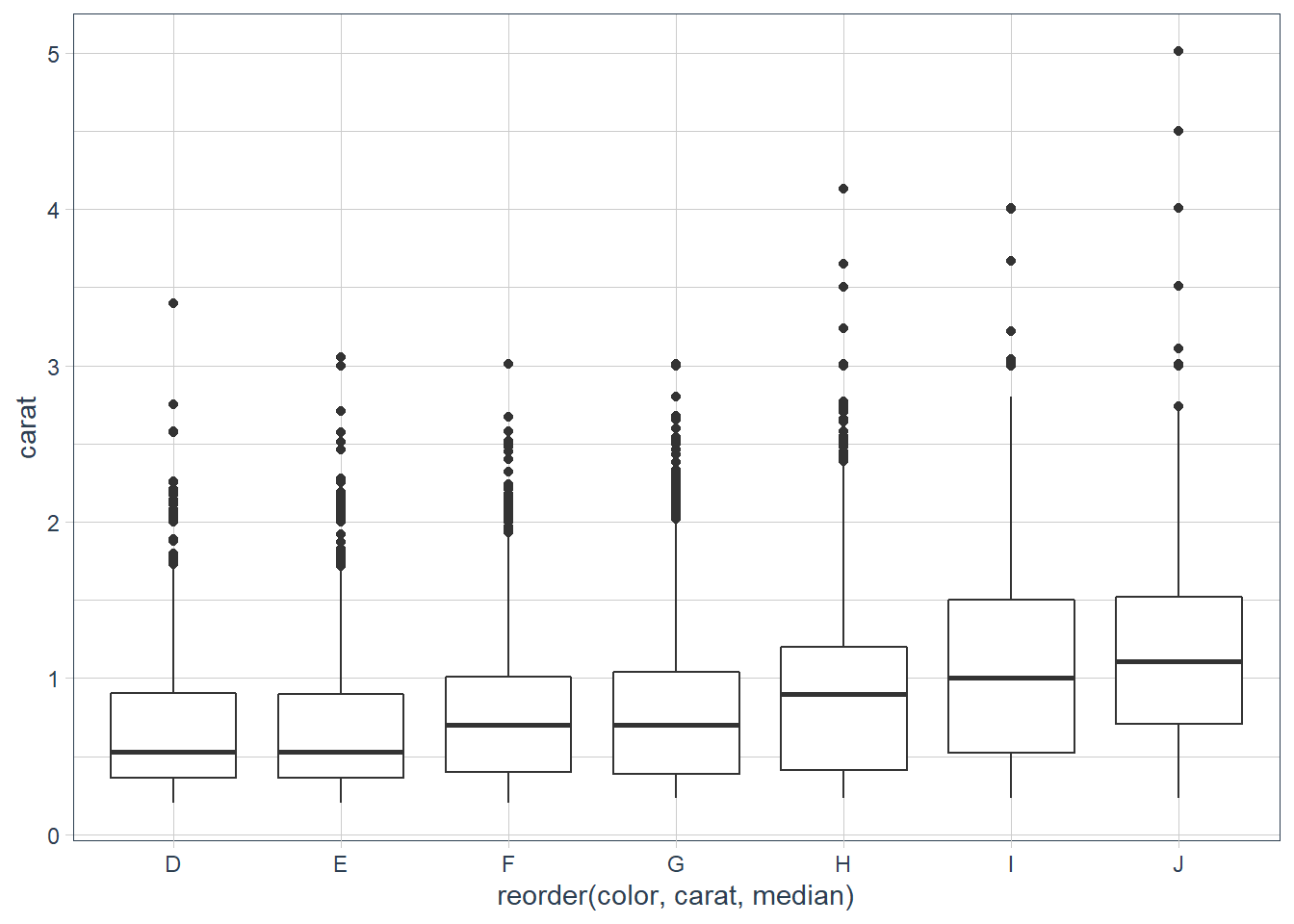ggplot(diamonds, aes(x = reorder(clarity, carat, median), y = carat)) + geom_boxplot()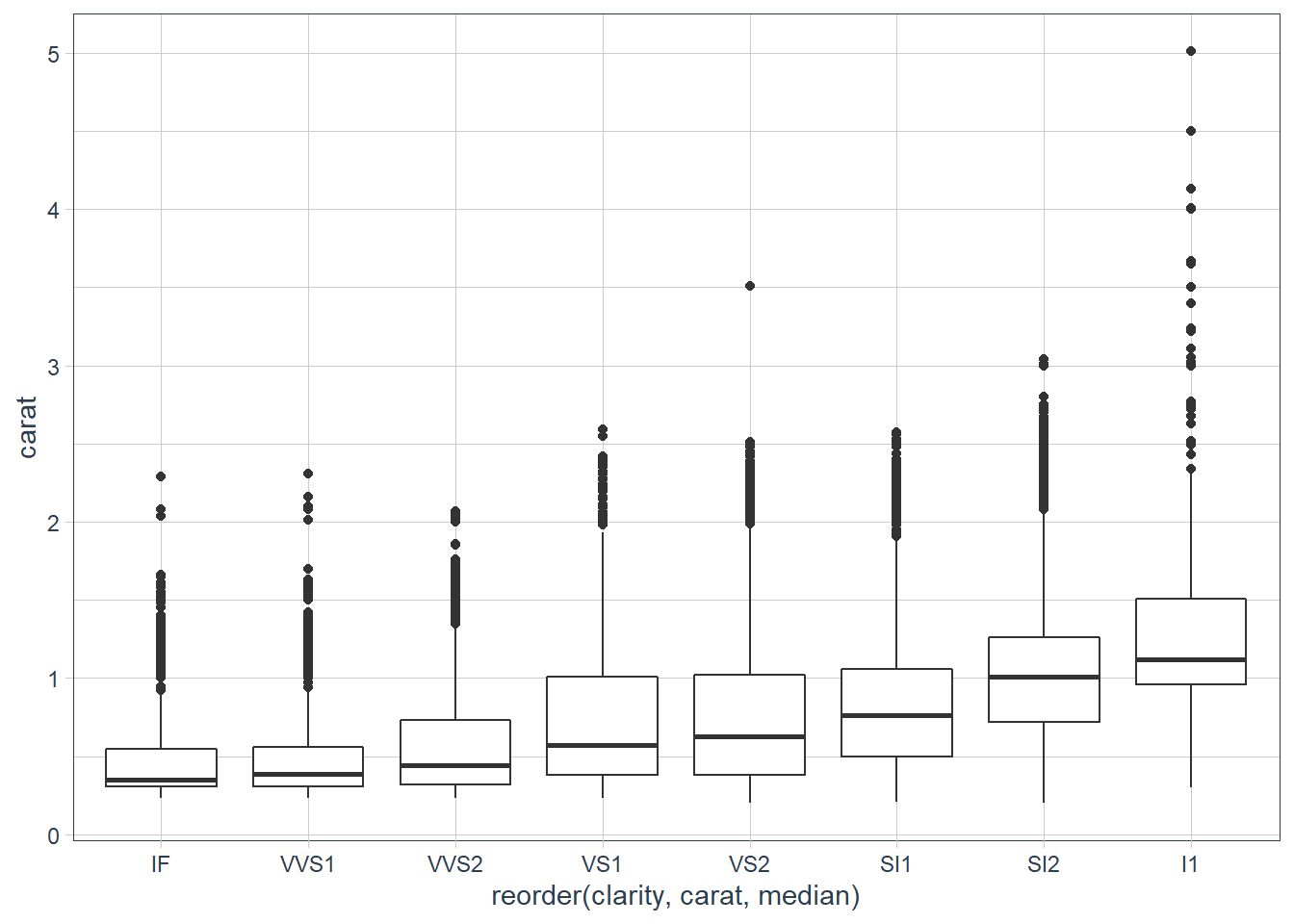cor(diamonds$price, diamonds\$carat)
 0.9215913

They say that there are 4 characteristics of diamonds that come into play when considering the quality - Carat Weight, Cut, Colour and Clarity. It looks like carat, is most correlated with the price, and there are quite a few low quality for the Cut of the diamonds that have a big carat weight.

3. Install the ggstance package, and create a horizontal boxplot. How does this compare to using coord_flip()?

There is no longer a need to use the ggstance 📦 as this functionality is provided in ggplot2 since version 3.3.0.

4. One problem with boxplots is that they were developed in an era of much smaller datasets and tend to display a prohibitively large number of “outlying values”. One approach to remedy this problem is the letter value plot. Install the lvplot package, and try using geom_lv() to display the distribution of price vs cut. What do you learn? How do you interpret the plots?

library(lvplot)
ggplot(diamonds, aes(reorder(cut,
price, median), price)) +
geom_boxplot() +
labs(x = "Cut",
y = "Price")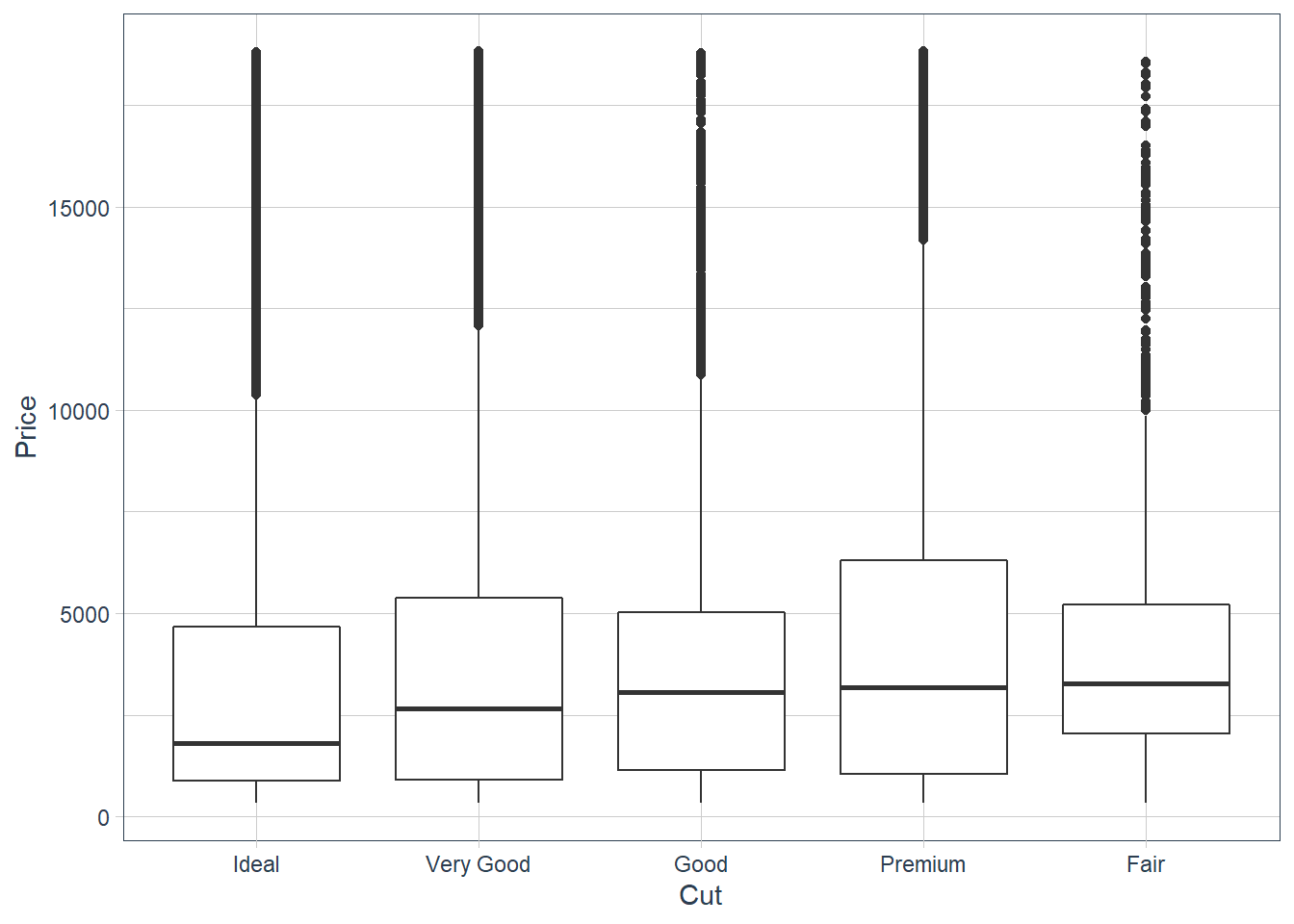ggplot(diamonds, aes(reorder(cut, price,
median), price)) +
geom_lv(outlier.colour = "darkgreen") +
labs(x = "Cut", y = "Price")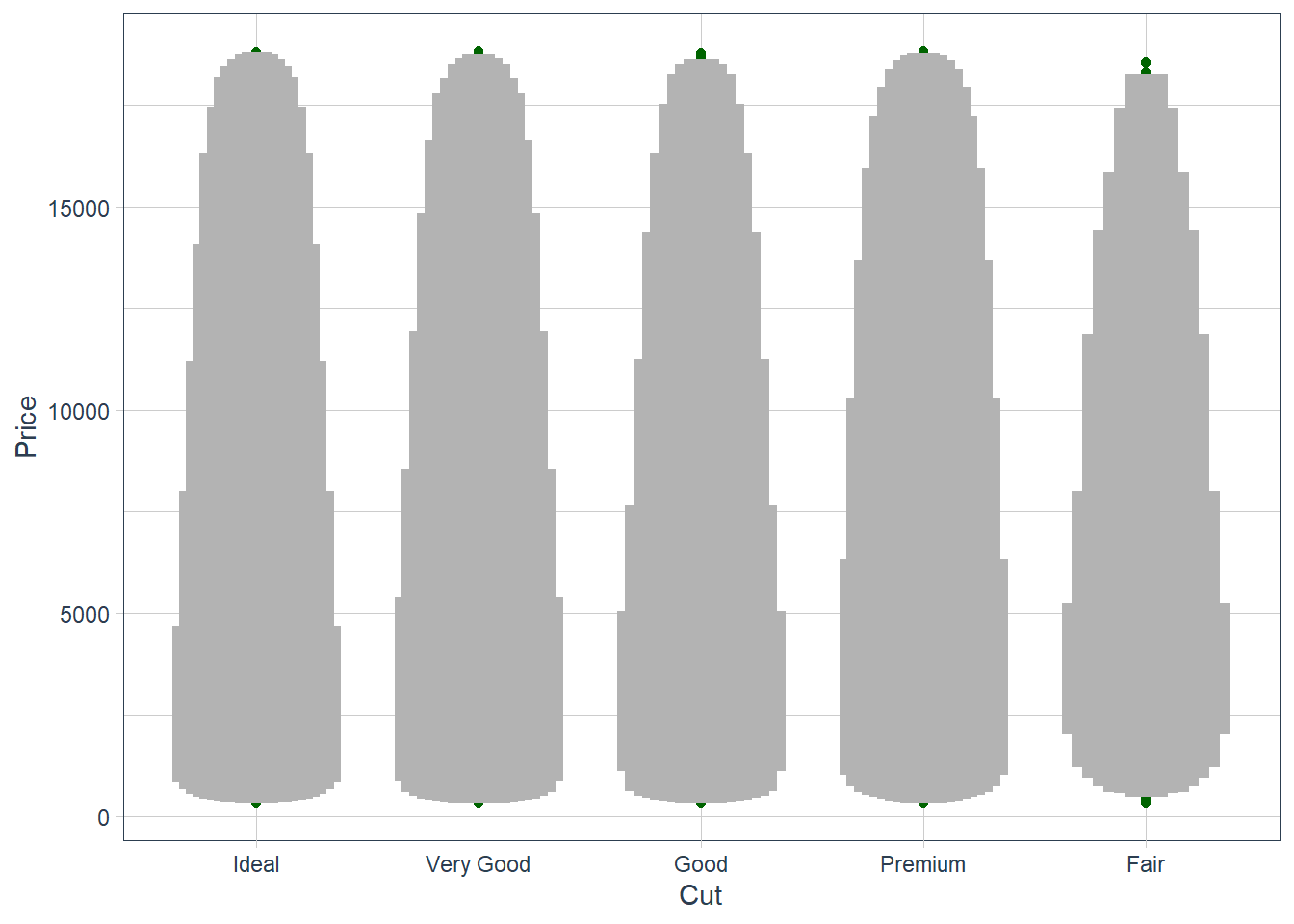ggplot(diamonds, aes(reorder(cut, price,
median), price)) +
geom_lv(aes(fill = ..LV..), outlier.colour = "darkgreen",
k = 6) +
labs(x = "Cut", y = "Price") +
scale_fill_lv()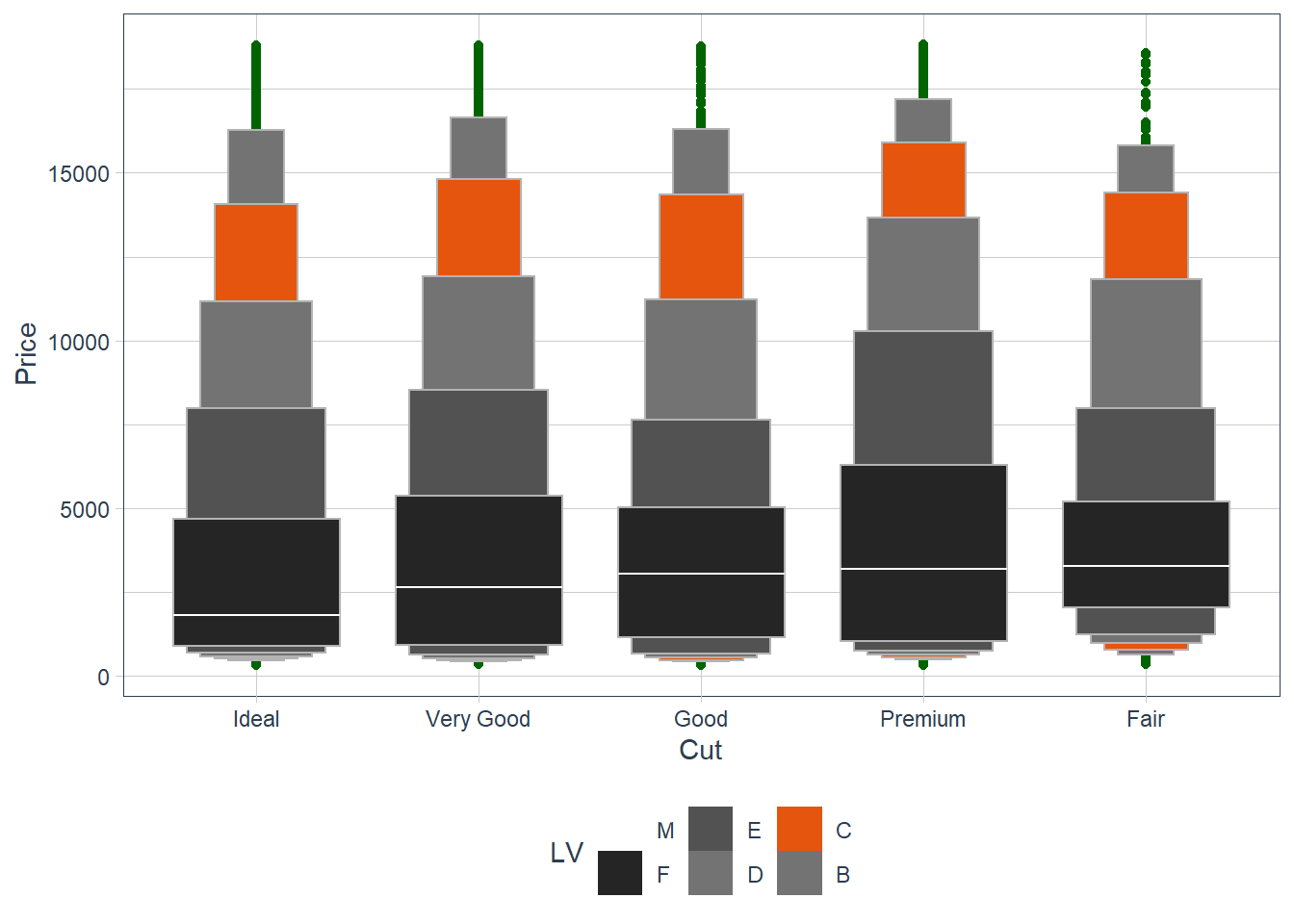The plots are an extension of the boxplots and allows you to see the distribution better especially for large datasets. More information on the plot can be found here.

5. Compare and contrast geom_violin() with a facetted geom_histogram(), or a coloured geom_freqpoly(). What are the pros and cons of each method?

ggplot(penguins, aes(x = species, body_mass_g)) +
geom_violin(aes(colour = species))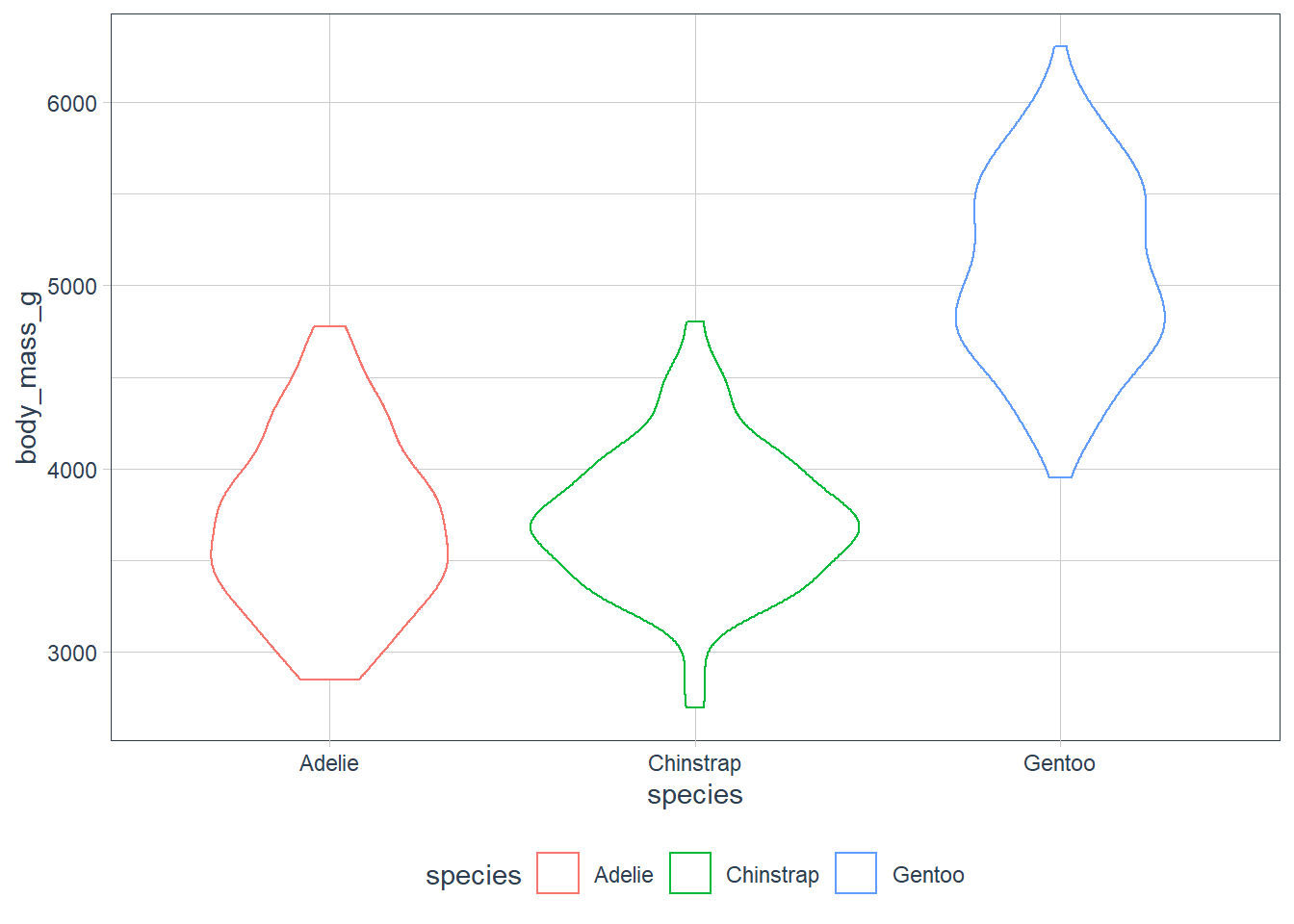ggplot(penguins, aes(x = body_mass_g)) +
geom_histogram() +
facet_wrap(~ species,
ncol = 1,
scales = "free_y")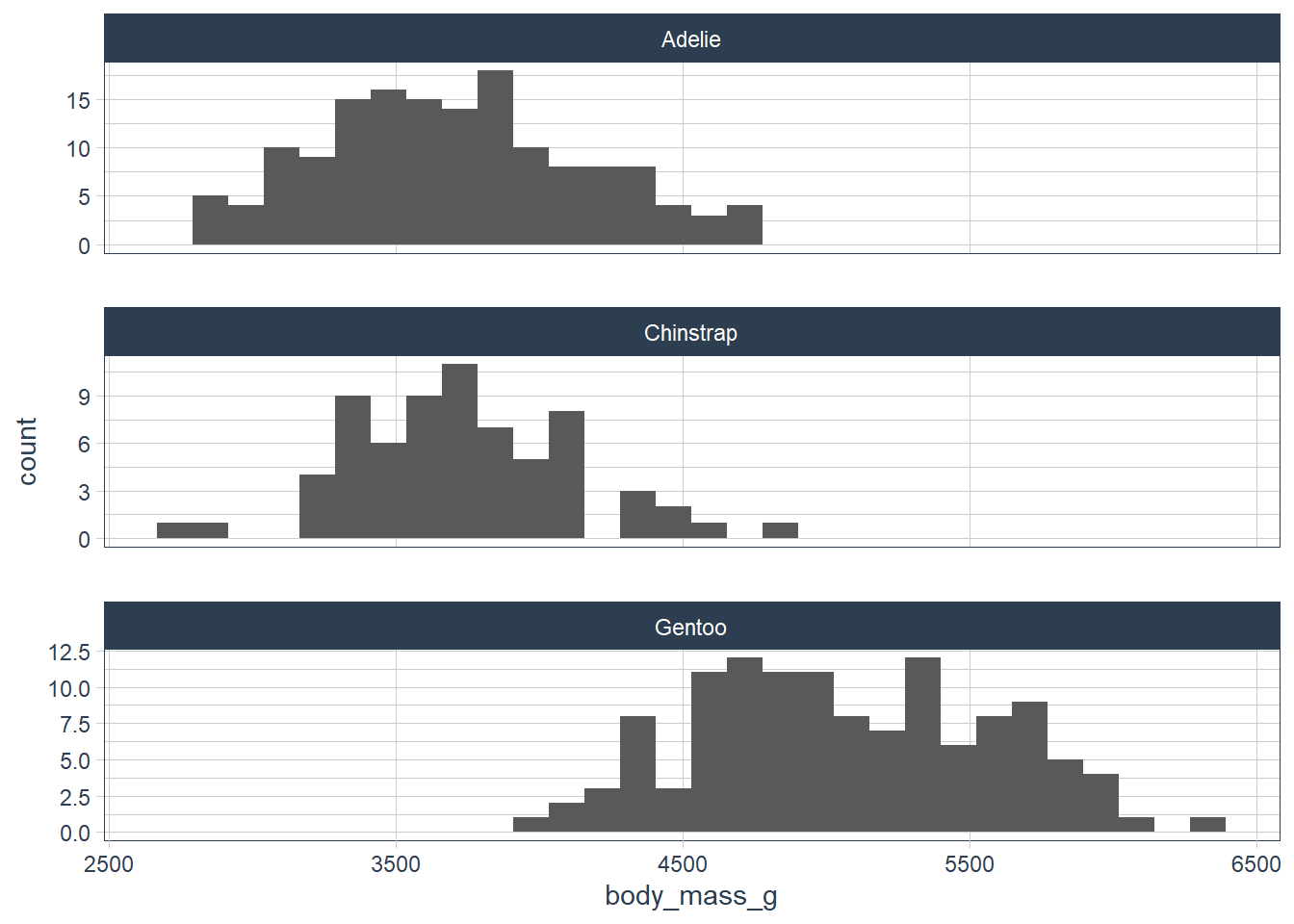ggplot(penguins, aes(x = body_mass_g, y = ..density..)) +
geom_freqpoly(aes(colour = species), binwidth = 200)They’re all pretty similar, but I think the geom_freqpoly() and geom_violin() use density and hence is harder to interpret.

6. If you have a small dataset, it’s sometimes useful to use geom_jitter() to see the relationship between a continuous and categorical variable. The ggbeeswarm package provides a number of methods similar to geom_jitter(). List them and briefly describe what each one does.

library(ggbeeswarm)
ggplot(penguins, aes(x = species, body_mass_g)) +
geom_quasirandom()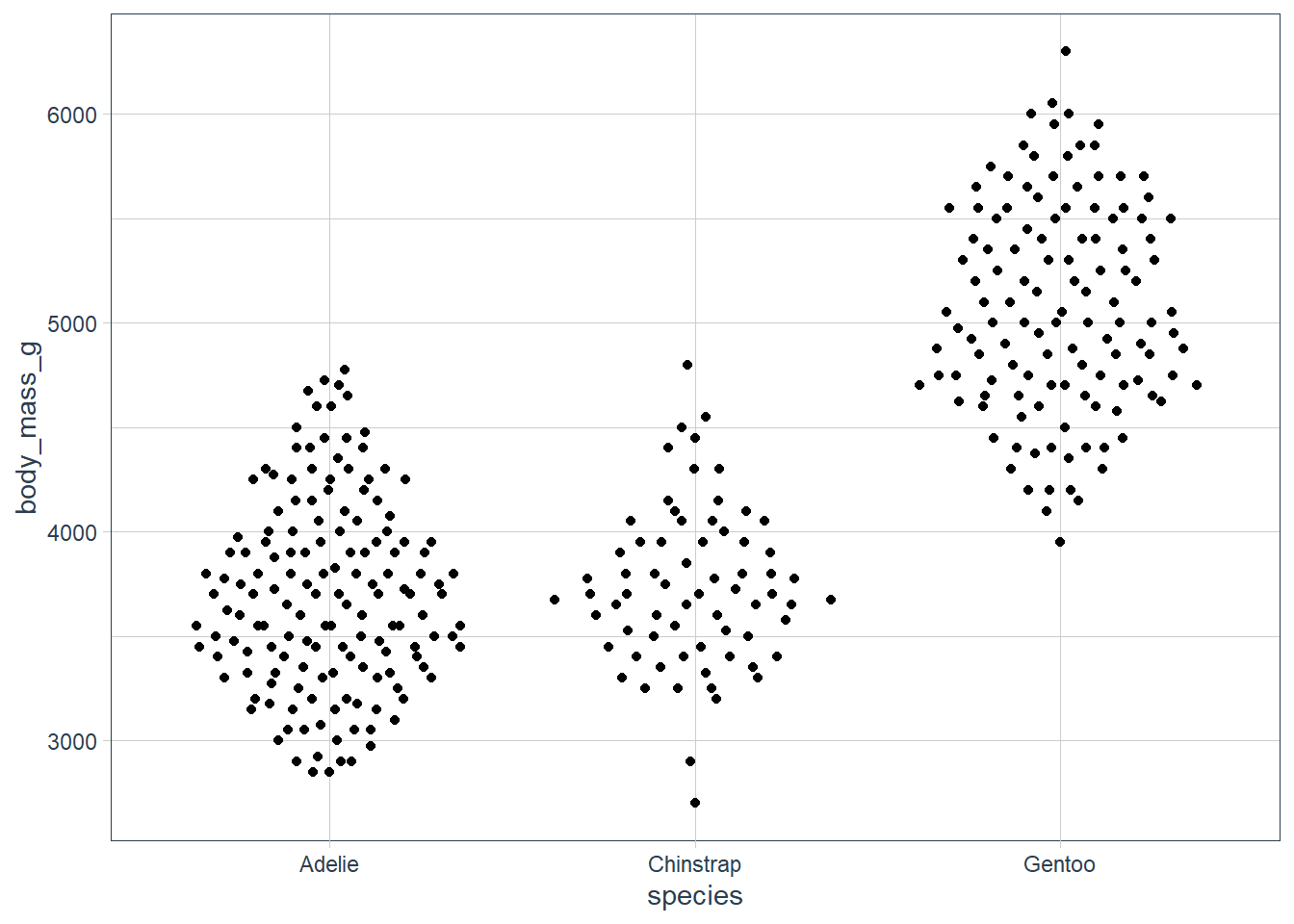ggplot(penguins, aes(x = species, body_mass_g)) +
geom_beeswarm()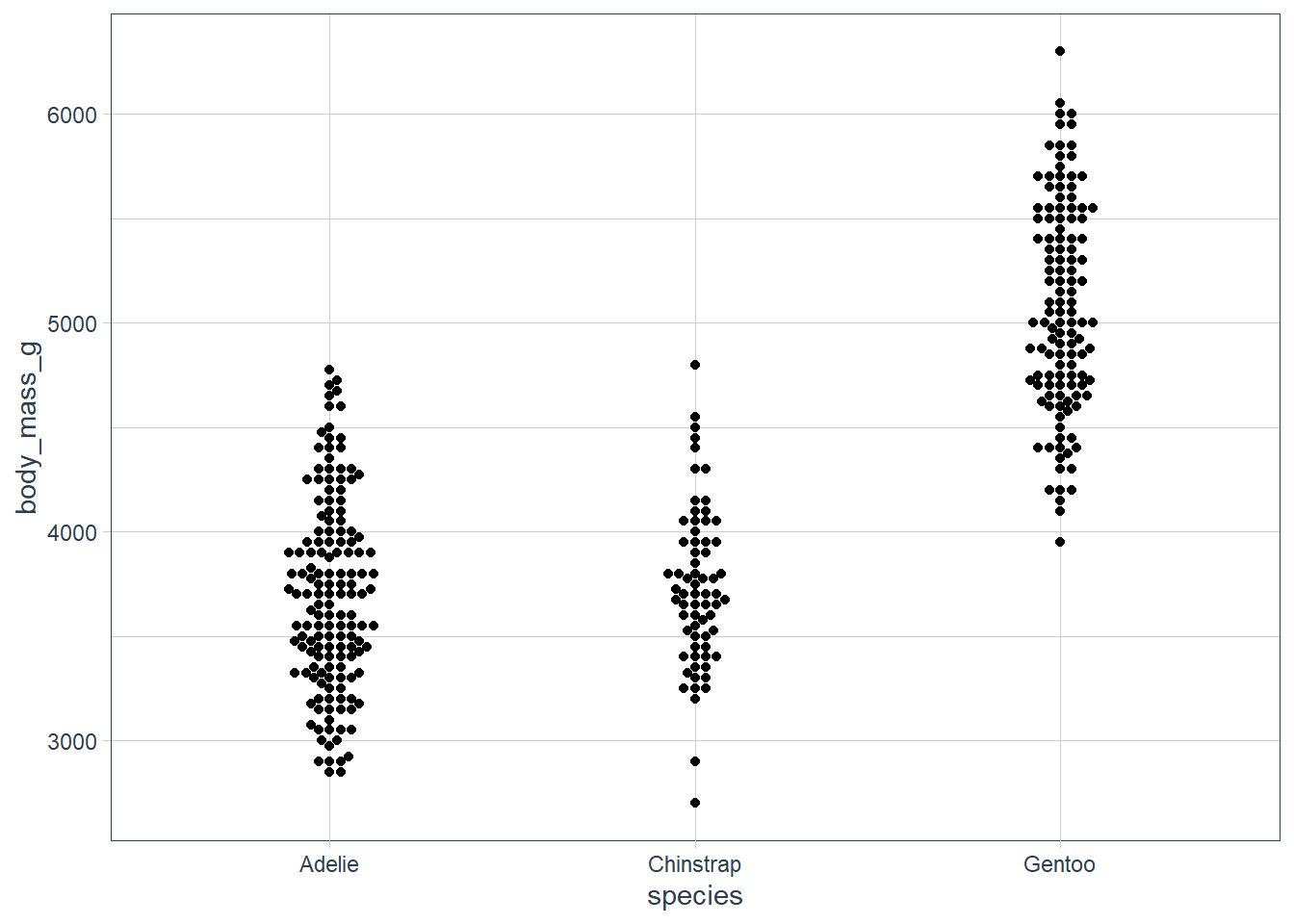ggplot(penguins, aes(x = species, bill_depth_mm)) +
geom_beeswarm()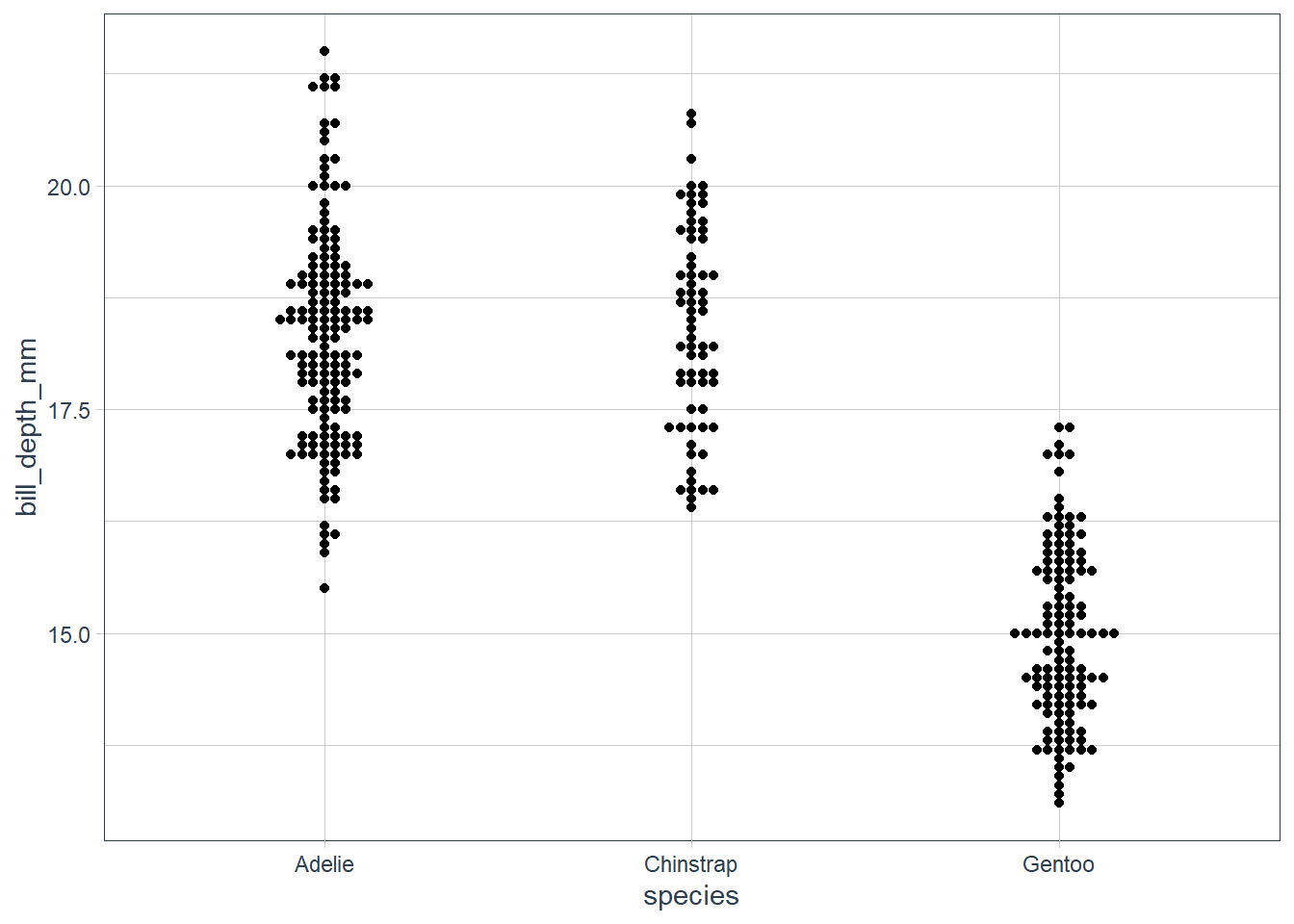ggplot(diamonds, aes(x = reorder(cut, price,
median), y = price)) +
geom_quasirandom()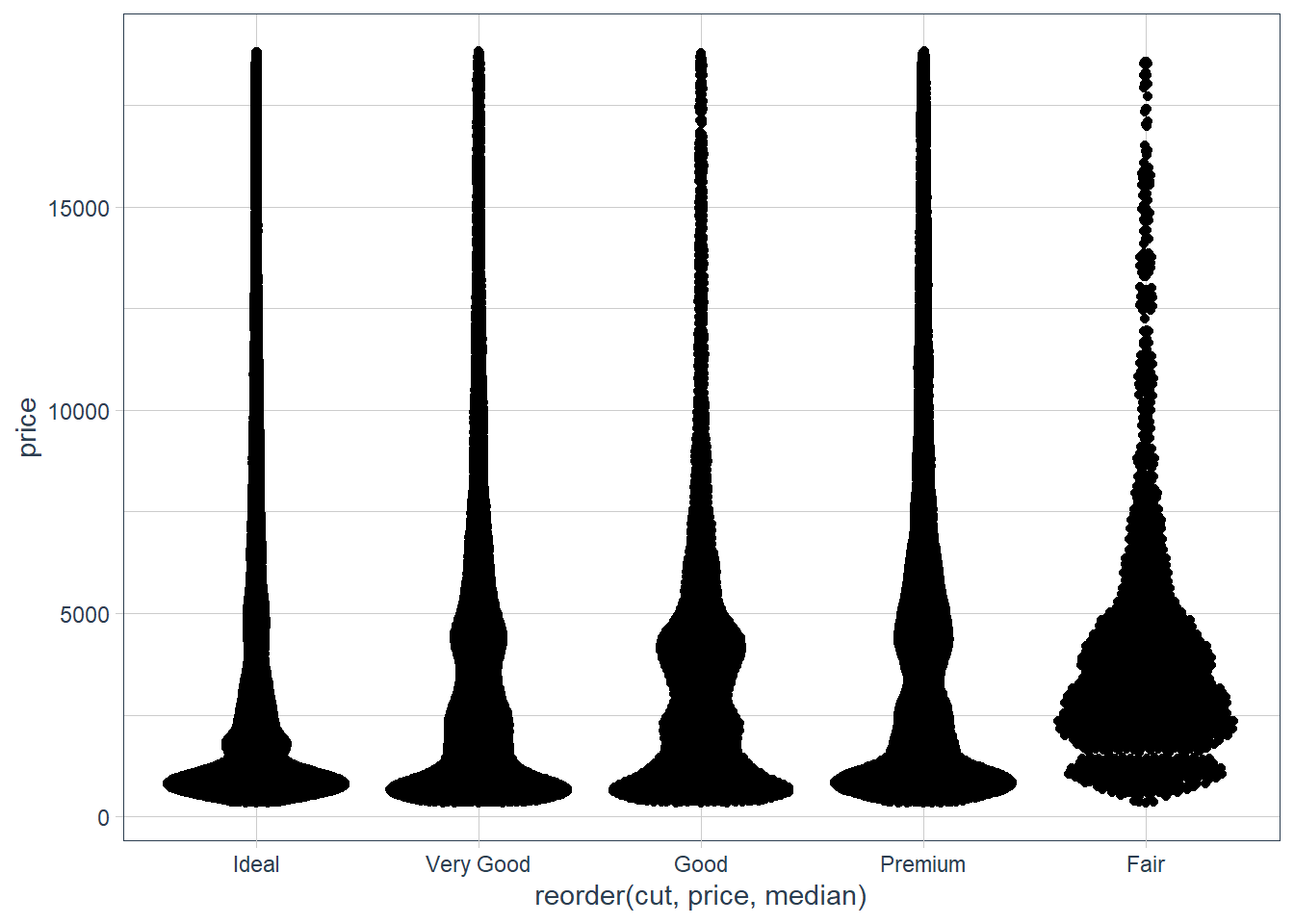The ggbeeswarm 📦 produces column scatter plots and has 2 functions, one geom_quasirandom() reduces the overplotting and also helps visualise the density at each point.

The other is geom_beeswarm() and does a point-size offset.

### Two Categorical Variables

For two catgories we need to count the number of observations in each combination of the 2 categories.

We can use geom_count() for this, and the size of each dot tells you how many observations fell in that category.

ggplot(penguins) +  geom_count(aes(x = species, y = island))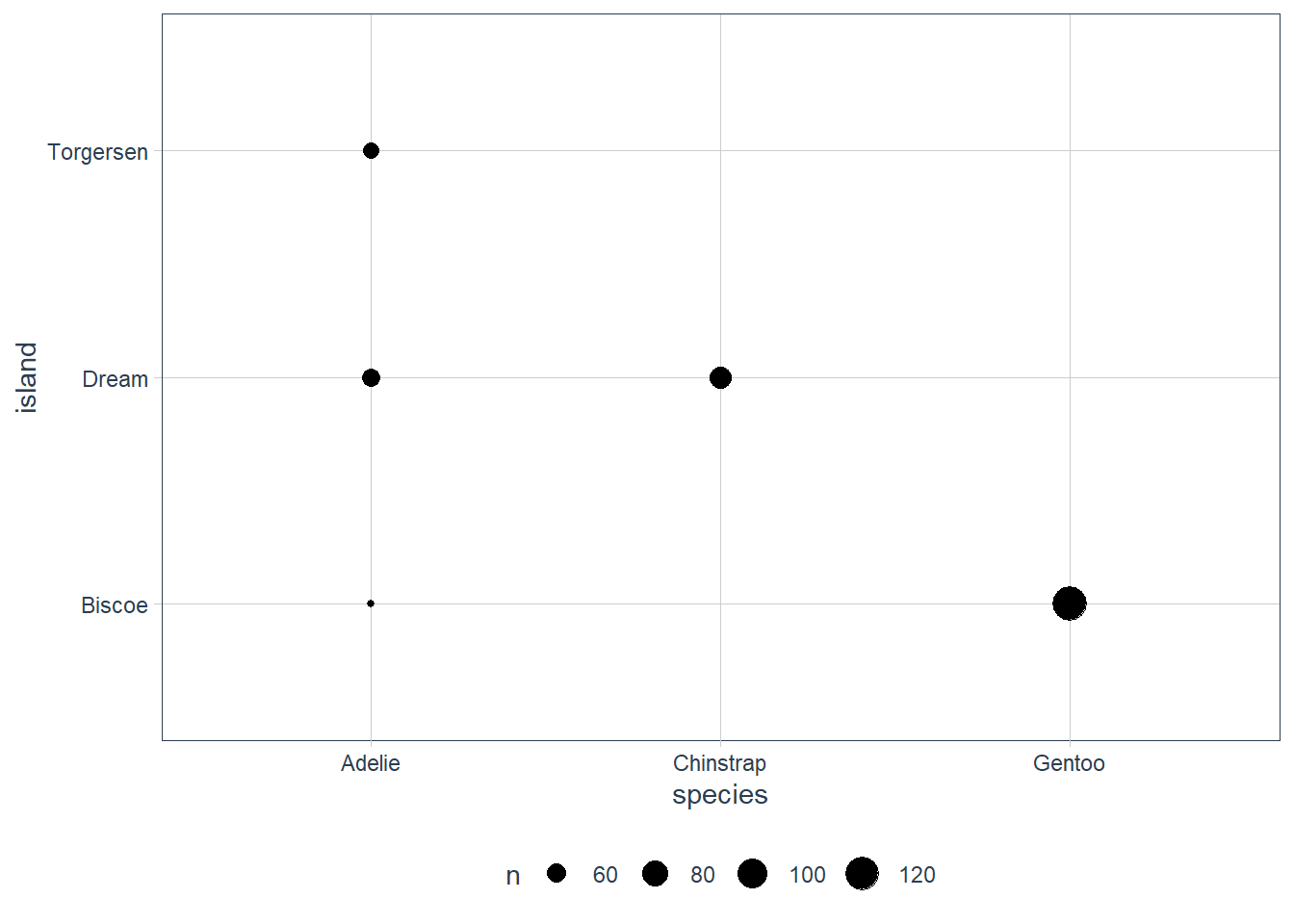penguins %>%   count(species, island, sort = TRUE)

# A tibble: 5 x 3
species   island        n
<fct>     <fct>     <int>
1 Gentoo    Biscoe      124
2 Chinstrap Dream        68

ggplot(penguins) +  geom_count(aes(x = species, y = sex))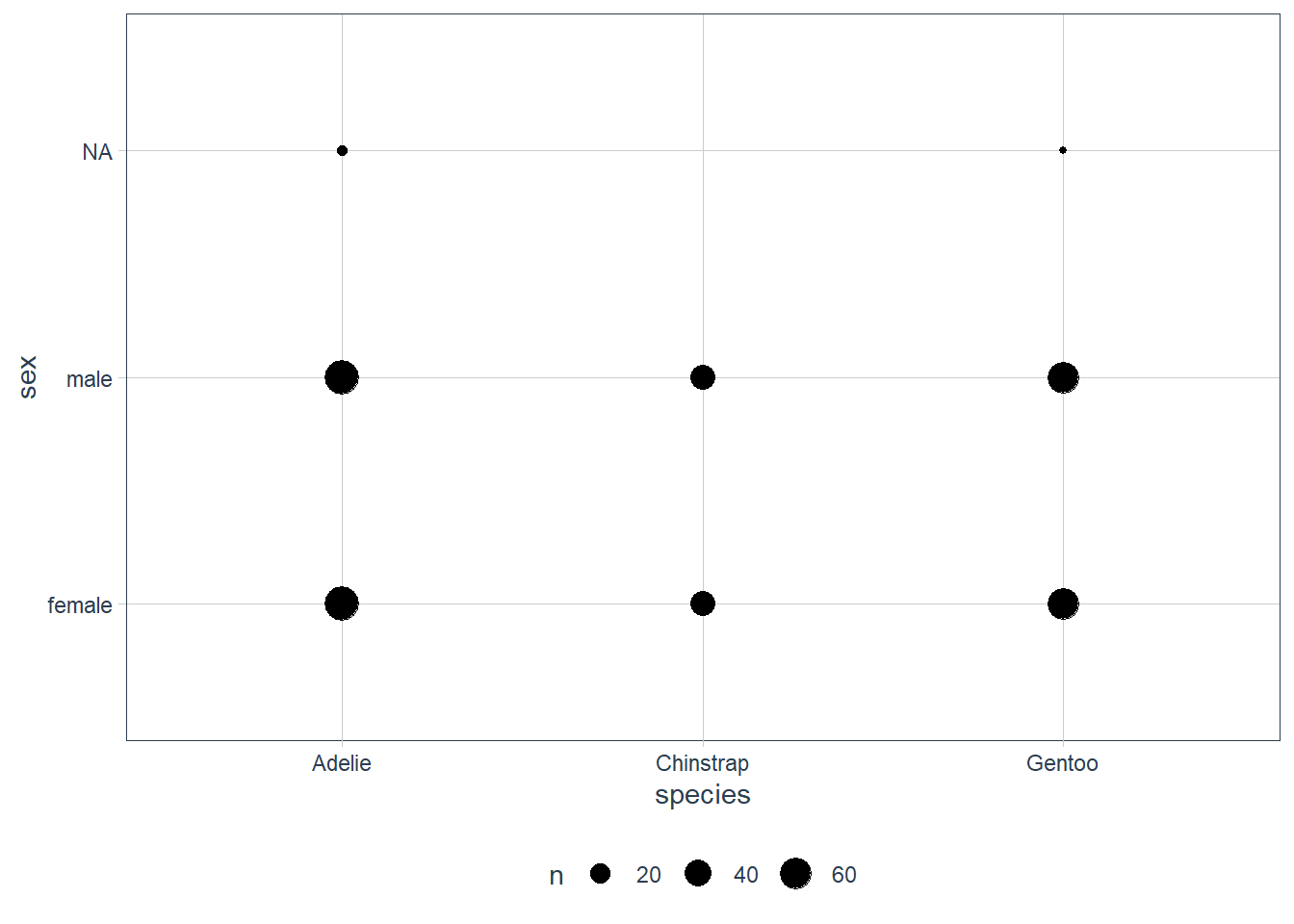penguins %>%   count(species, sex, sort = TRUE)

# A tibble: 8 x 3
species   sex        n
<fct>     <fct>  <int>
3 Gentoo    male      61
4 Gentoo    female    58
5 Chinstrap female    34
6 Chinstrap male      34
8 Gentoo    <NA>       5


We can see that the largest number of observations were of the Gentoo species, on the island Biscoe.

The male / female splits look roughly equal and is confirmed using a count().

Another way is to use the geom_tile() function but here you must first compute the count, and then use the count as a fill.

penguins %>%   count(species, island) %>%   ggplot(aes(x = species, y = island)) +  geom_tile(aes(fill = n ))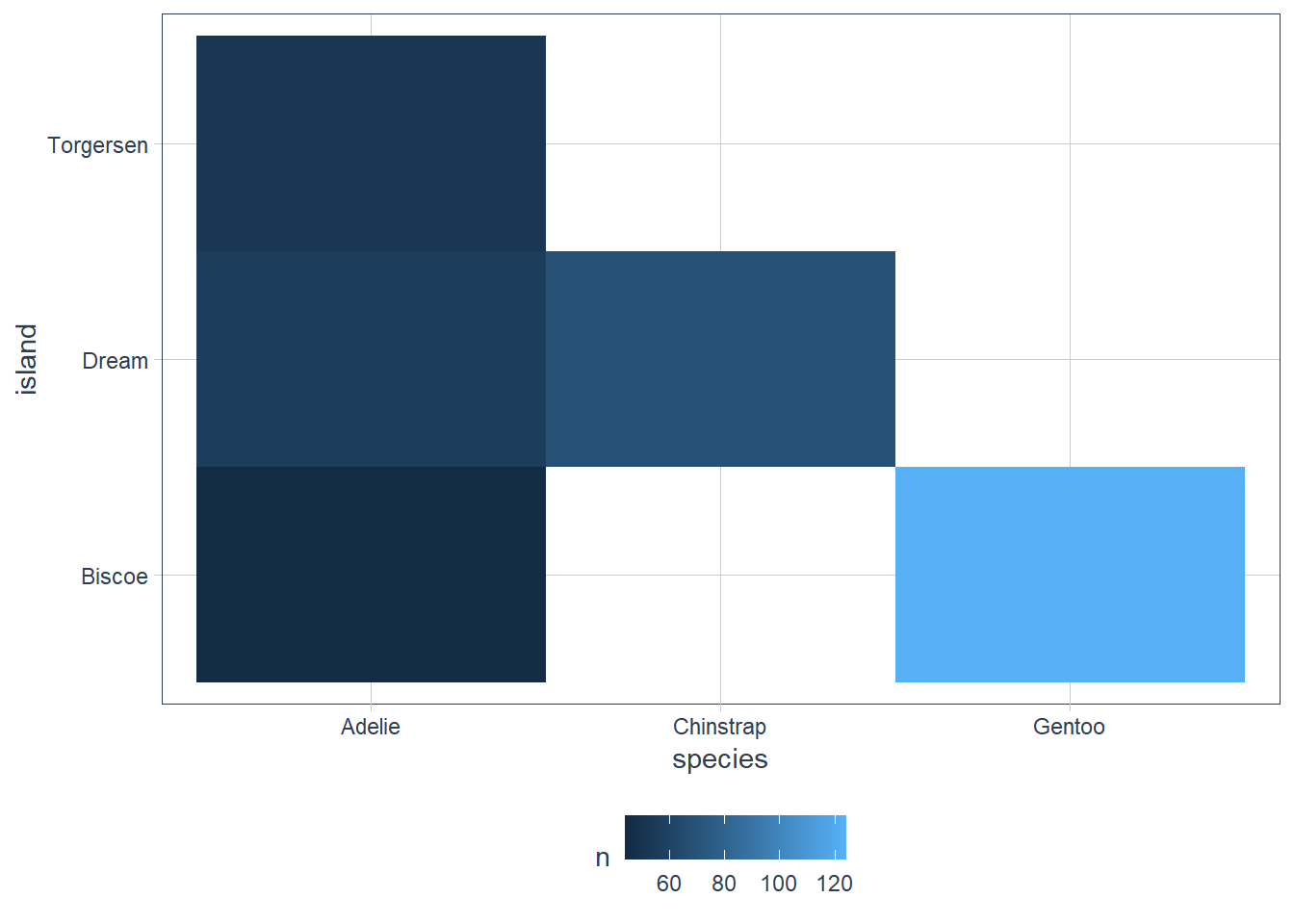penguins %>%   count(species, island, sort = TRUE)

# A tibble: 5 x 3
species   island        n
<fct>     <fct>     <int>
1 Gentoo    Biscoe      124
2 Chinstrap Dream        68

penguins %>%   count(species, sex) %>%   ggplot(aes(x = species, y = sex)) +  geom_tile(aes(fill = n ))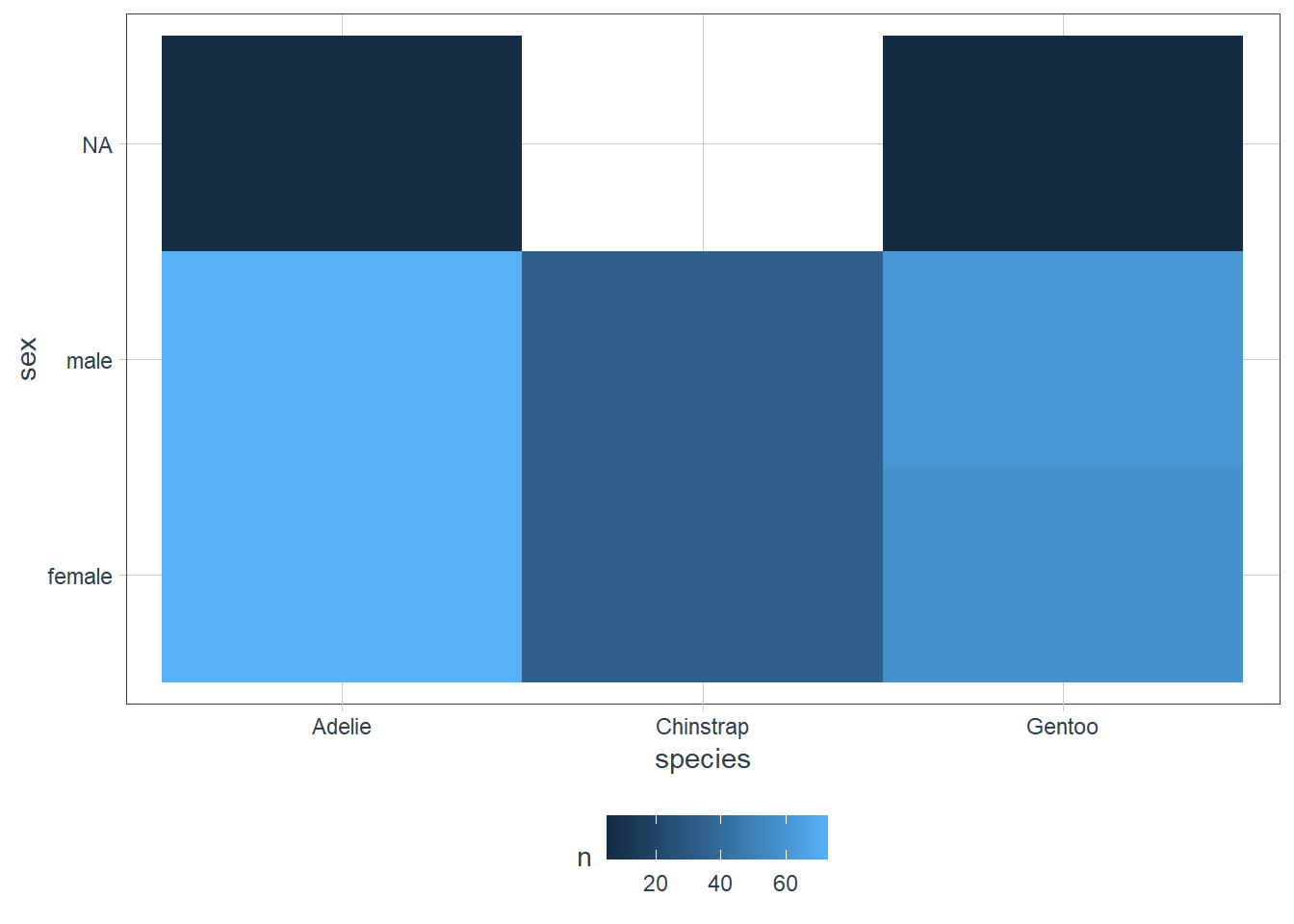penguins %>%   count(species, sex, sort = TRUE)

# A tibble: 8 x 3
species   sex        n
<fct>     <fct>  <int>
3 Gentoo    male      61
4 Gentoo    female    58
5 Chinstrap female    34
6 Chinstrap male      34
8 Gentoo    <NA>       5


We see that the colouring for the tiled graph of species and sex show again that the data is quite balanced in terms of males vs females for each species. The NA values also only appear for Adelie and Gentoo.

### Exercises

1. How could you rescale the count dataset above to more clearly show the distribution of cut within colour, or colour within cut?

Original:

diamonds %>%
count(color, cut) %>%
ggplot(mapping = aes(x = color, y = cut)) +
geom_tile(mapping = aes(fill = n))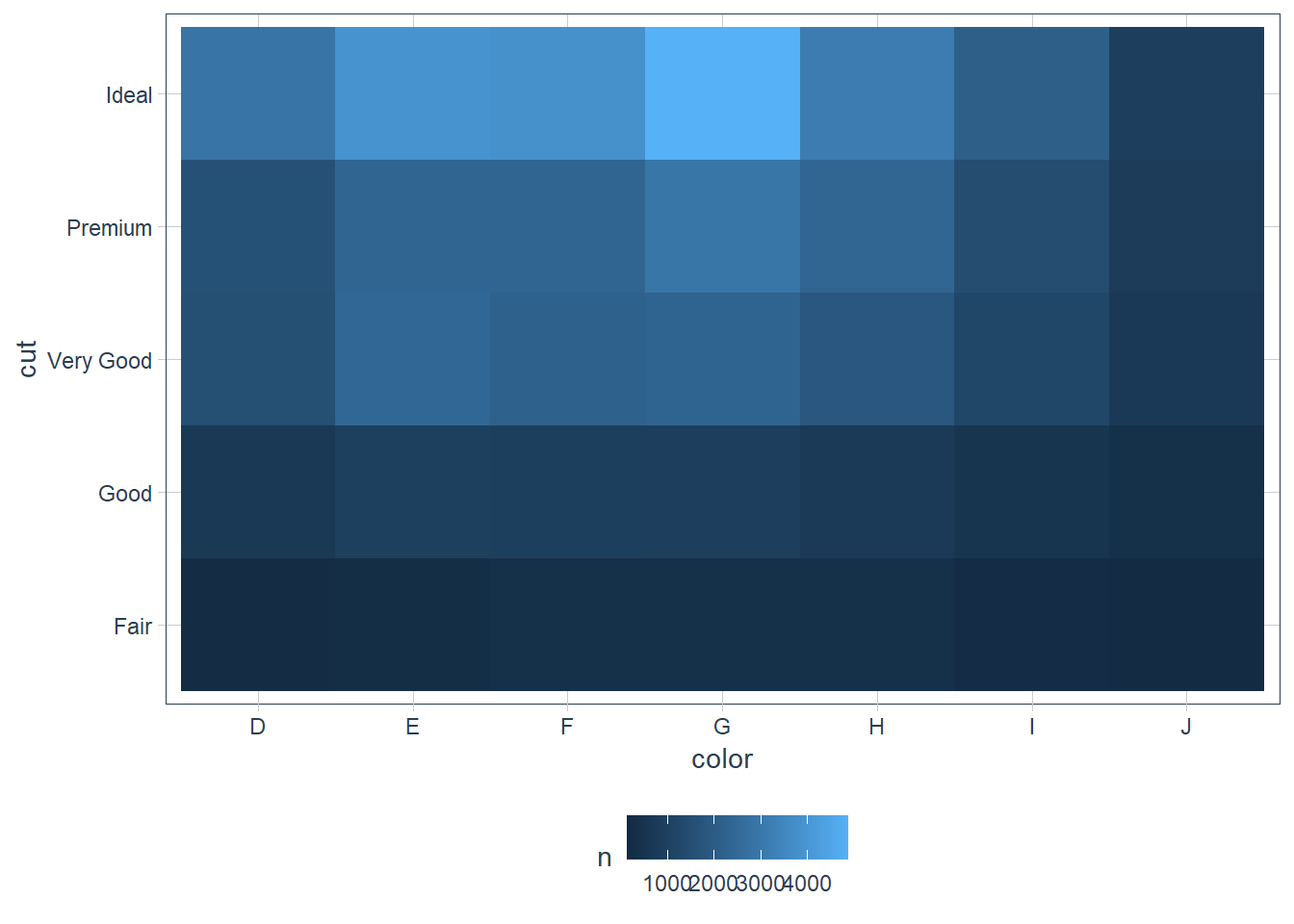diamonds %>%
group_by(color) %>%
mutate(perc = n / sum(n) * 100) %>%
ggplot(mapping = aes(x = color, y = cut)) +
geom_tile(mapping = aes(fill = perc)) +
high = "gold") +
guides(fill = guide_colourbar(barwidth = 10,
barheight = 0.5))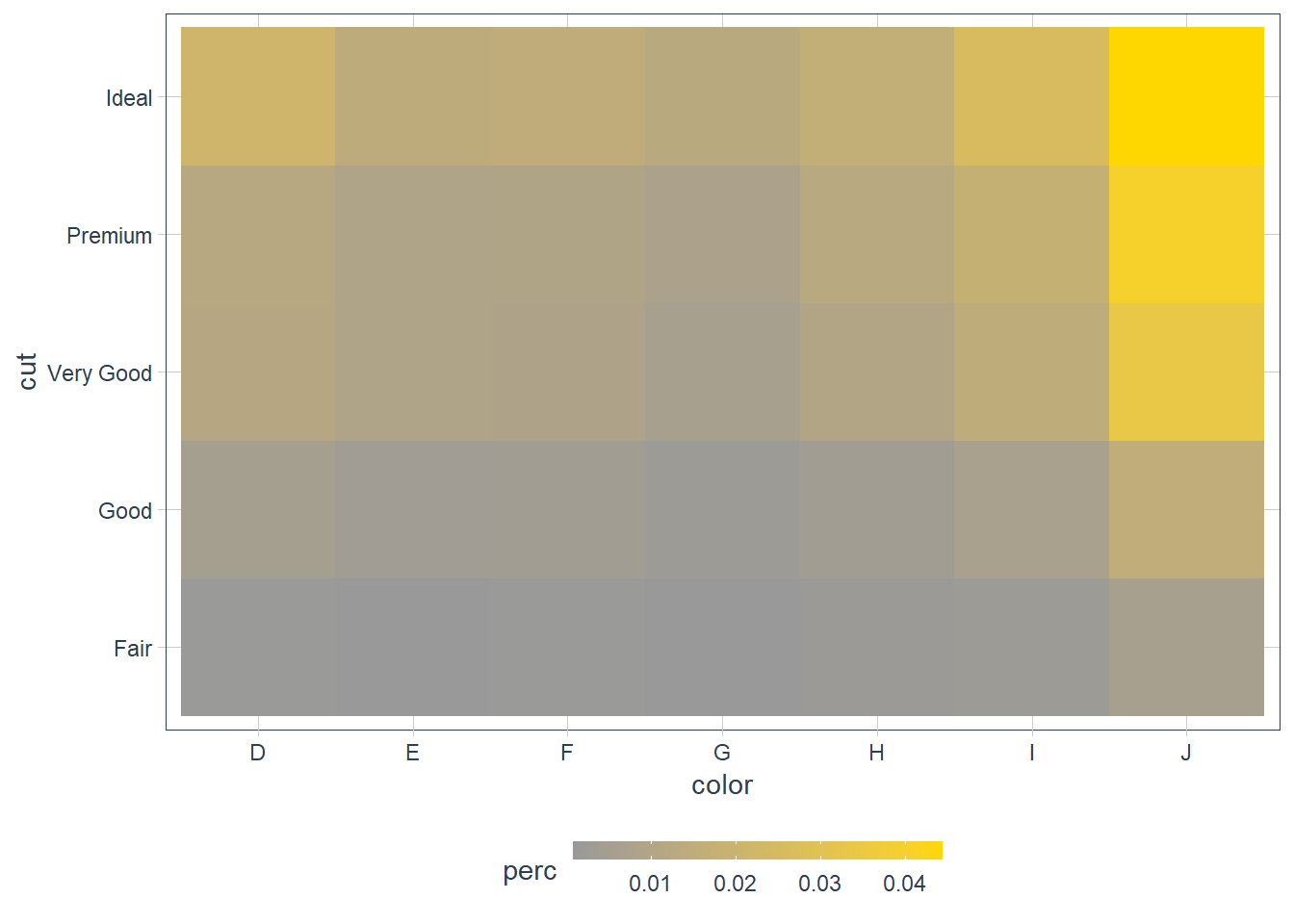diamonds %>%
group_by(cut) %>%
mutate(perc = n / sum(n) * 100) %>%
ggplot(mapping = aes(x = cut, y = color)) +
geom_tile(mapping = aes(fill = perc)) +
high = "#293352") +
guides(fill = guide_colourbar(barwidth = 10,
barheight = 0.5))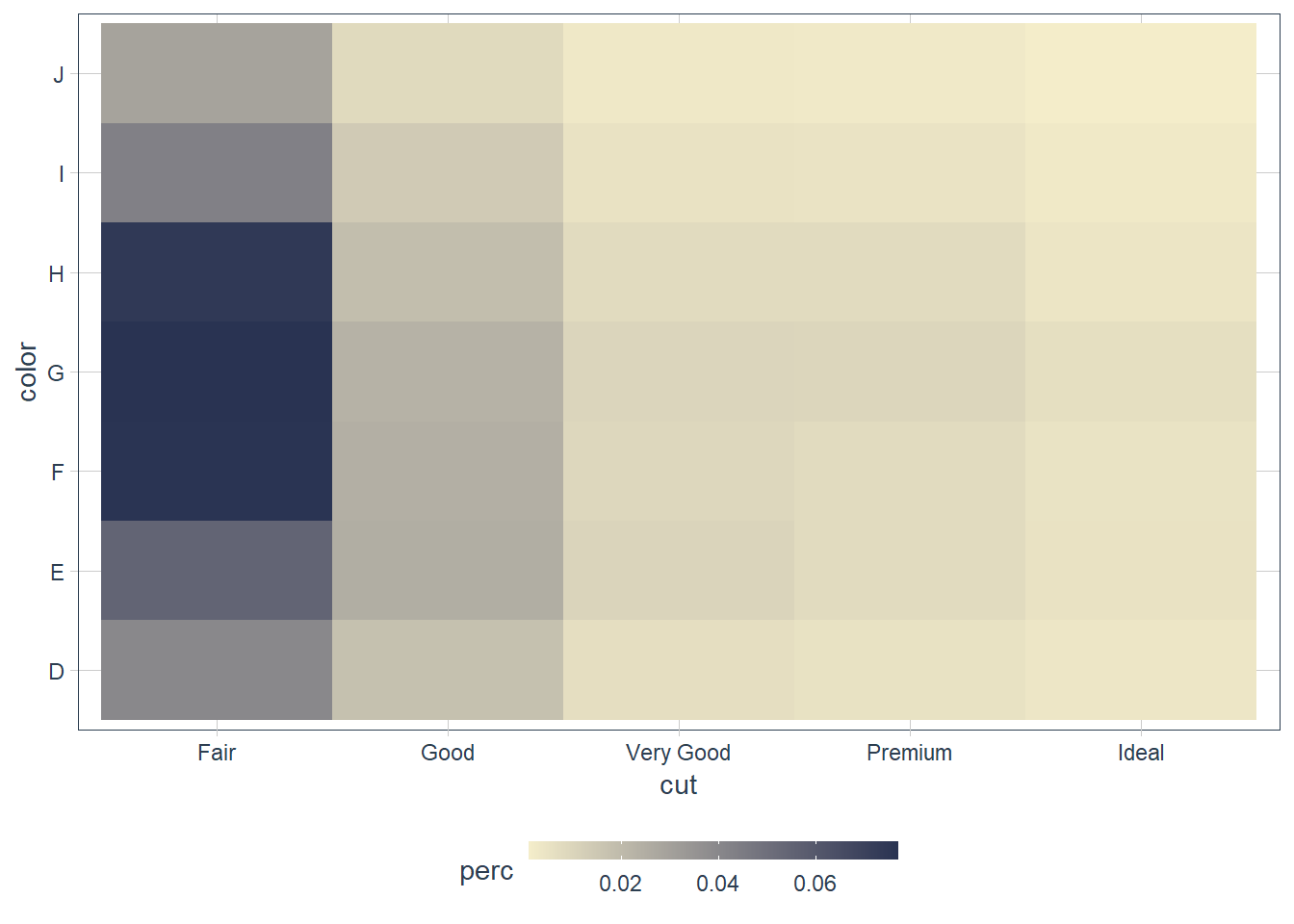Maybe use a percentage within each color.

2. Use geom_tile() together with dplyr to explore how average flight delays vary by destination and month of year. What makes the plot difficult to read? How could you improve it?

flights %>%
group_by(dest, month) %>%
mutate(avg_delay = mean(arr_delay, na.rm = TRUE)) %>%
ggplot(aes(x = month, y = dest)) +
geom_tile(mapping = aes(fill = avg_delay)) +
high = "#293352") +
guides(fill = guide_colourbar(barwidth = 10,
barheight = 0.5))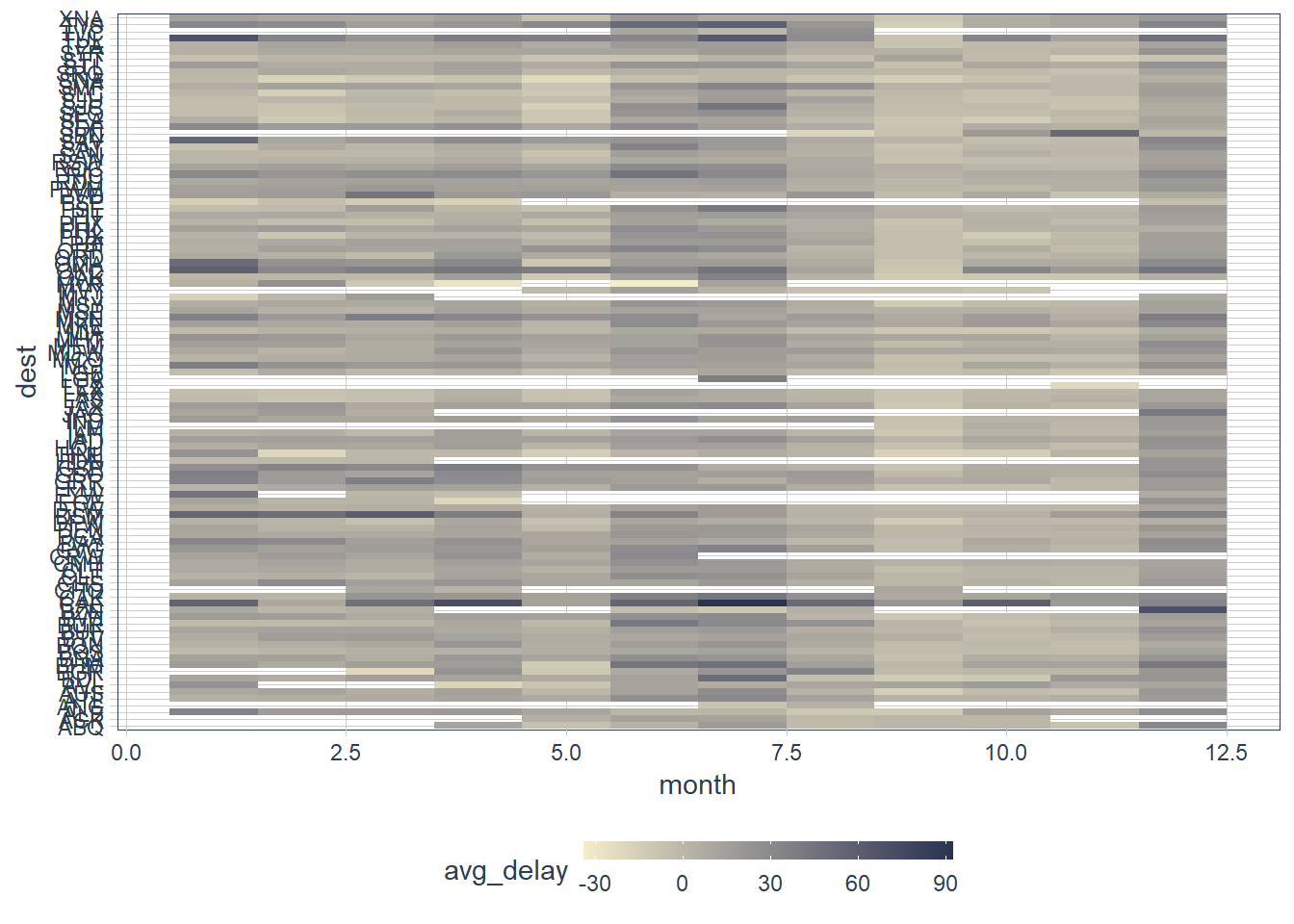flights %>%
mutate(dest = fct_lump(dest, 10)) %>%
group_by(dest, month) %>%
mutate(avg_delay = mean(arr_delay, na.rm = TRUE)) %>%
ggplot(aes(x = month, y = dest)) +
geom_tile(mapping = aes(fill = avg_delay)) +
high = "#293352") +
guides(fill = guide_colourbar(barwidth = 10,
barheight = 0.5))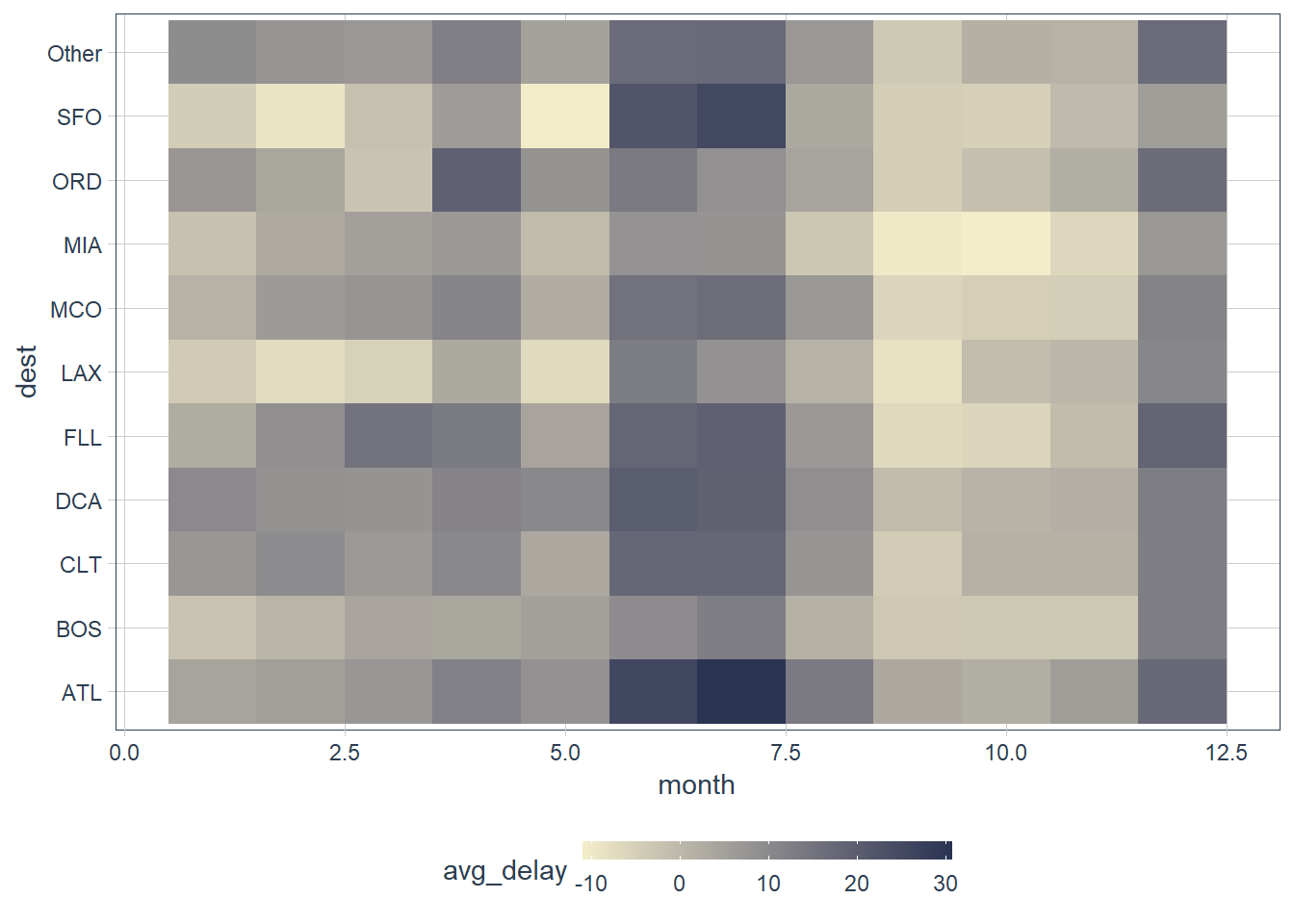The missing values for some months is an issue. There are many destinations. It will be better to sort it by the highest number of flights and highest average delay, and then maybe also lump the lower ones into a single category with something like fct_lump() which we will learn about later. For now just see fct_lump(var, n = 8) as lumping together factors that are less frequent. Here we keep the most frequent or top 8 of the variable var. In the example above we kept the 10 most frequent destinations, and lumped the rest into Other.

3. Why is it slightly better to use aes(x = color, y = cut) rather than aes(x = cut, y = color) in the example above?

diamonds %>%
count(color, cut) %>%
ggplot(mapping = aes(x = color, y = cut)) +
geom_tile(mapping = aes(fill = n)) +
high = "#293352") +
guides(fill = guide_colourbar(barwidth = 10,
barheight = 0.5))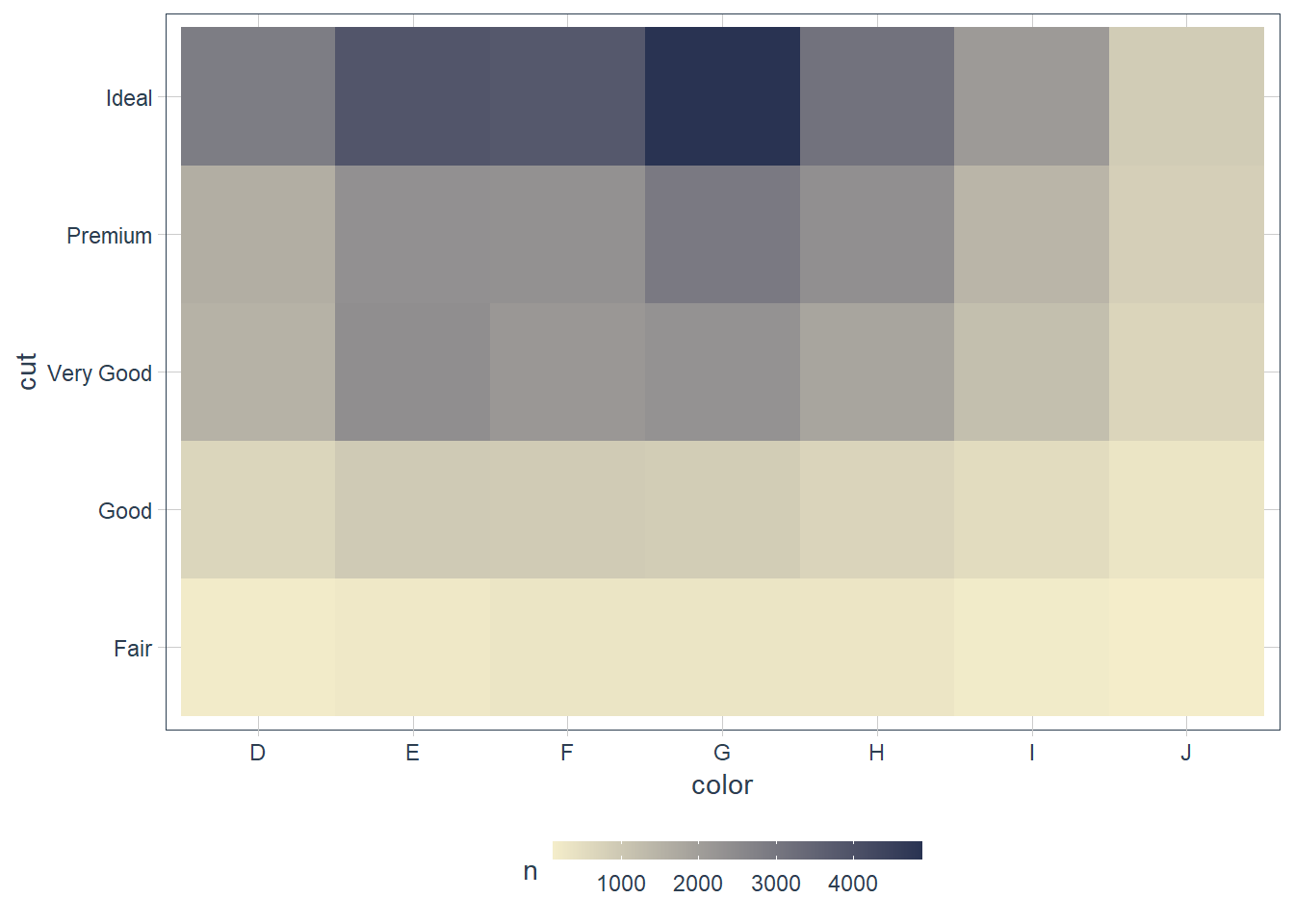diamonds %>%
count(cut, color) %>%
ggplot(mapping = aes(x = cut, y = color)) +
geom_tile(mapping = aes(fill = n)) +
high = "#293352") +
guides(fill = guide_colourbar(barwidth = 10,
barheight = 0.5))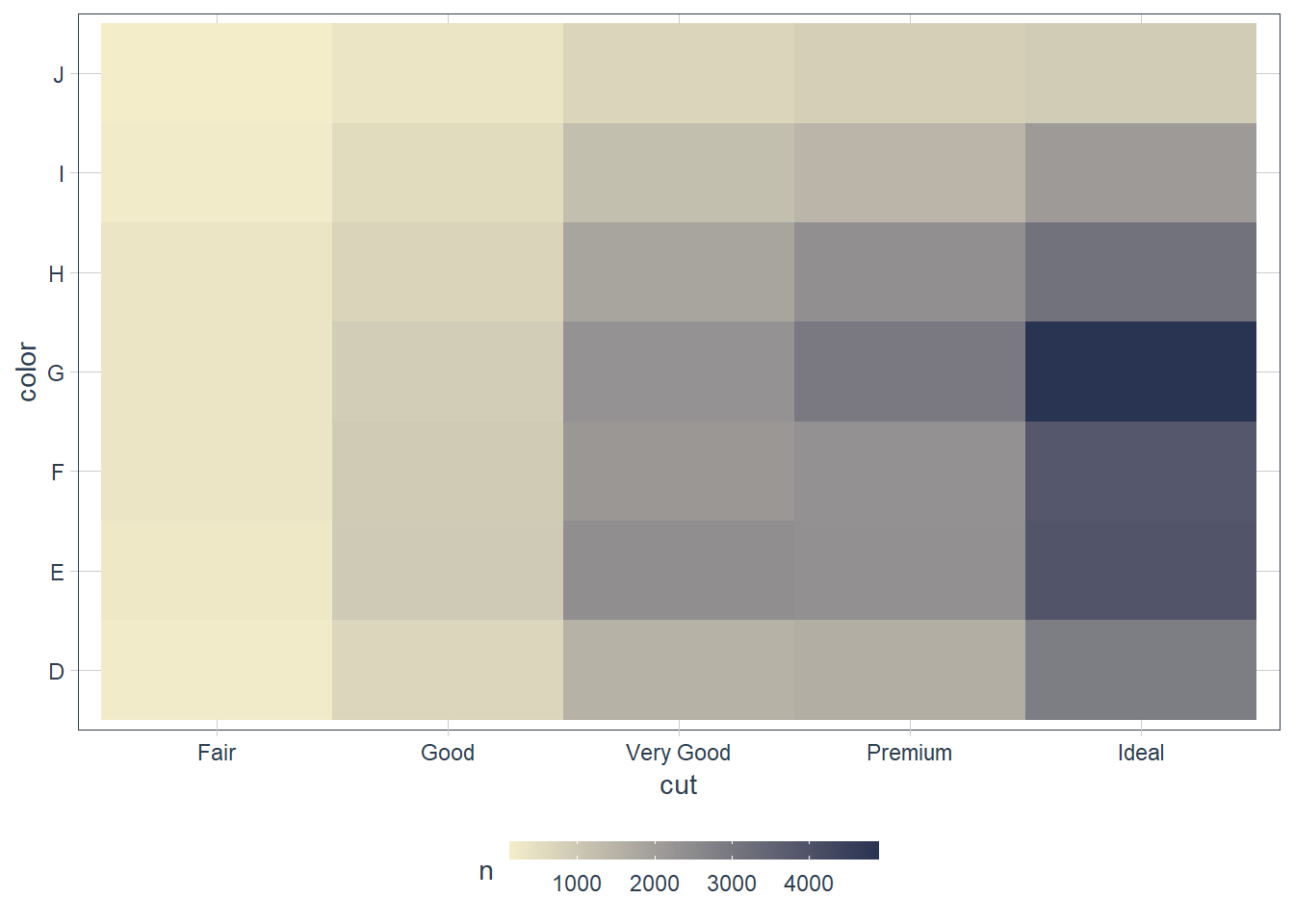For me it is easier to read. I can say in the color D there is approximately 200 observations of the cut Fair, ~ 500 observations of the cut Good etc. I.e. there is more variation in the cut within each color than the other way around if that makes sense.

If I read the color in each cut, there’s not much variation, e.g. for the Fair cut ~200 lie in every color.

### Two Continuous Variables

One way we have already seen is the geom_point(). When you have lots of data you can use an alpha value to prevent the overplotting, and see you data more clearly.

ggplot(penguins) +  geom_point(aes(x = bill_length_mm,                 y = bill_depth_mm))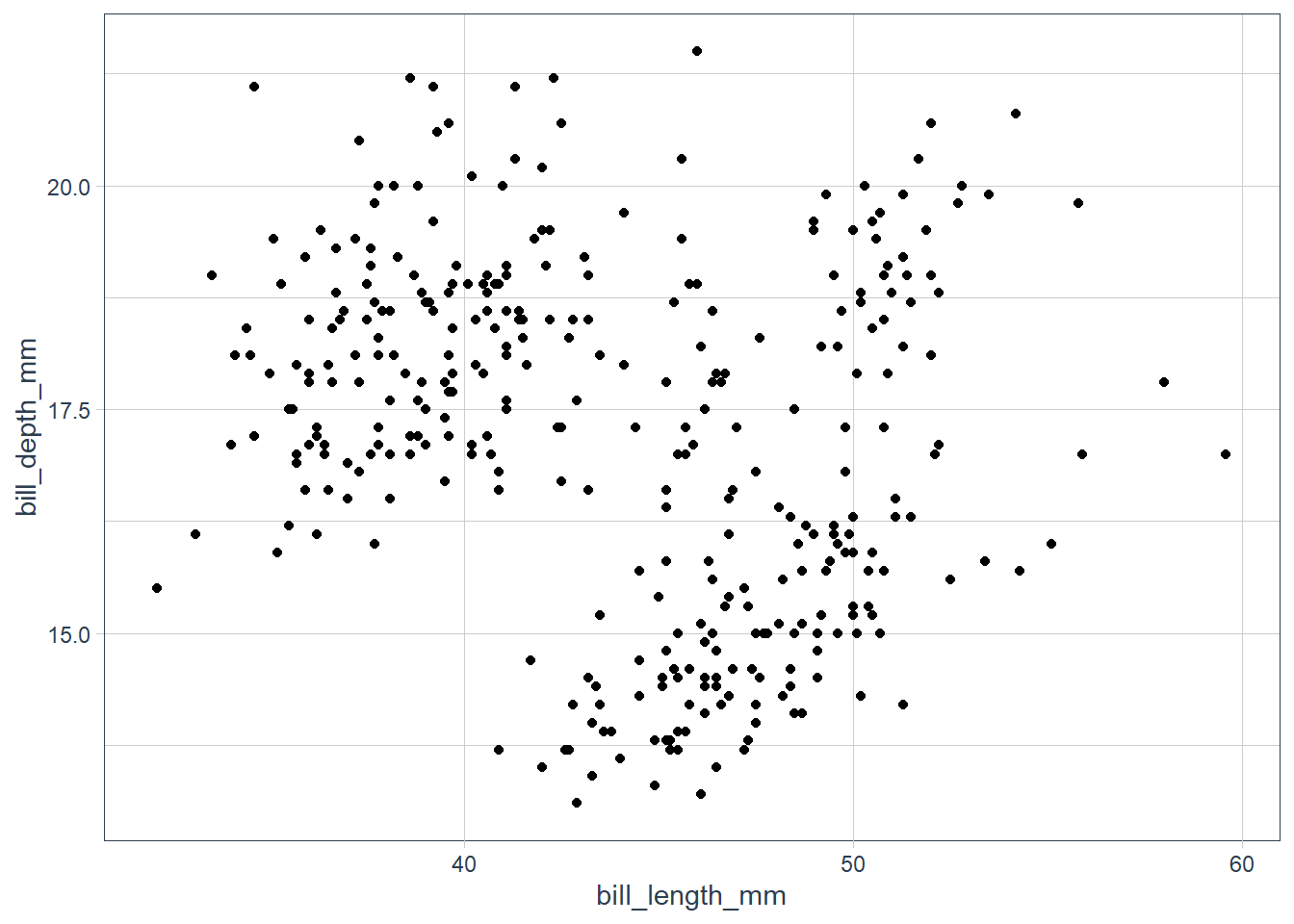ggplot(penguins) +  geom_point(aes(x = bill_length_mm,                 y = bill_depth_mm,                 colour = species))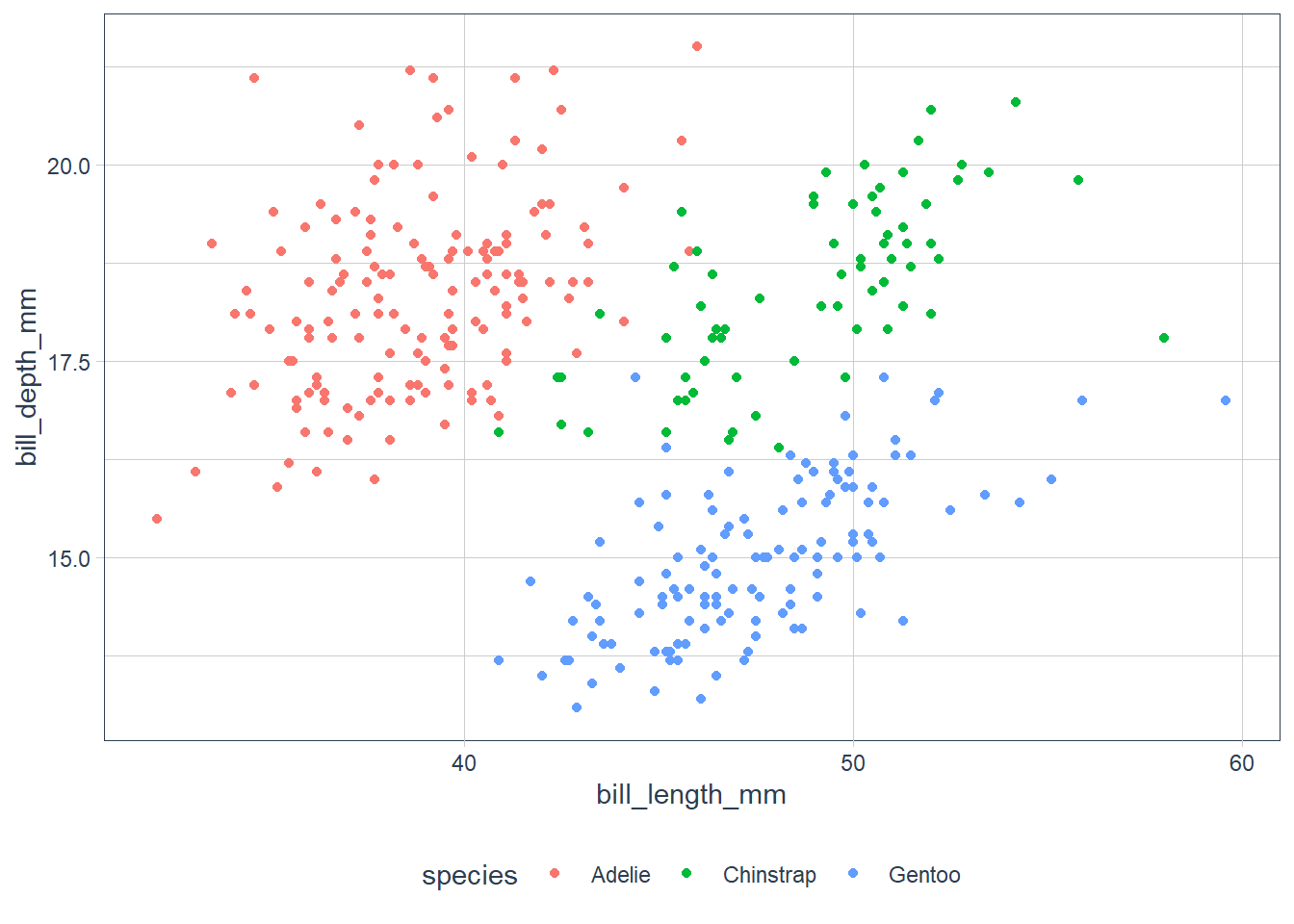It seems there is an upward trend but it is hard to make it out.

ggplot(penguins) +  geom_point(aes(x = bill_length_mm,                 y = bill_depth_mm,                 colour = species))Ah that’s much better, when the data is segmented by species we can see a trend.

With large datasets you can also bin your data and use geom_bin2d() and geom_hex().

Or alternately you can bin one variable to act as though it is categorical e.g. using cut_width(), then we can use one of the visualisations suitable for a categorical and continuous combination.

#### Exercises

1. Instead of summarising the conditional distribution with a boxplot, you could use a frequency polygon. What do you need to consider when using cut_width() vs cut_number()? How does that impact a visualisation of the 2d distribution of carat and price?

smaller <- diamonds %>%
filter(carat < 3)

ggplot(data = smaller, mapping = aes(colour =
cut_width(carat,
0.4),
x = price)) +
geom_freqpoly()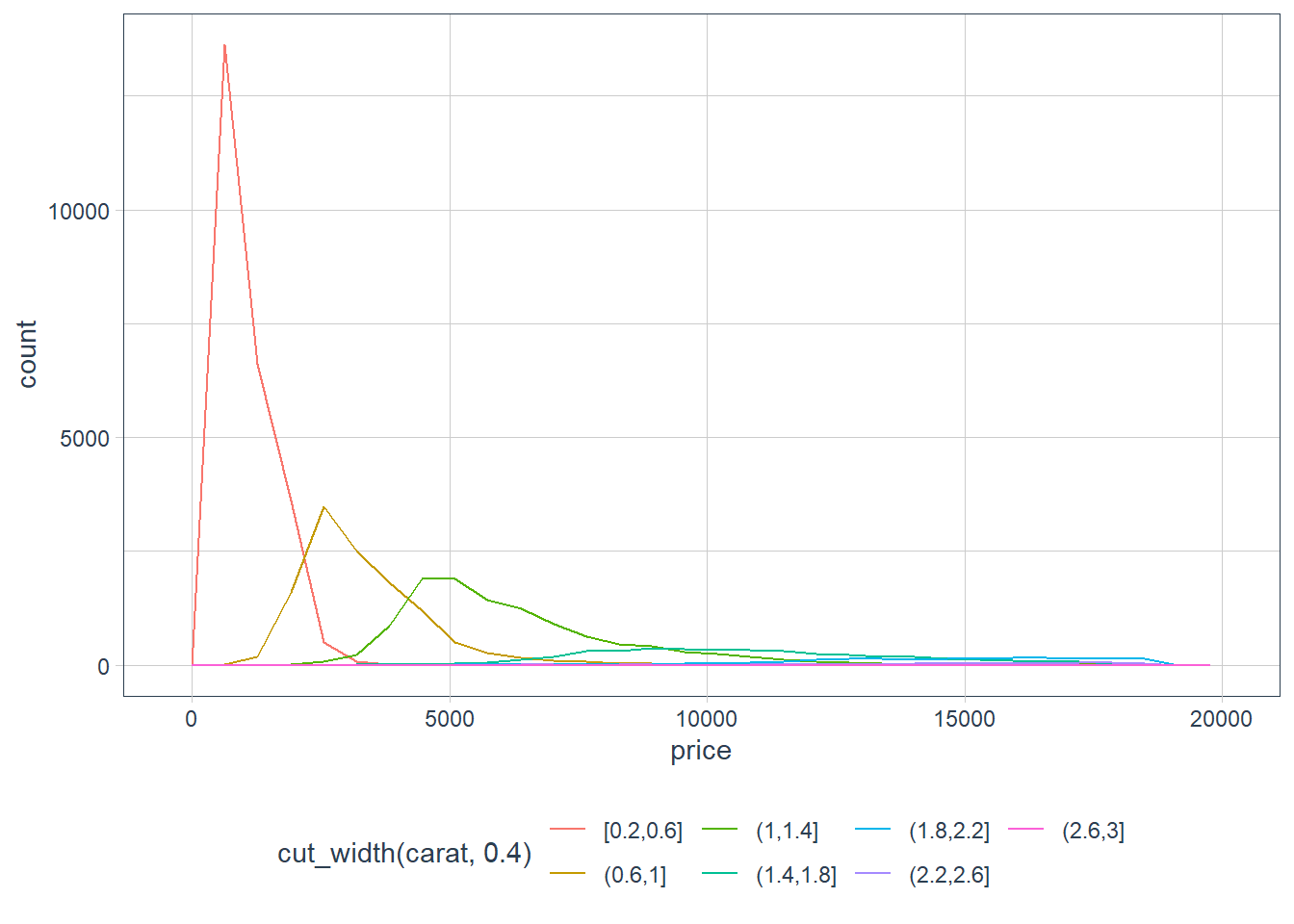ggplot(data = smaller, mapping = aes(colour =
cut_number(carat,
6),
x = price)) +
geom_freqpoly()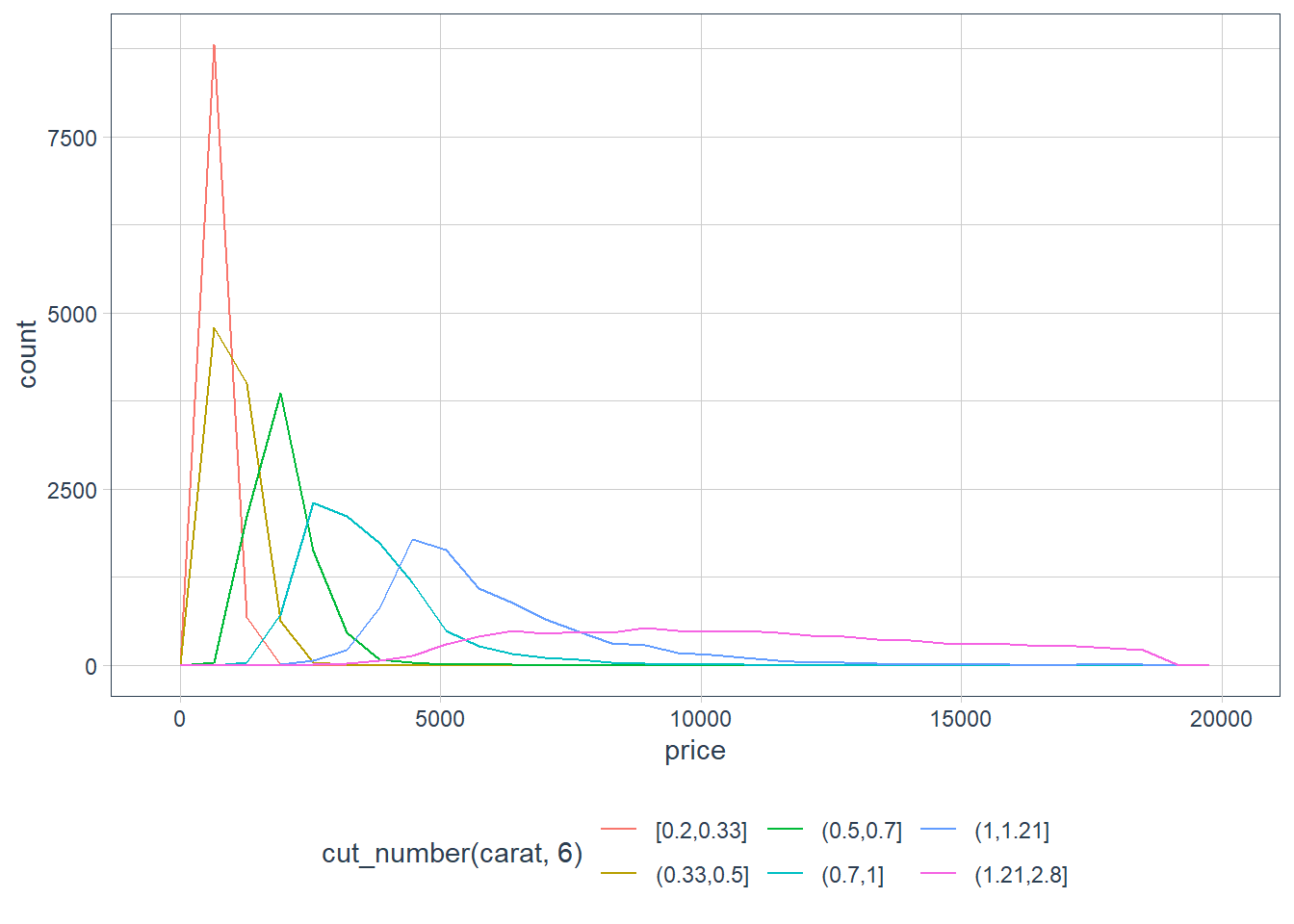ggplot(data = diamonds, mapping = aes(colour =
cut_width(carat,
0.8),
x = price)) +
geom_freqpoly()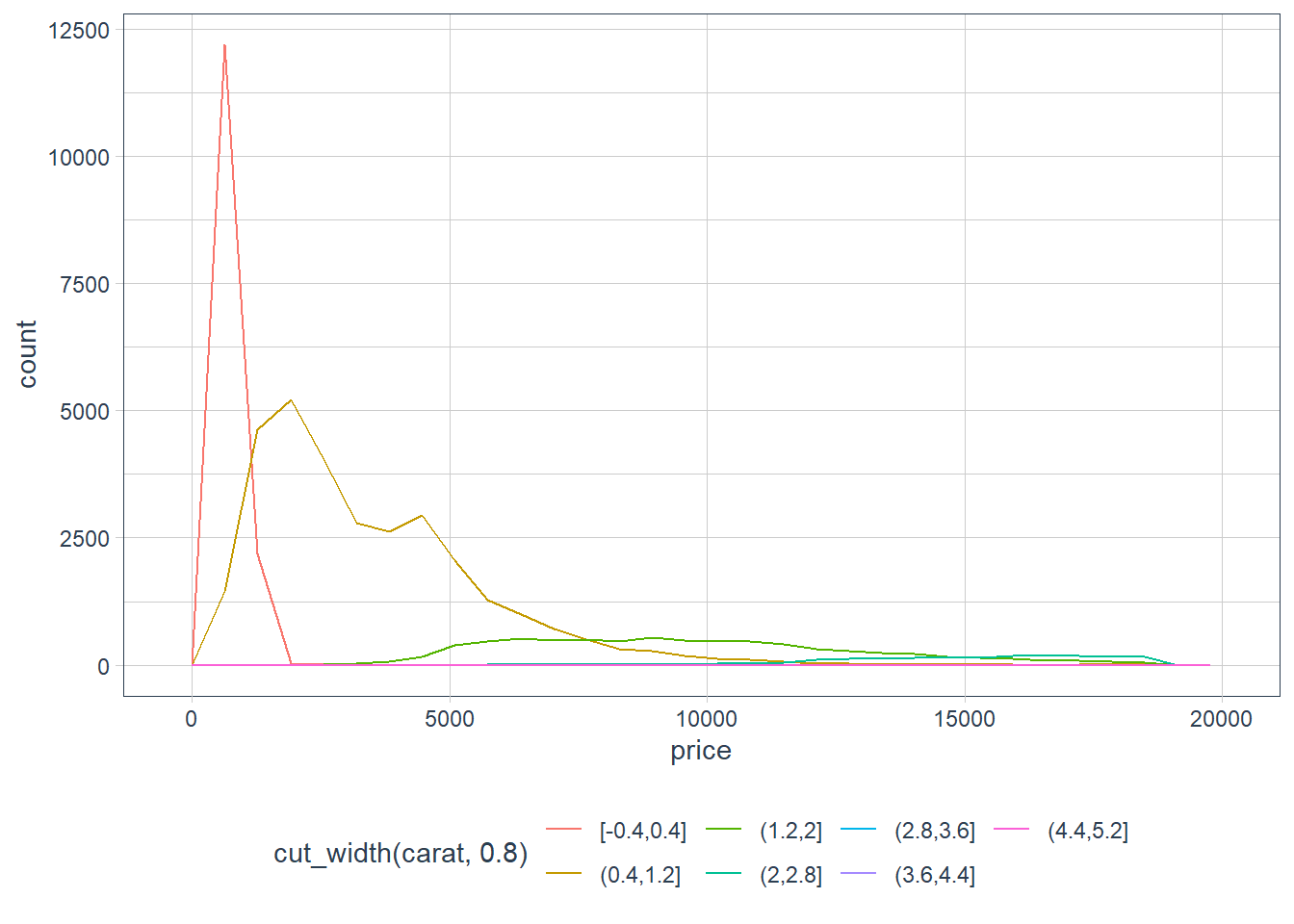ggplot(data = diamonds, mapping = aes(colour =
cut_number(carat,
6),
x = price)) +
geom_freqpoly()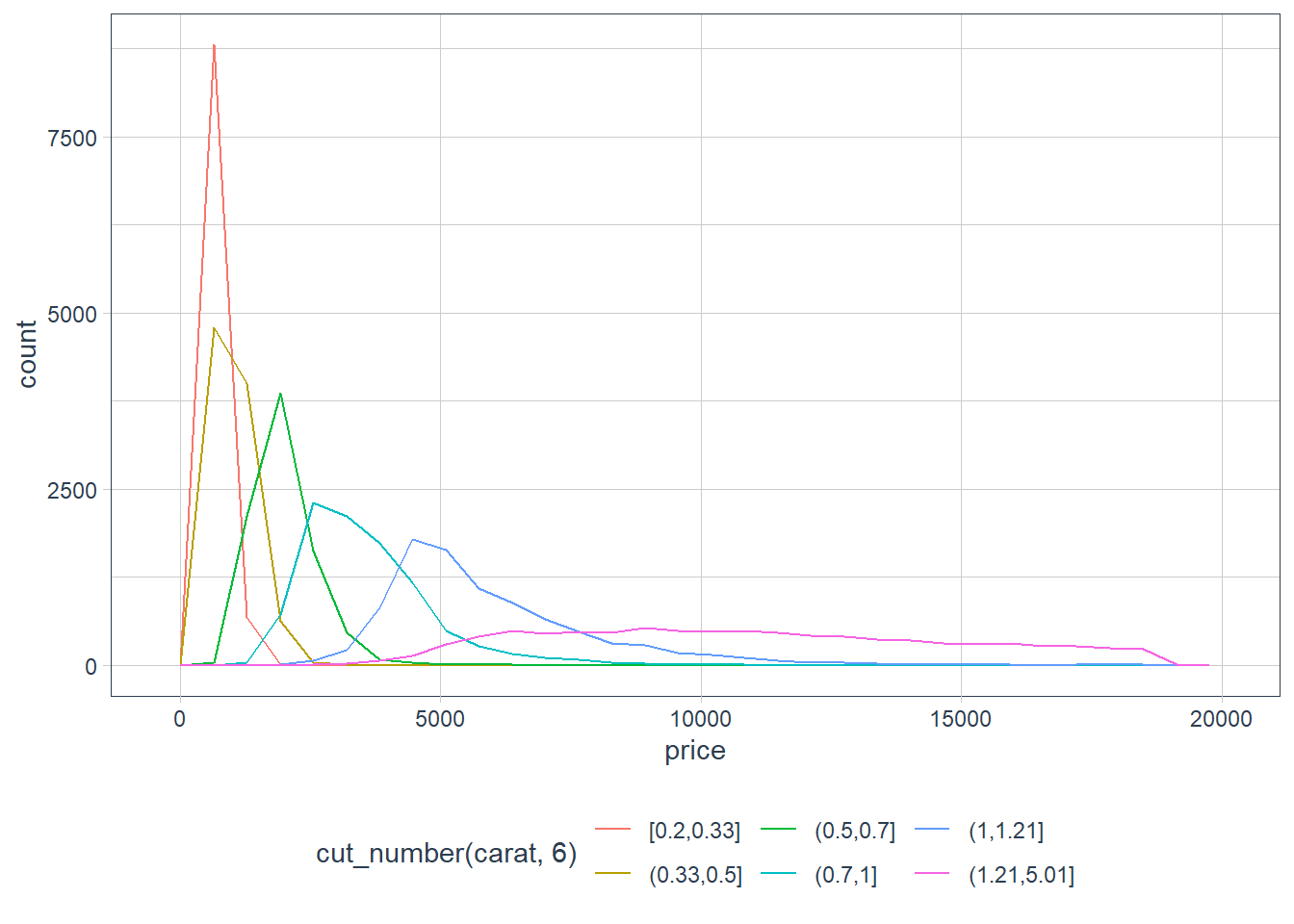cut_width() we say what we’d like the binwidth of the variable to be, and the number of bins will be calculated. cut_number() we say what we’d like as a bin number, and the binwidth of each bin is calculated.

2. Visualise the distribution of carat, partitioned by price.

ggplot(diamonds,
aes(x = carat, y = price)) +
geom_boxplot(aes(group = cut_number(price, 10)))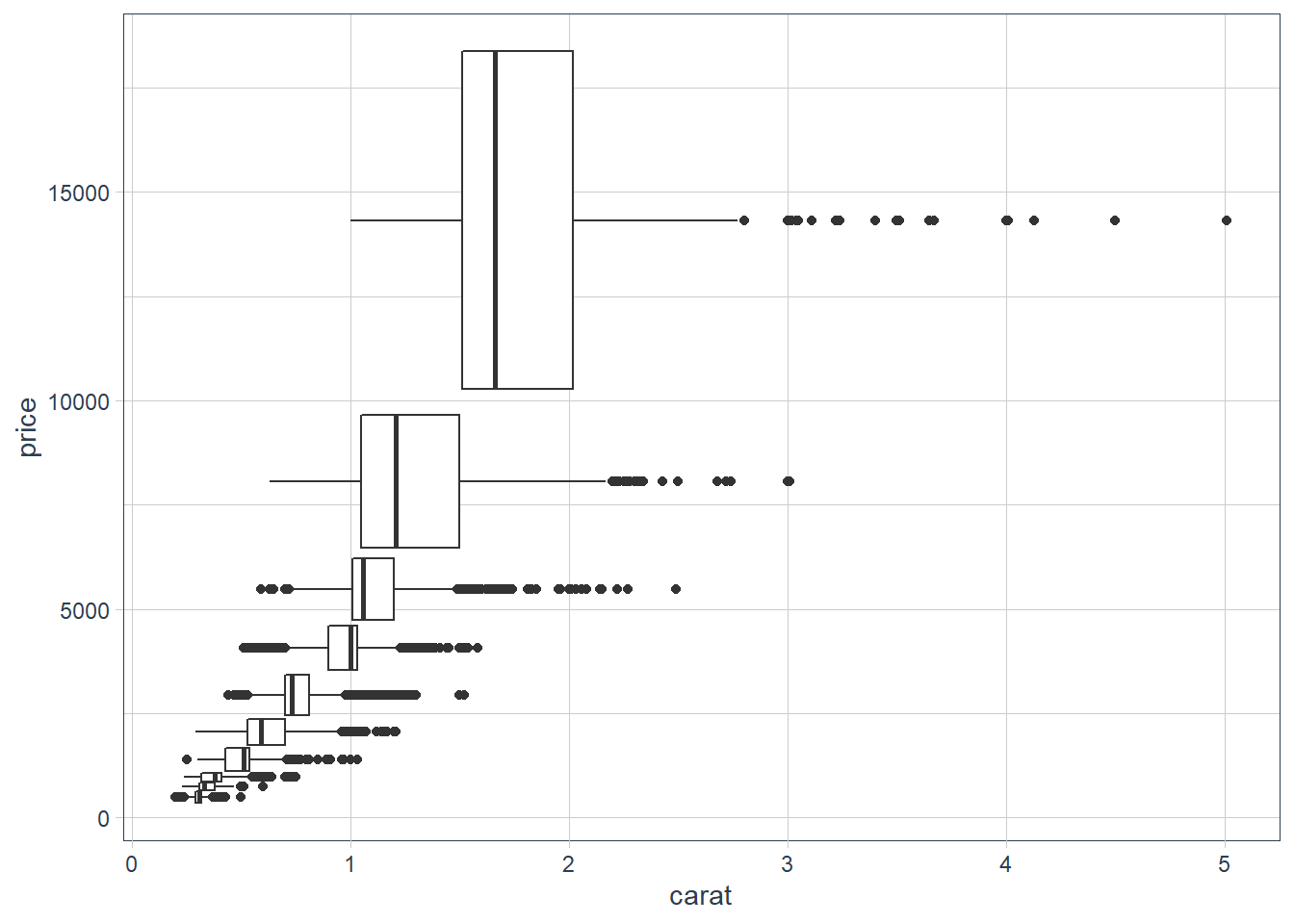3. How does the price distribution of very large diamonds compare to small diamonds? Is it as you expect, or does it surprise you?

Heavier diamonds cost more than lighter diamonds. This does not surprise me, but as you see diamonds in the range 3-5 all get a big price tag, so there is more to the pricing mechanism than just carat weight and I would guess this is where the 4C’s come in. Heavier diamonds attract a big price tag.

4. Combine two of the techniques you’ve learned to visualise the combined distribution of cut, carat, and price.

ggplot(diamonds, aes(carat, price)) +
geom_boxplot(aes(group = cut_number(price, 10))) +
facet_wrap(~ cut, scales = "free_y")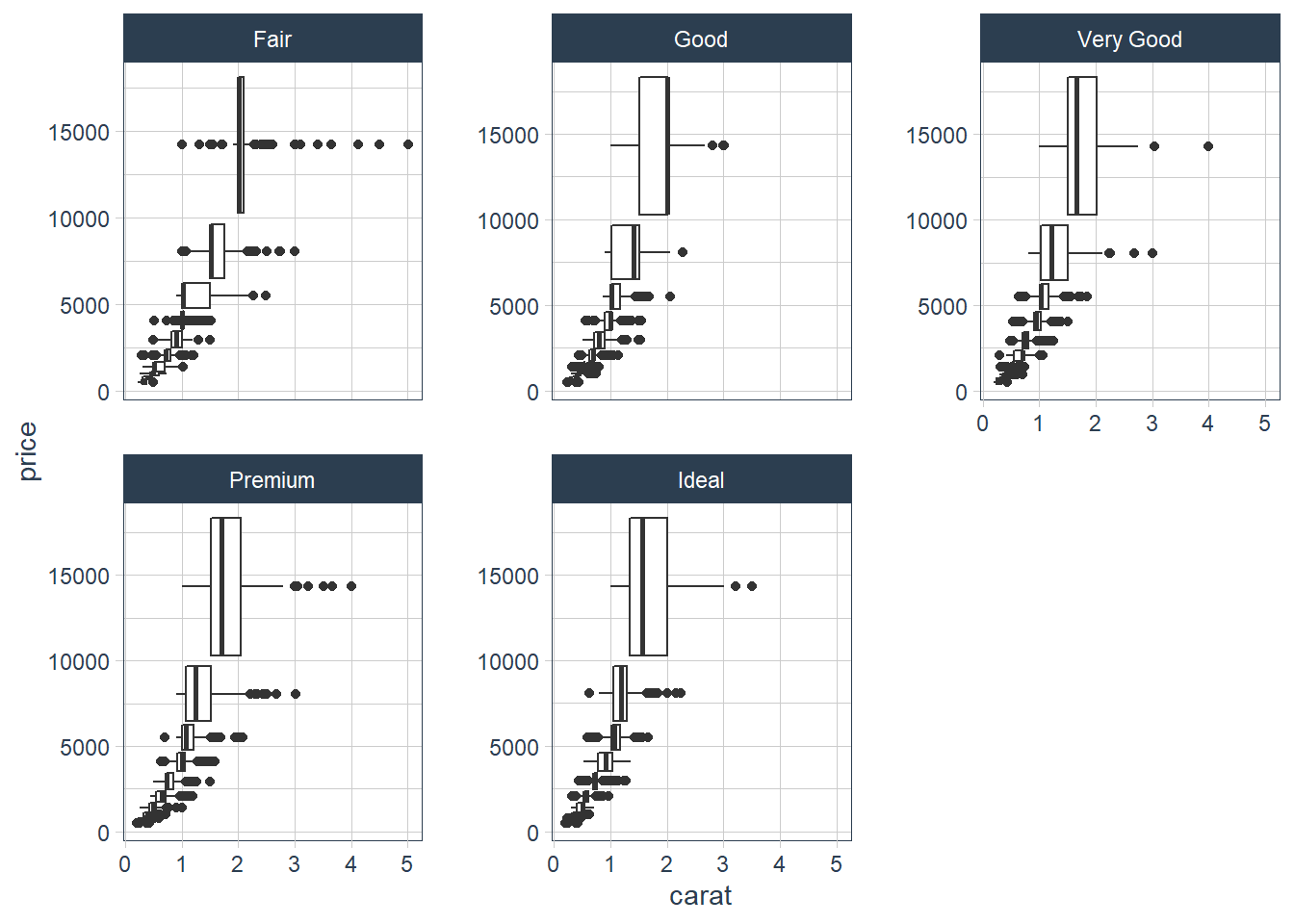5. Two dimensional plots reveal outliers that are not visible in one dimensional plots. For example, some points in the plot below have an unusual combination of x and y values, which makes the points outliers even though their x and y values appear normal when examined separately.

ggplot(data = diamonds) +
geom_point(mapping = aes(x = x, y = y))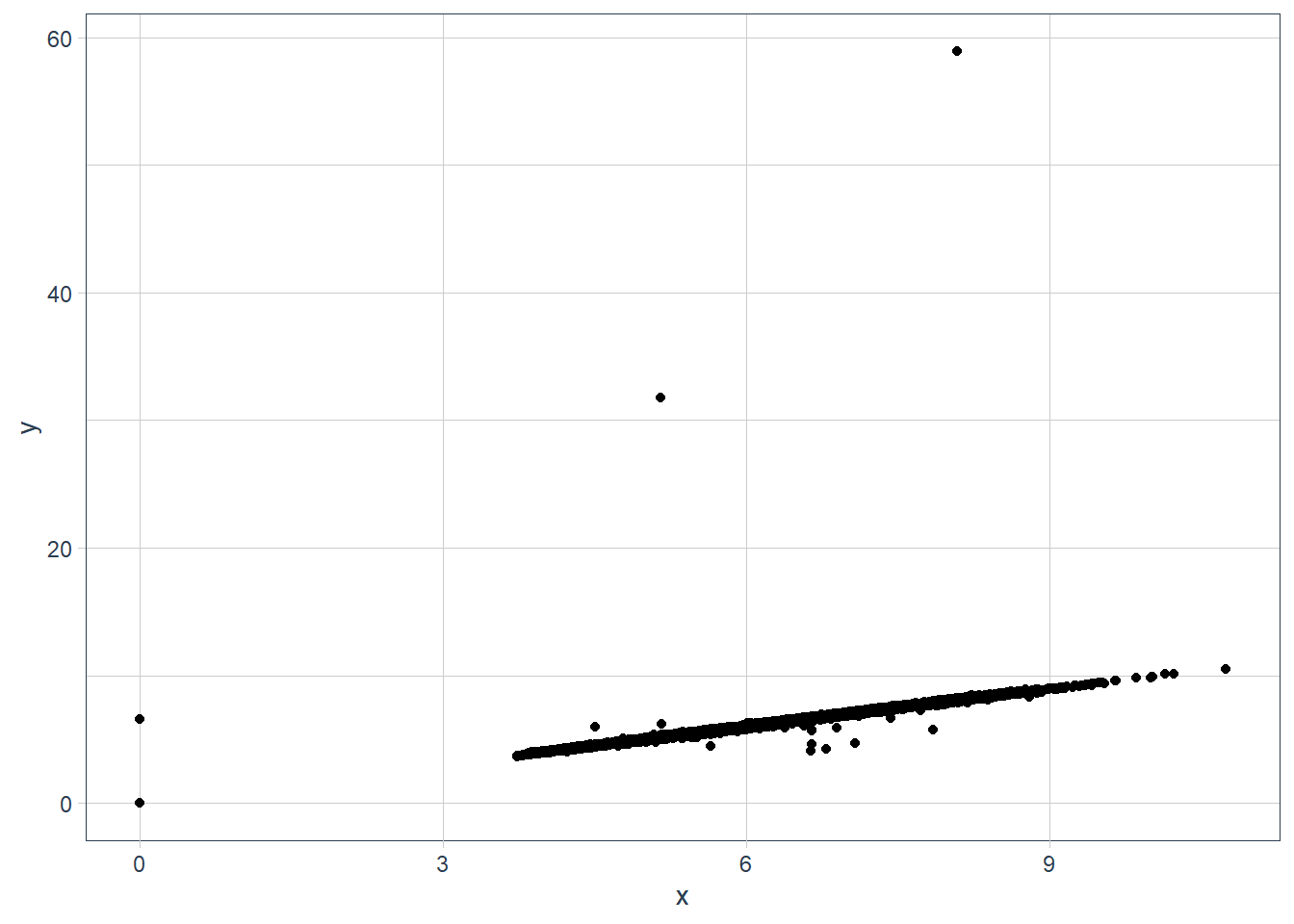ggplot(data = diamonds) +
geom_point(mapping = aes(x = x, y = y)) +
coord_cartesian(xlim = c(4, 11), ylim = c(4, 11))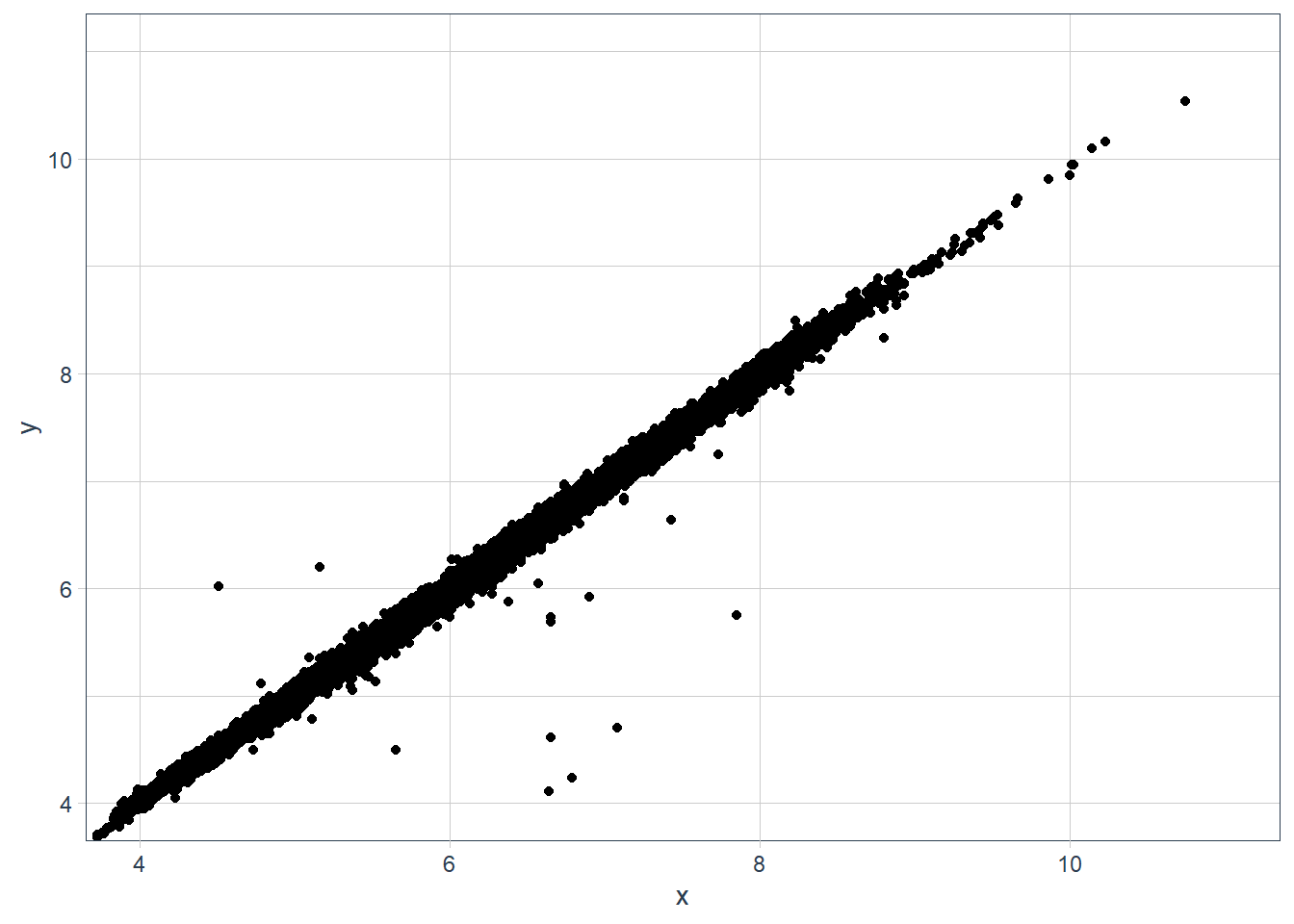ggplot(data = diamonds) +
geom_boxplot(mapping = aes(x = x, y = y, group=cut_number(y, 10))) +
coord_cartesian(xlim = c(4, 11), ylim = c(4, 11))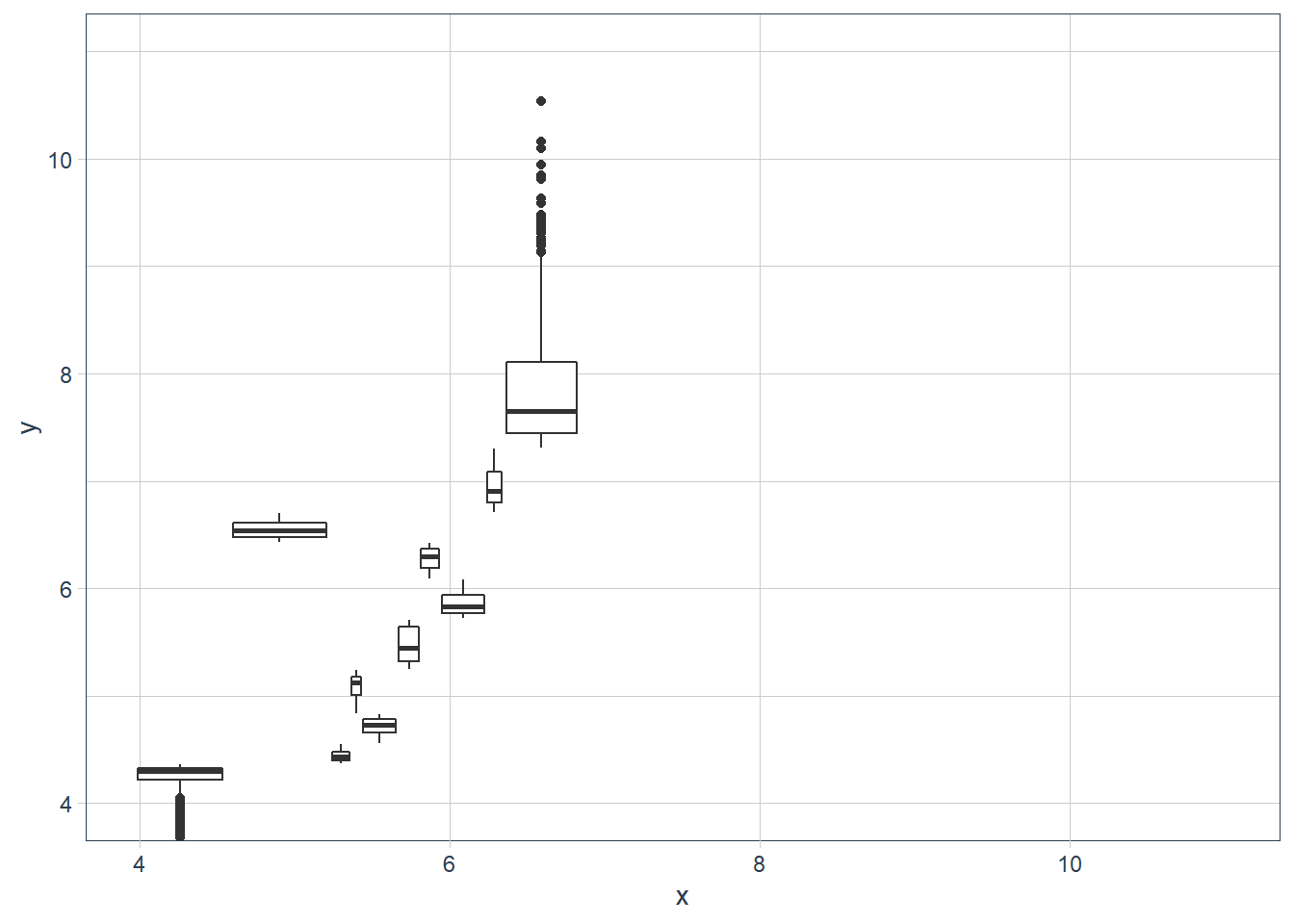Why is a scatterplot a better display than a binned plot for this case?

In a binned plot the points are gathered together in a bin and you may not notice that it is an outlier.

### Patterns

If you spot a pattern, some Qs:

• Could this pattern be due to coincidence (i.e. random chance)?

• How can you describe the relationship implied by the pattern?

• How strong is the relationship implied by the pattern?

• What other variables might affect the relationship?

• Does the relationship change if you look at individual subgroups of the data?

# old faithful eruption lengths vs wait time
ggplot(faithful) +
geom_point(mapping = aes(x = eruptions, y = waiting))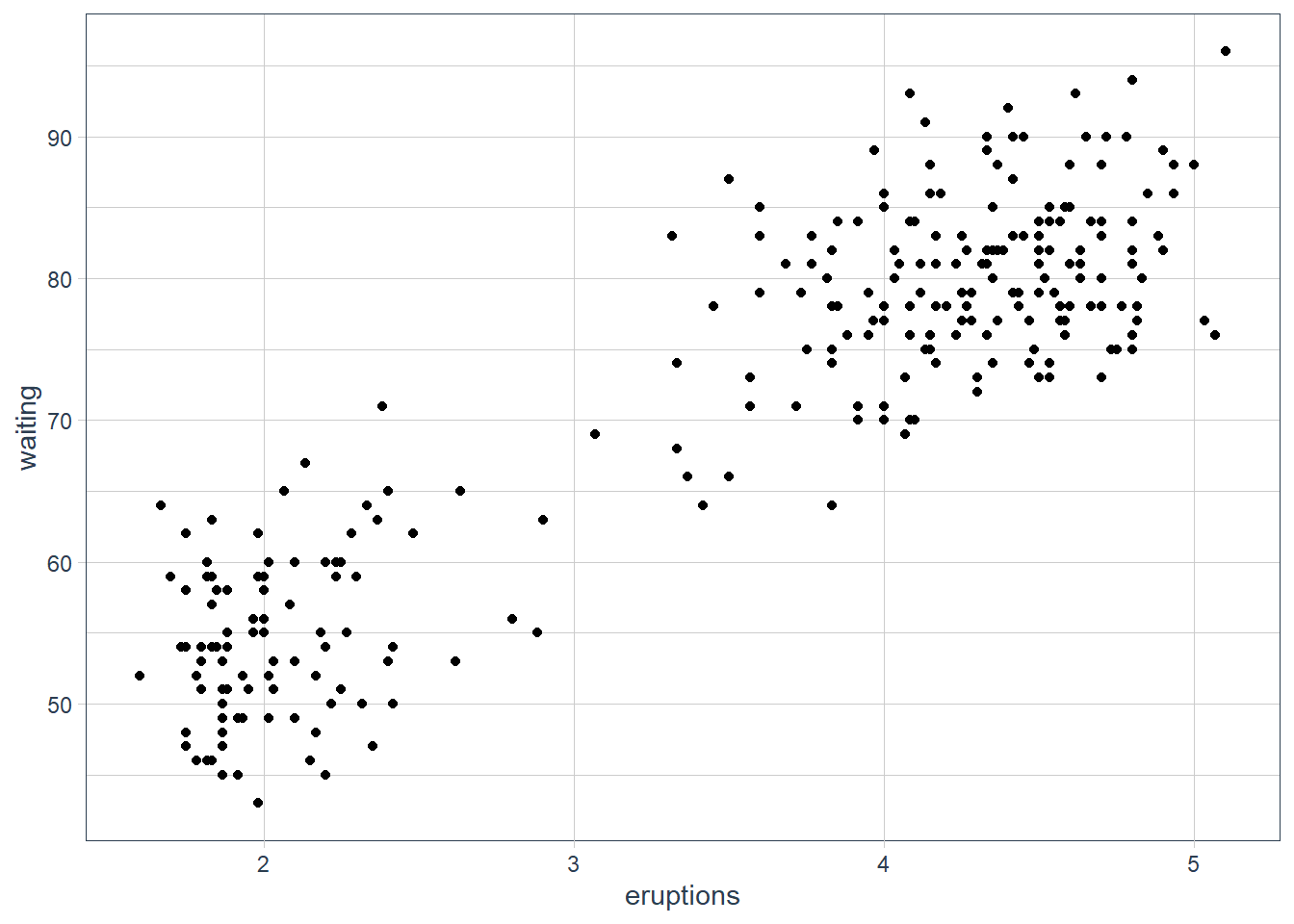sessionInfo()
R version 3.6.3 (2020-02-29)
Platform: x86_64-w64-mingw32/x64 (64-bit)
Running under: Windows 10 x64 (build 18363)

Matrix products: default

locale:
 LC_COLLATE=English_South Africa.1252  LC_CTYPE=English_South Africa.1252
 LC_MONETARY=English_South Africa.1252 LC_NUMERIC=C
 LC_TIME=English_South Africa.1252

attached base packages:
 stats     graphics  grDevices utils     datasets  methods   base

other attached packages:
 ggbeeswarm_0.6.0           lvplot_0.2.0
 magrittr_1.5               tidyquant_1.0.0
 quantmod_0.4.17            TTR_0.23-6
 PerformanceAnalytics_2.0.4 xts_0.12-0
 zoo_1.8-7                  lubridate_1.7.8
 emo_0.0.0.9000             skimr_2.1.1
 gt_0.2.2                   palmerpenguins_0.1.0
 nycflights13_1.0.1         flair_0.0.2
 forcats_0.5.0              stringr_1.4.0
 dplyr_1.0.0                purrr_0.3.4
 tibble_3.0.3               ggplot2_3.3.0
 tidyverse_1.3.0            workflowr_1.6.2

loaded via a namespace (and not attached):
 nlme_3.1-144       fs_1.4.1           RColorBrewer_1.1-2 httr_1.4.2
 rprojroot_1.3-2    repr_1.1.0         tools_3.6.3        backports_1.1.6
 utf8_1.1.4         R6_2.4.1           vipor_0.4.5        DBI_1.1.0
 colorspace_1.4-1   withr_2.2.0        tidyselect_1.1.0   curl_4.3
 compiler_3.6.3     git2r_0.26.1       cli_2.0.2          rvest_0.3.5
 xml2_1.3.2         labeling_0.3       sass_0.2.0         scales_1.1.0
 ellipsis_0.3.1